# R S Aggarwal Solutions for Class 10 Maths Chapter 18 Mean, Median, Mode of Grouped Data, Cumulative Frequency Graph and Ogive

R S Aggarwal Solutions for Class 10 Maths Chapter 18 Mean, Median, Mode of Grouped Data, Cumulative Frequency Graph and Ogive is one of the most important concepts in Class 10. Chapter 18 of class 10 Maths mainly deals with problems based on mean, median, mode and graphical representation of the data. Students must practice R S Aggarwal Solutions for Maths to score well in exams.

## Download PDF of R S Aggarwal Solutions for Class 10 Maths Chapter 18 Mean, Median, Mode of Grouped Data, Cumulative Frequency Graph and Ogive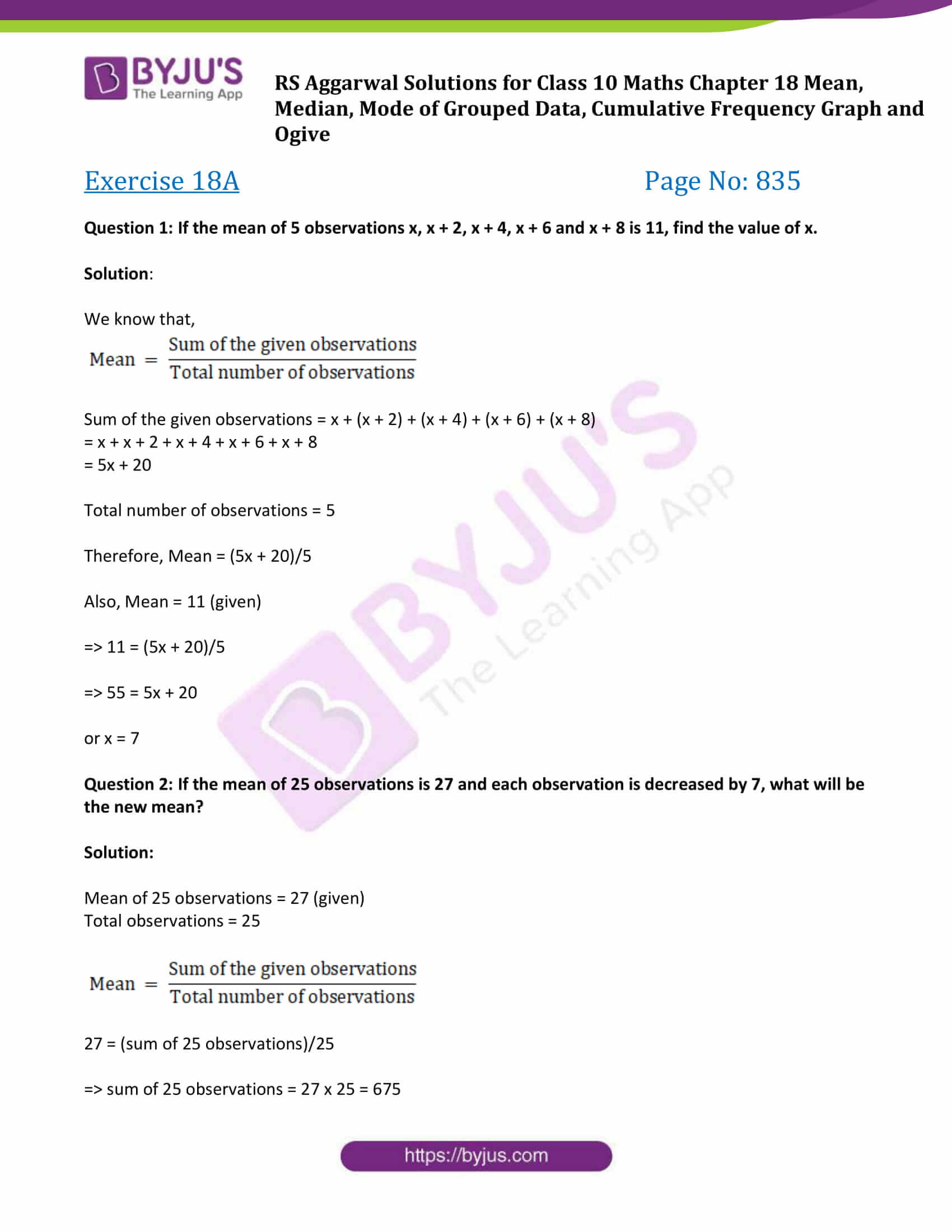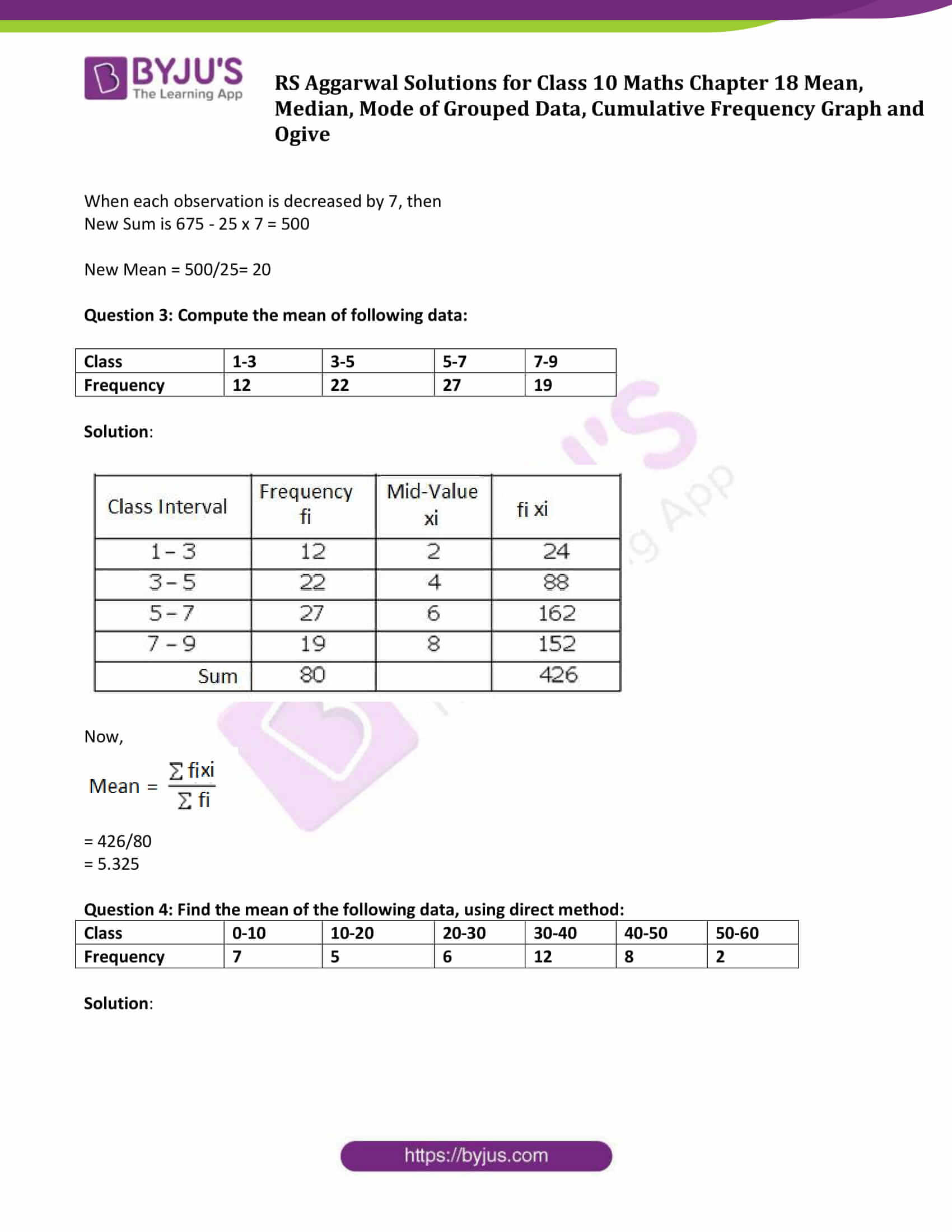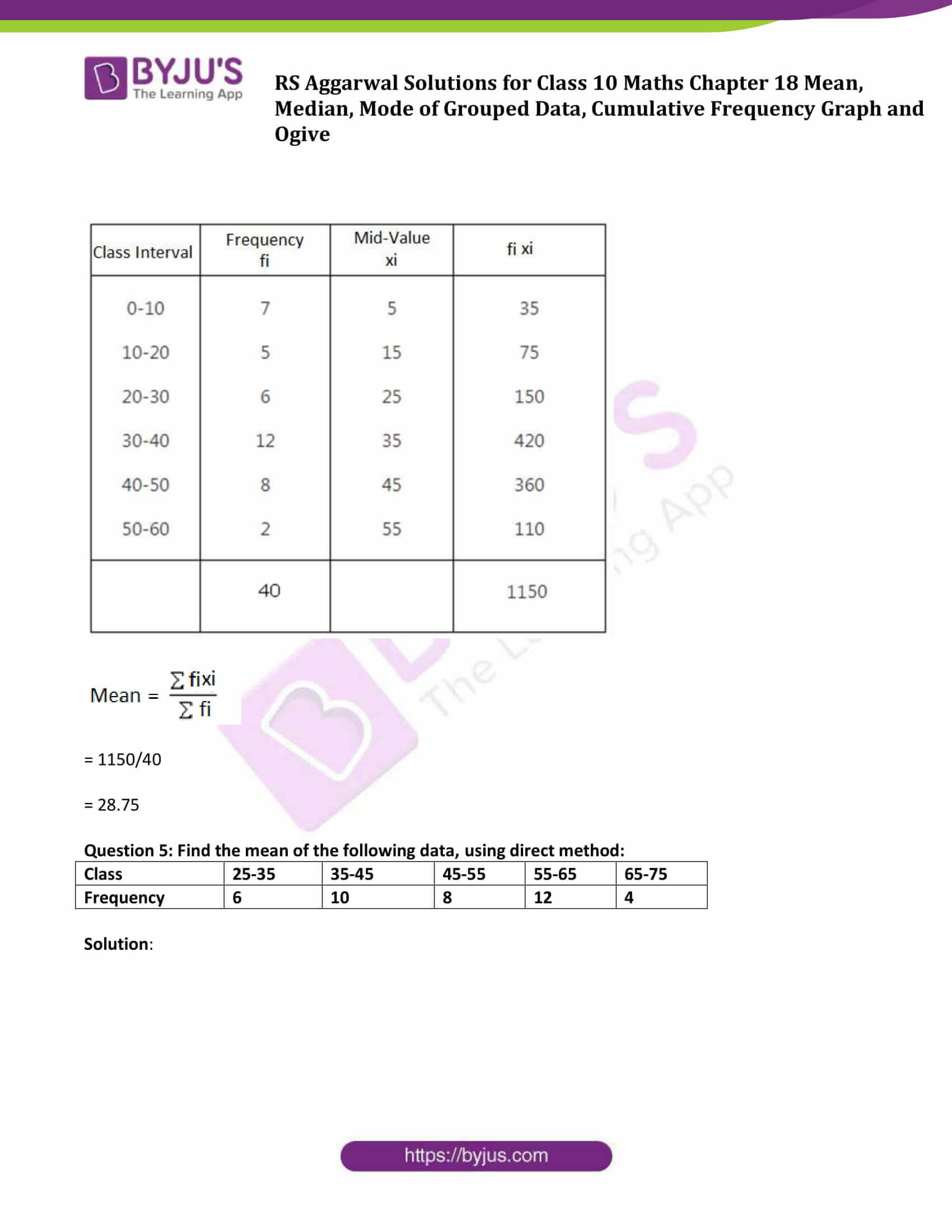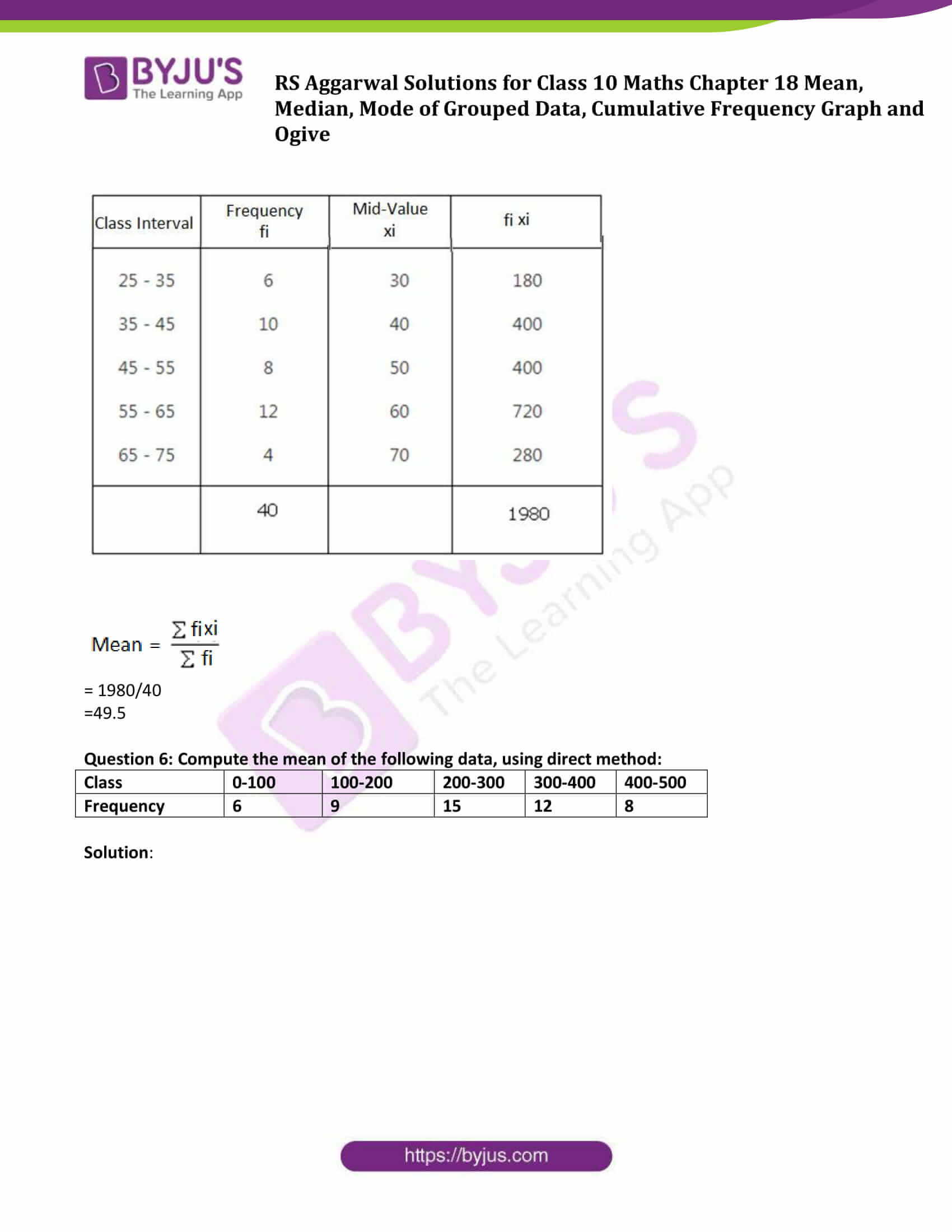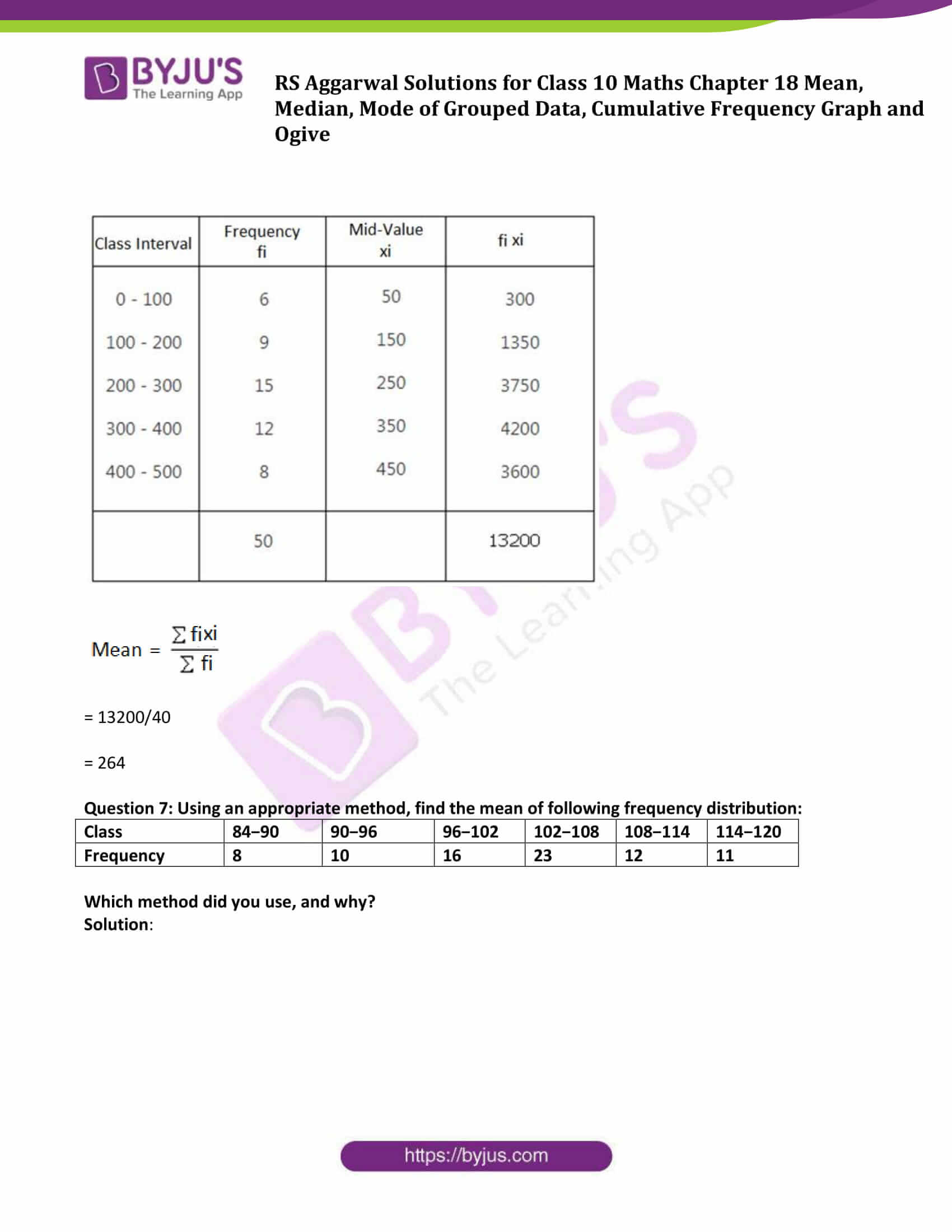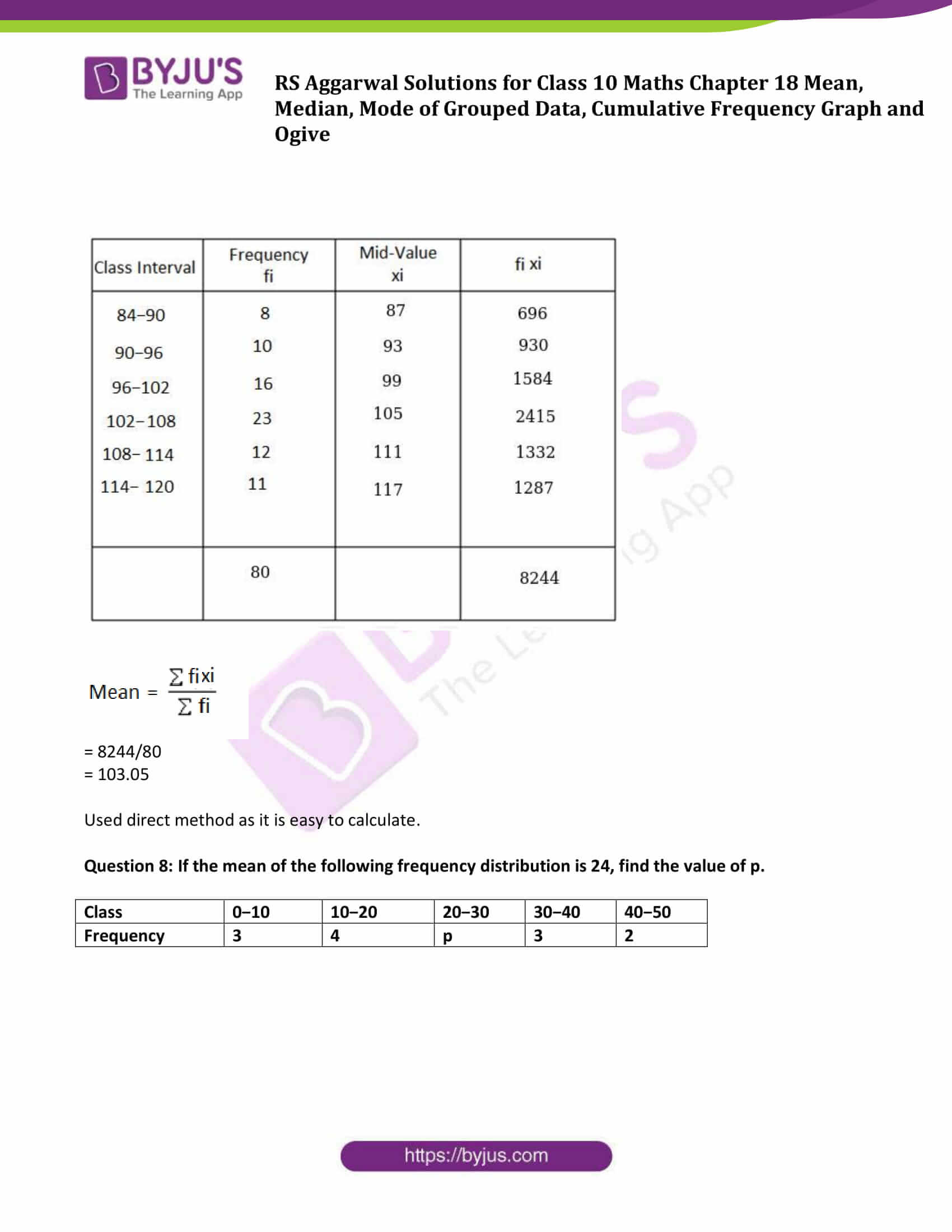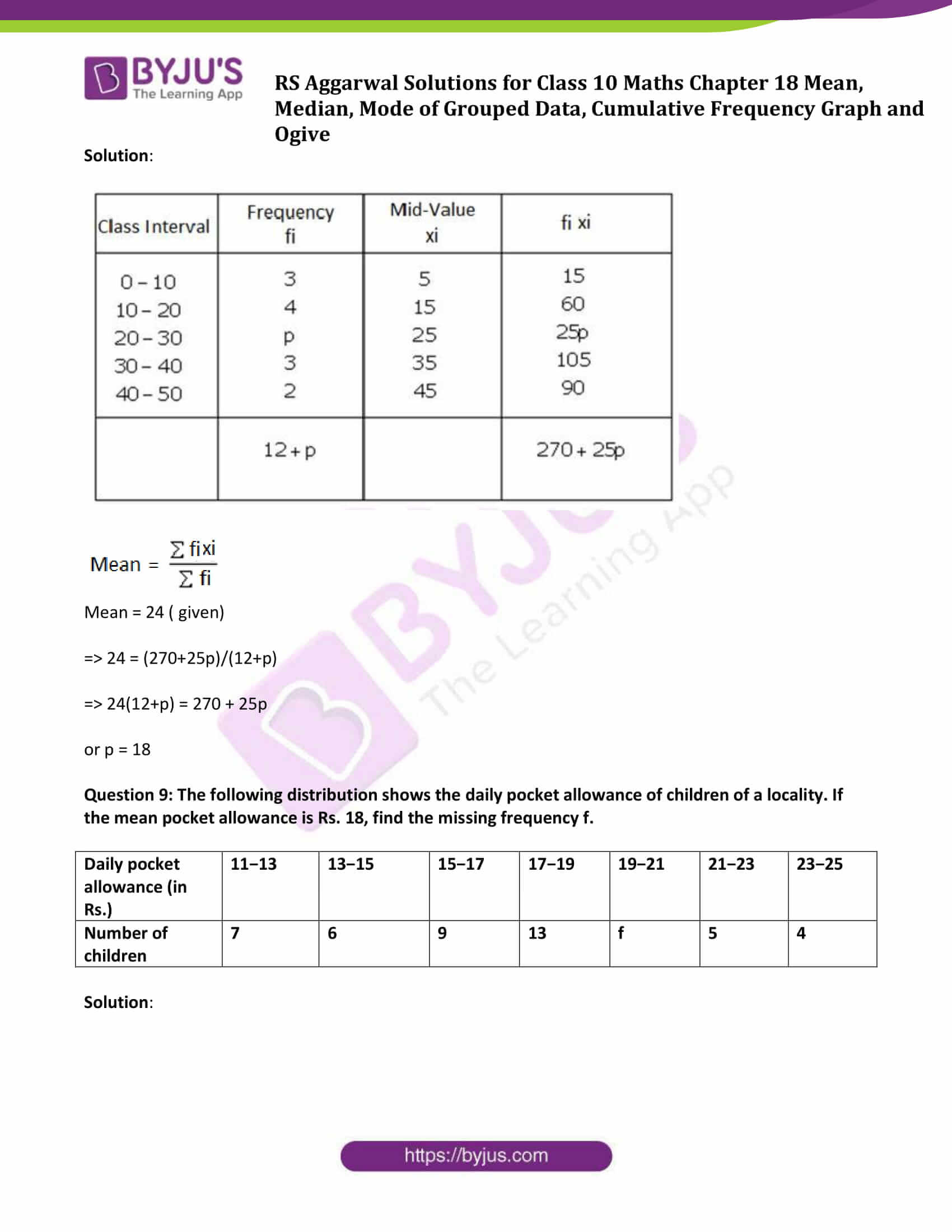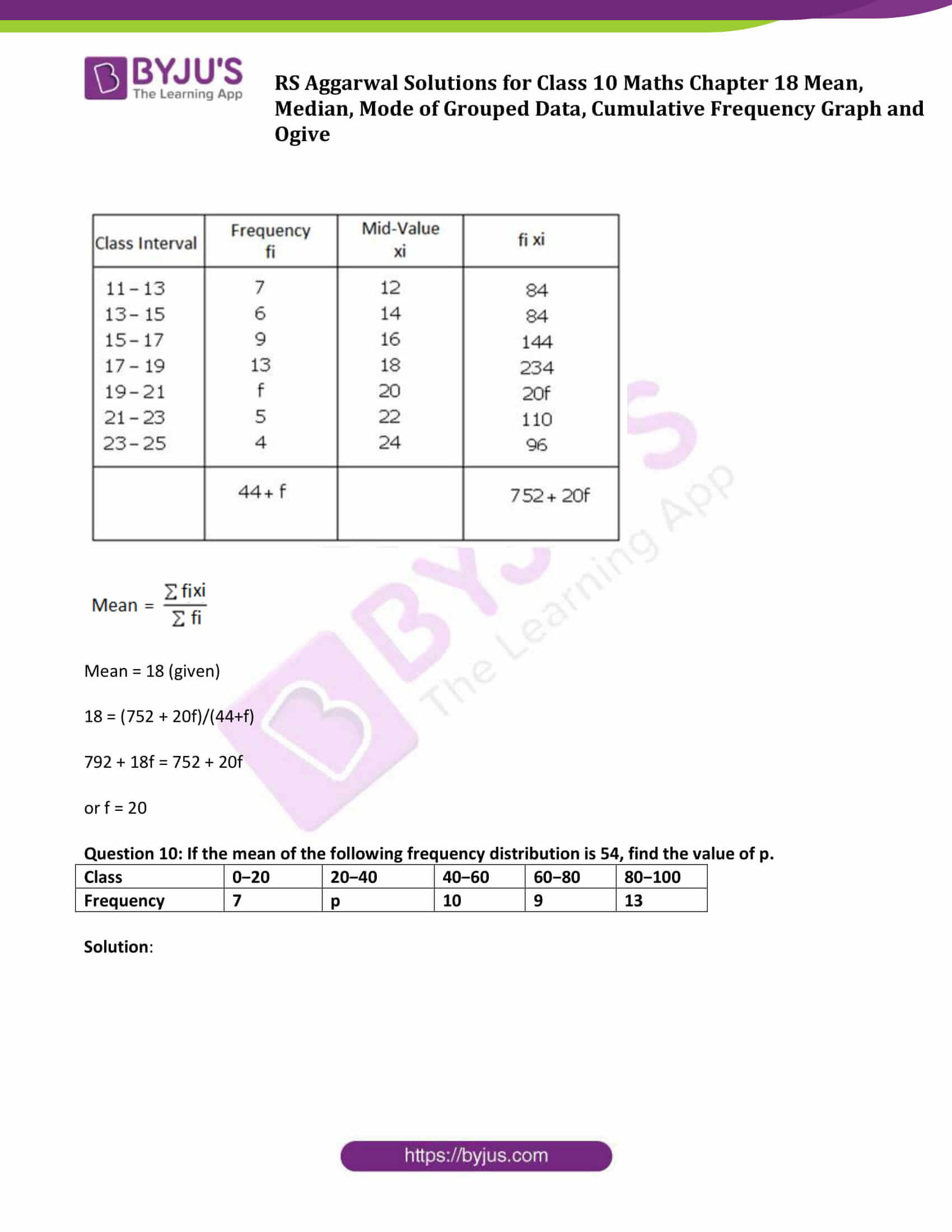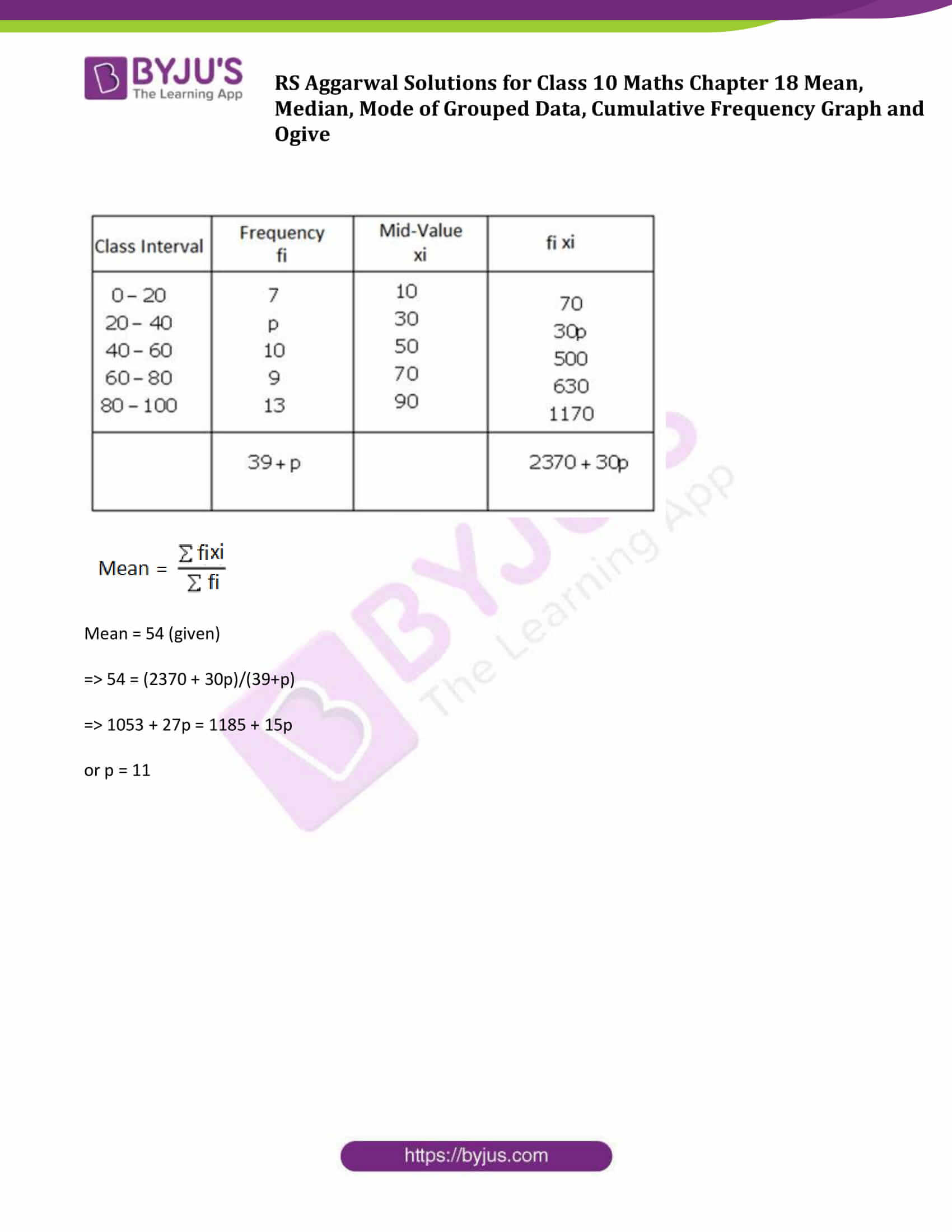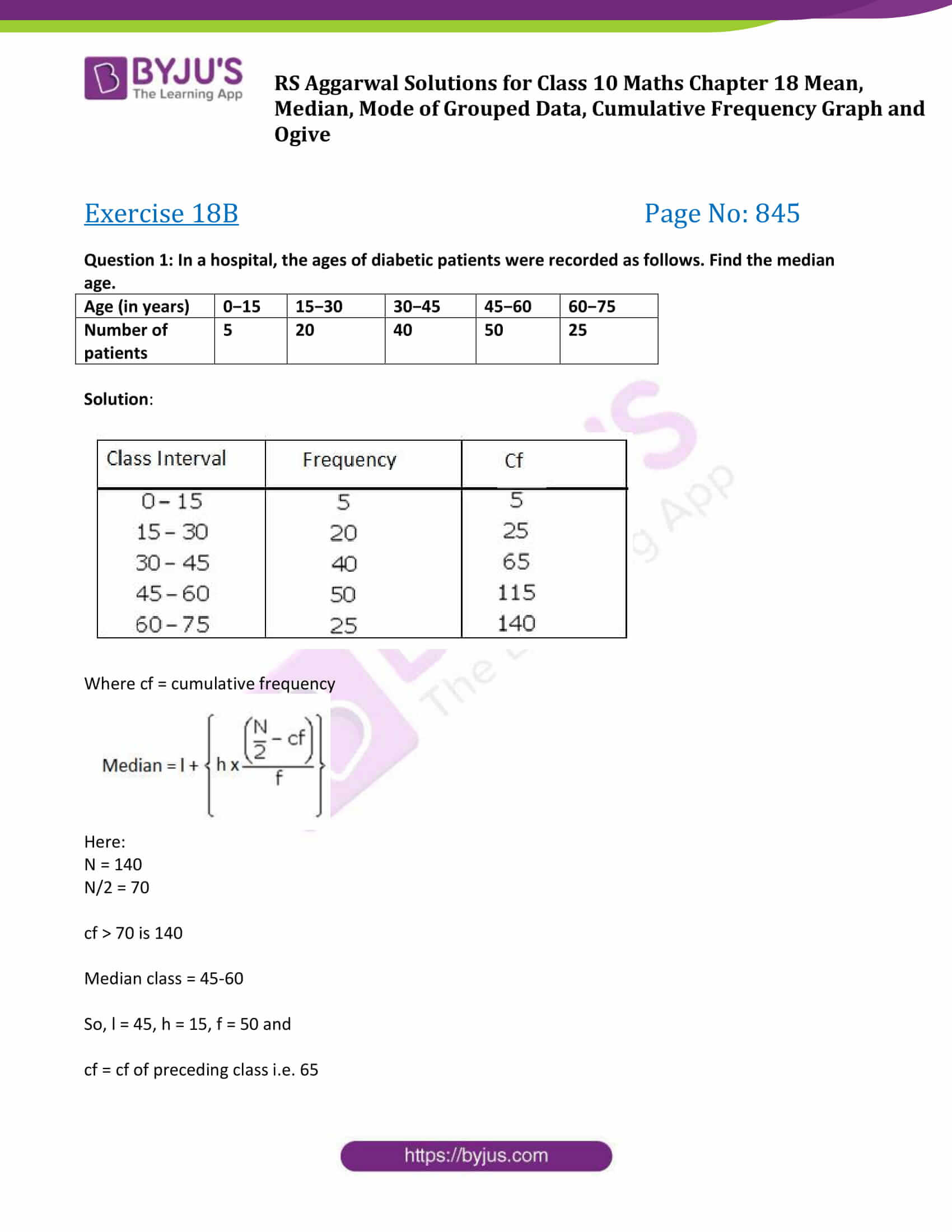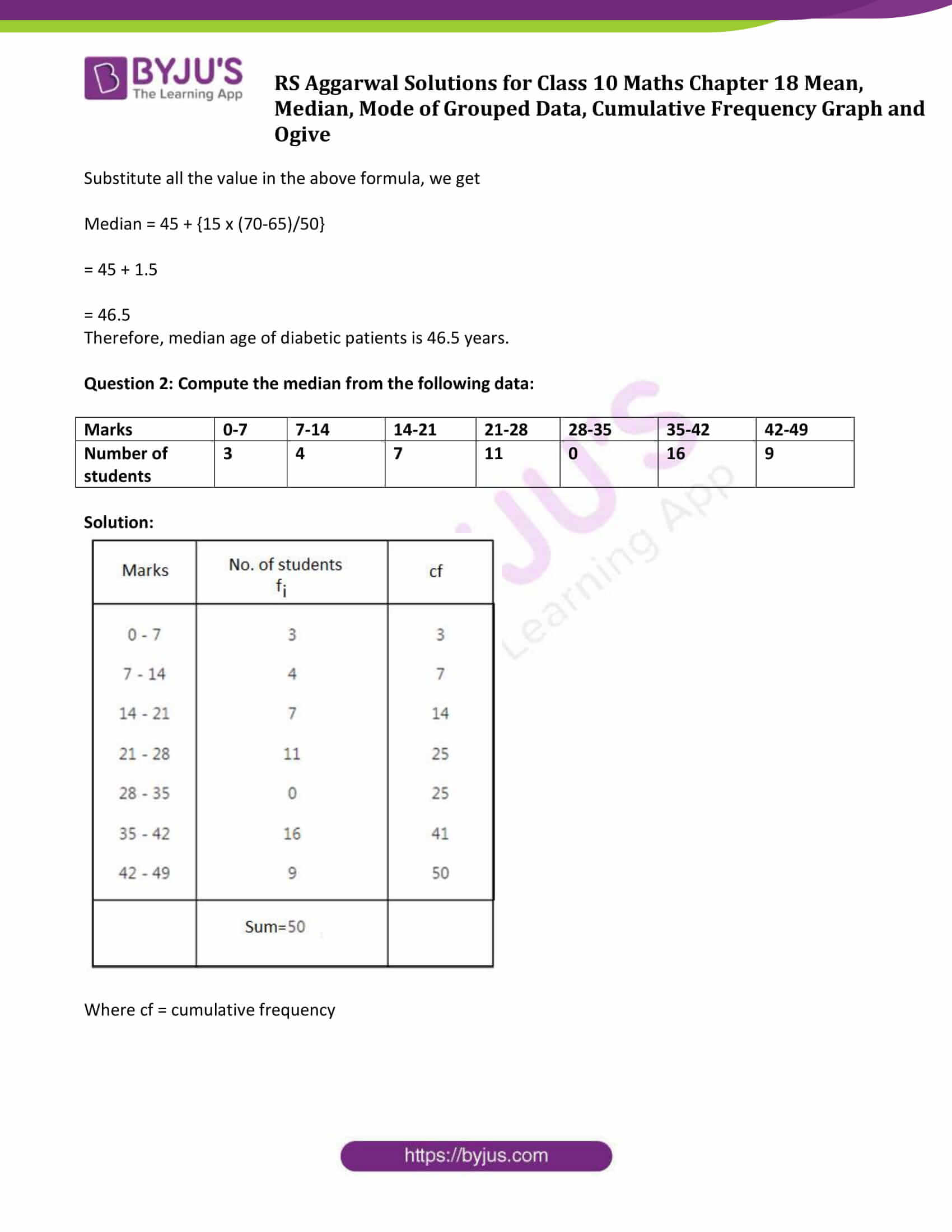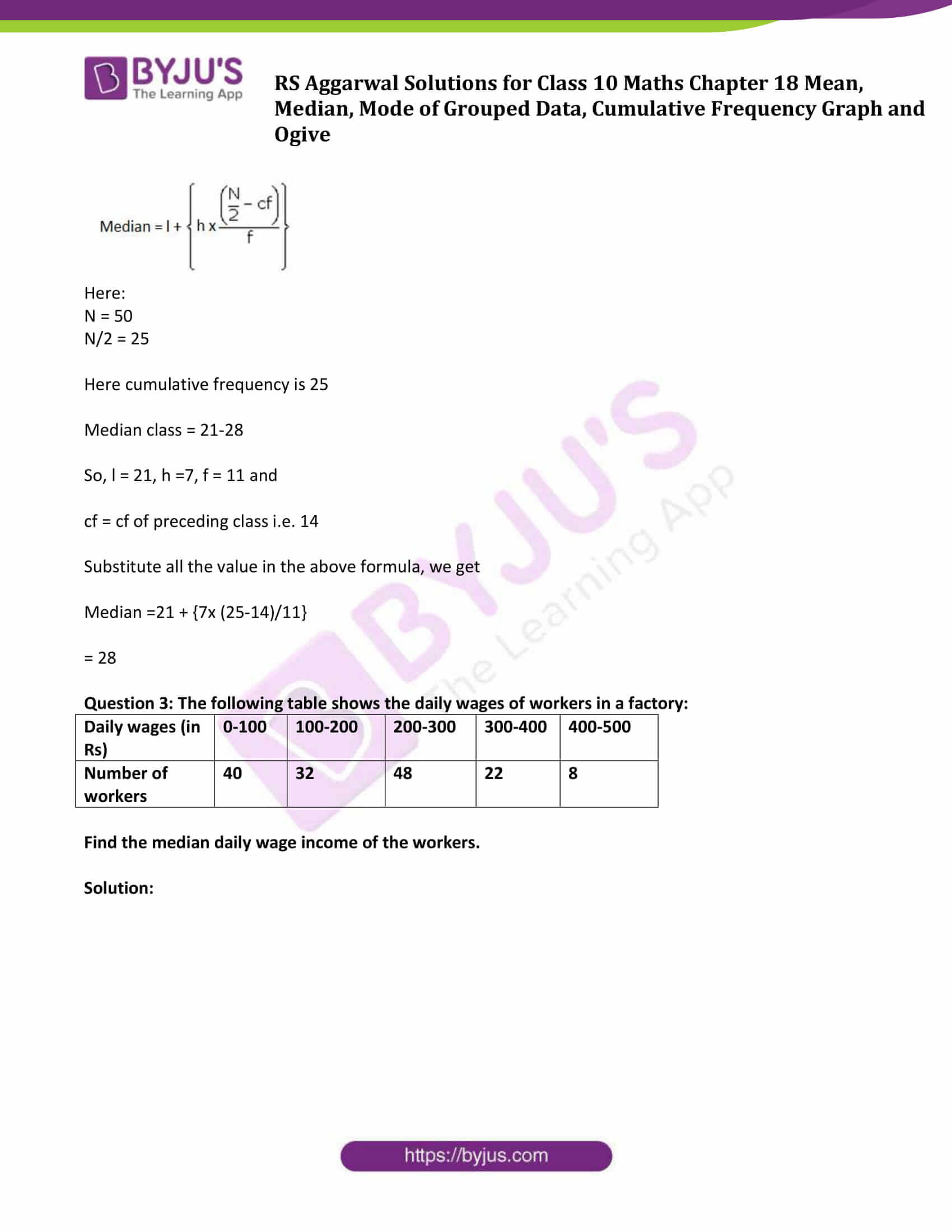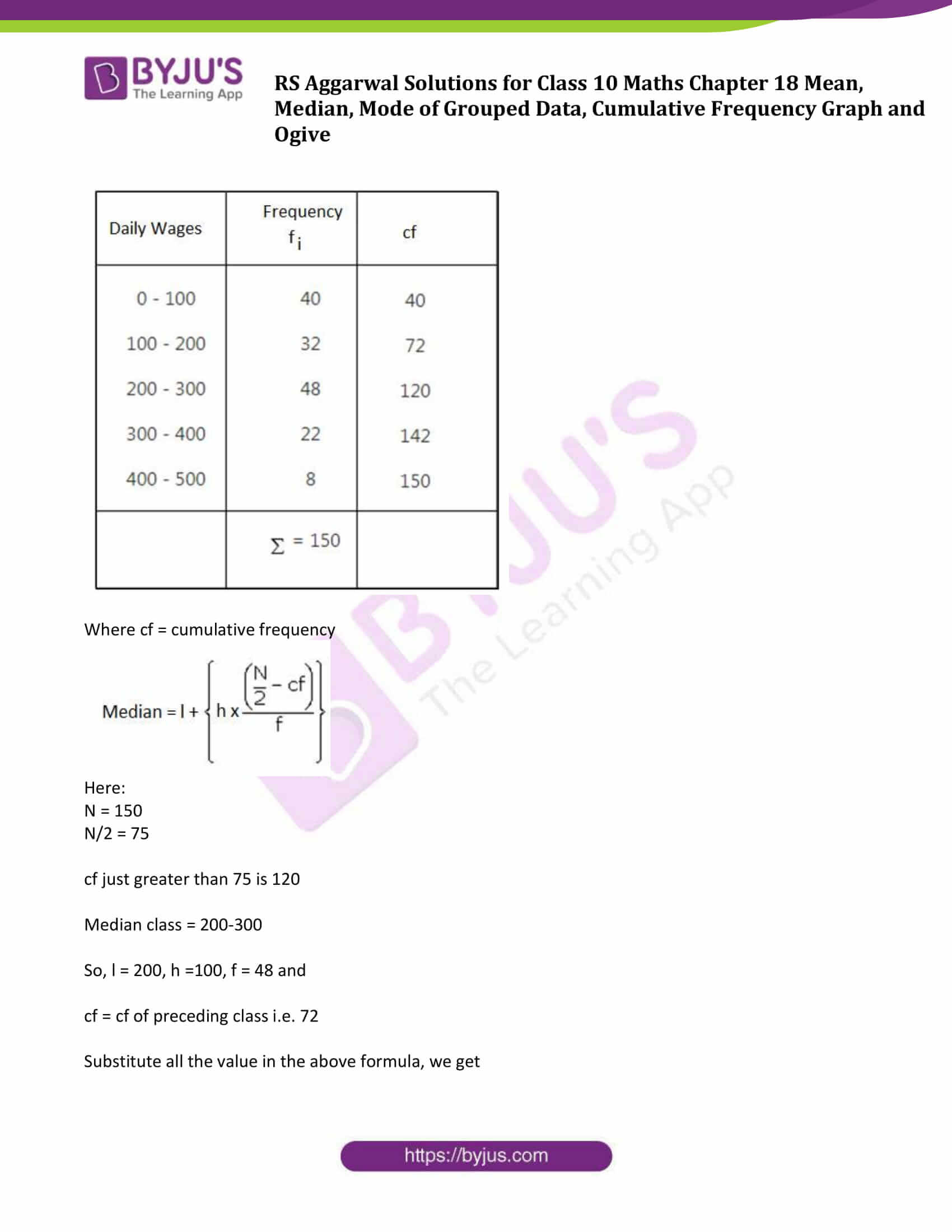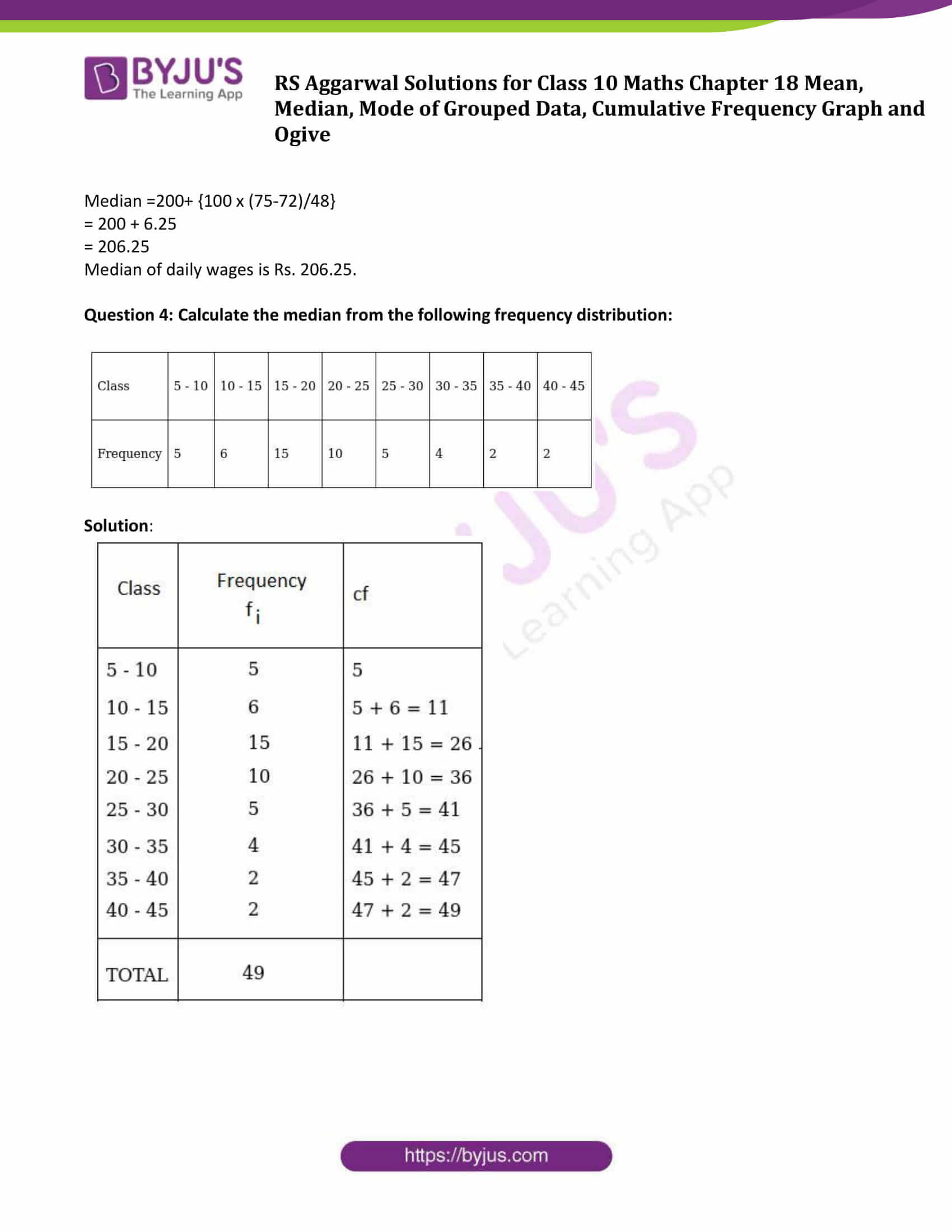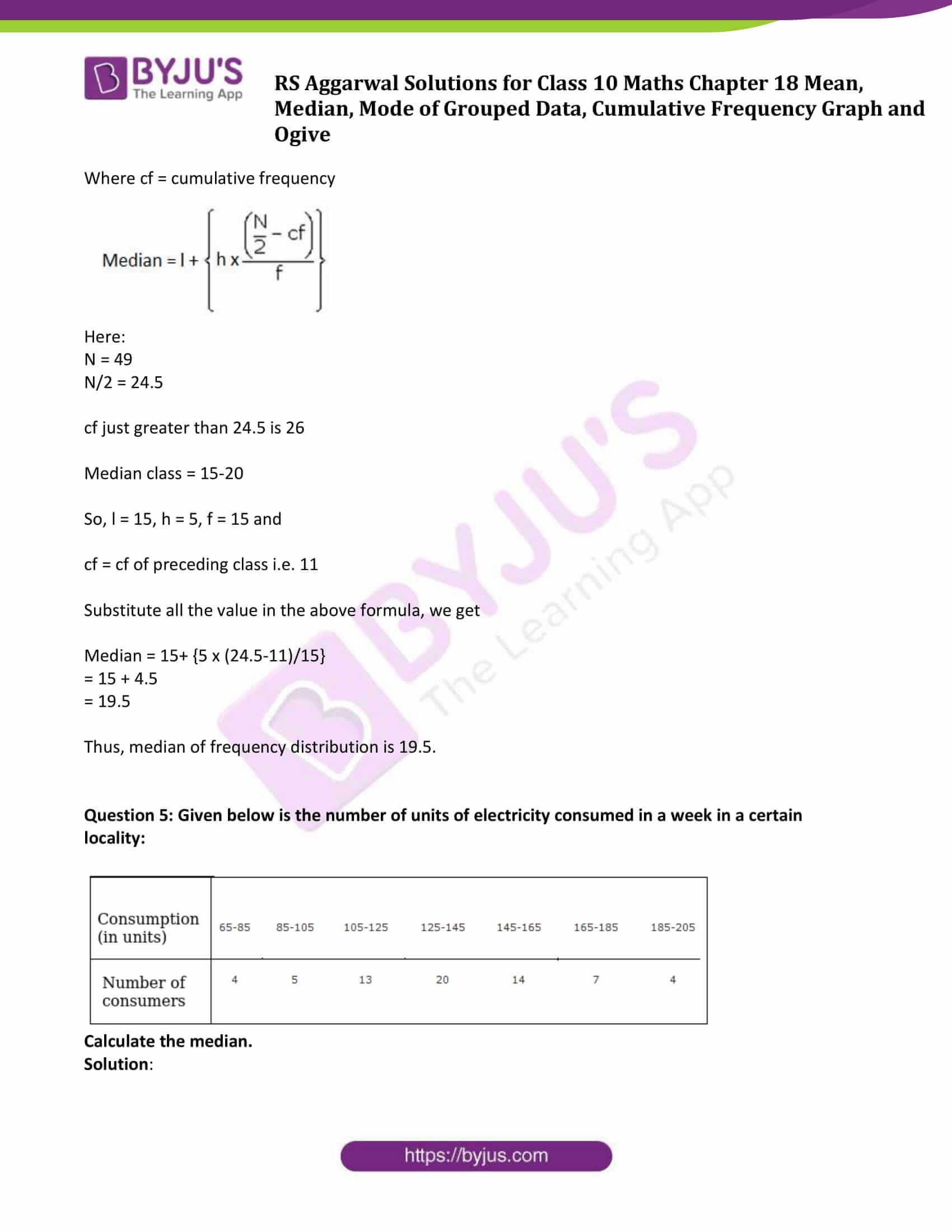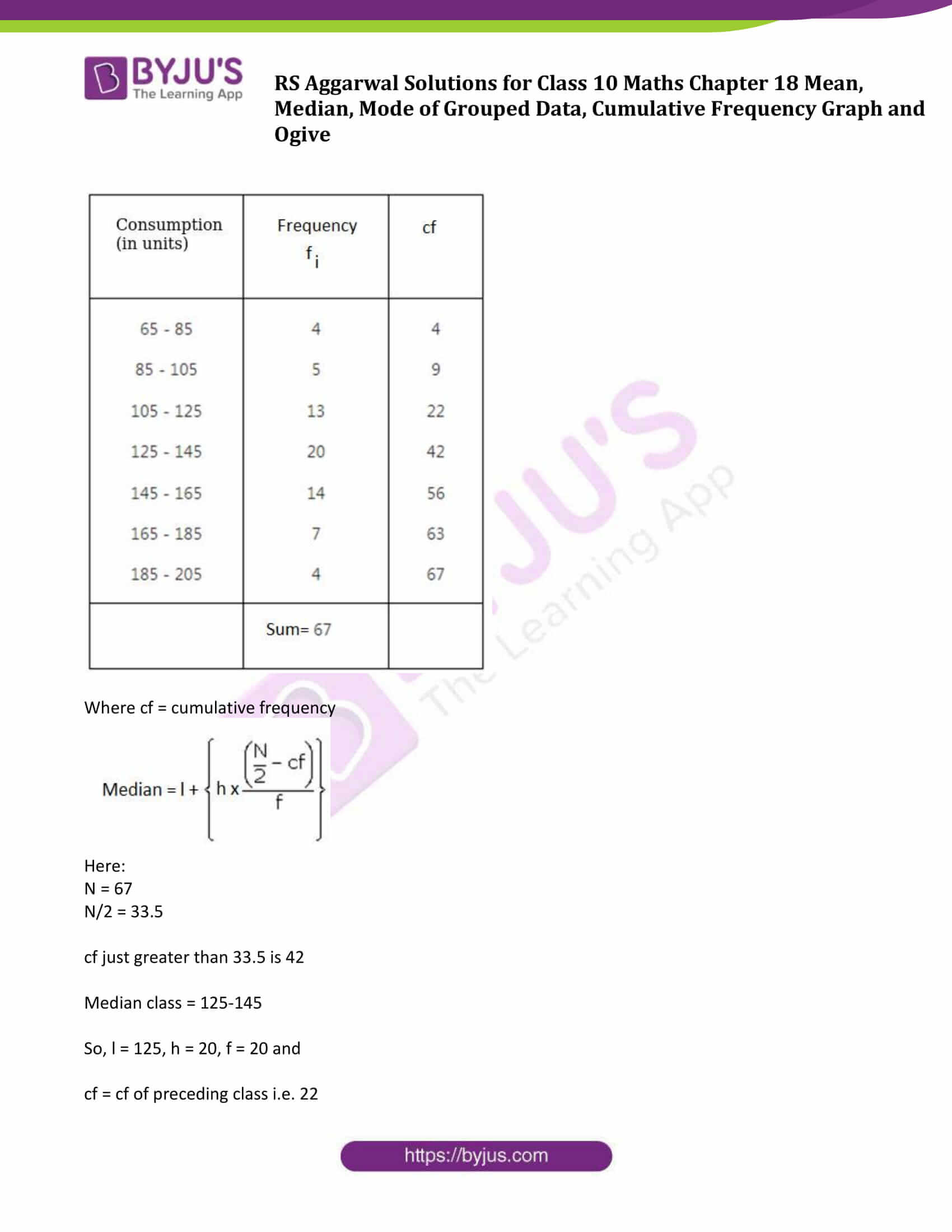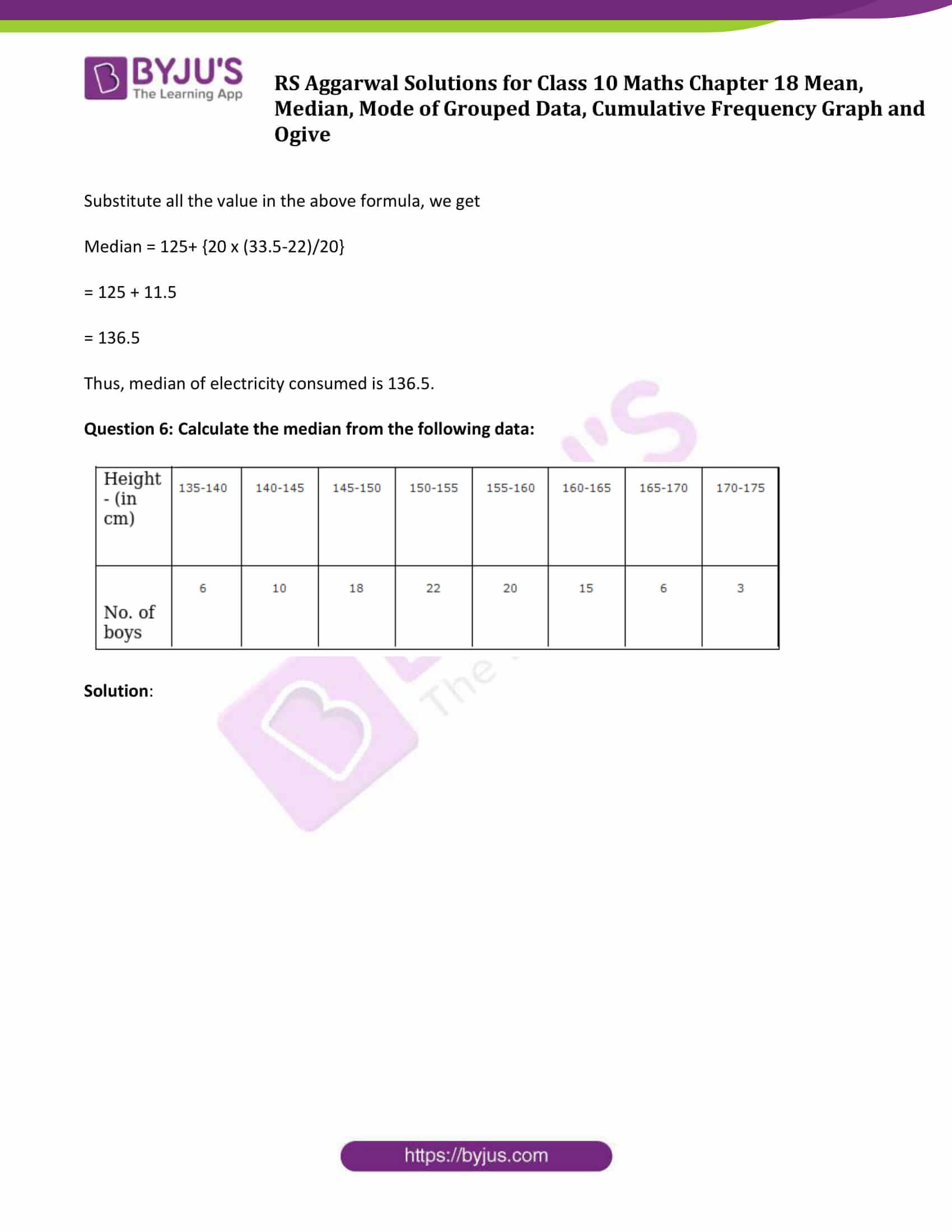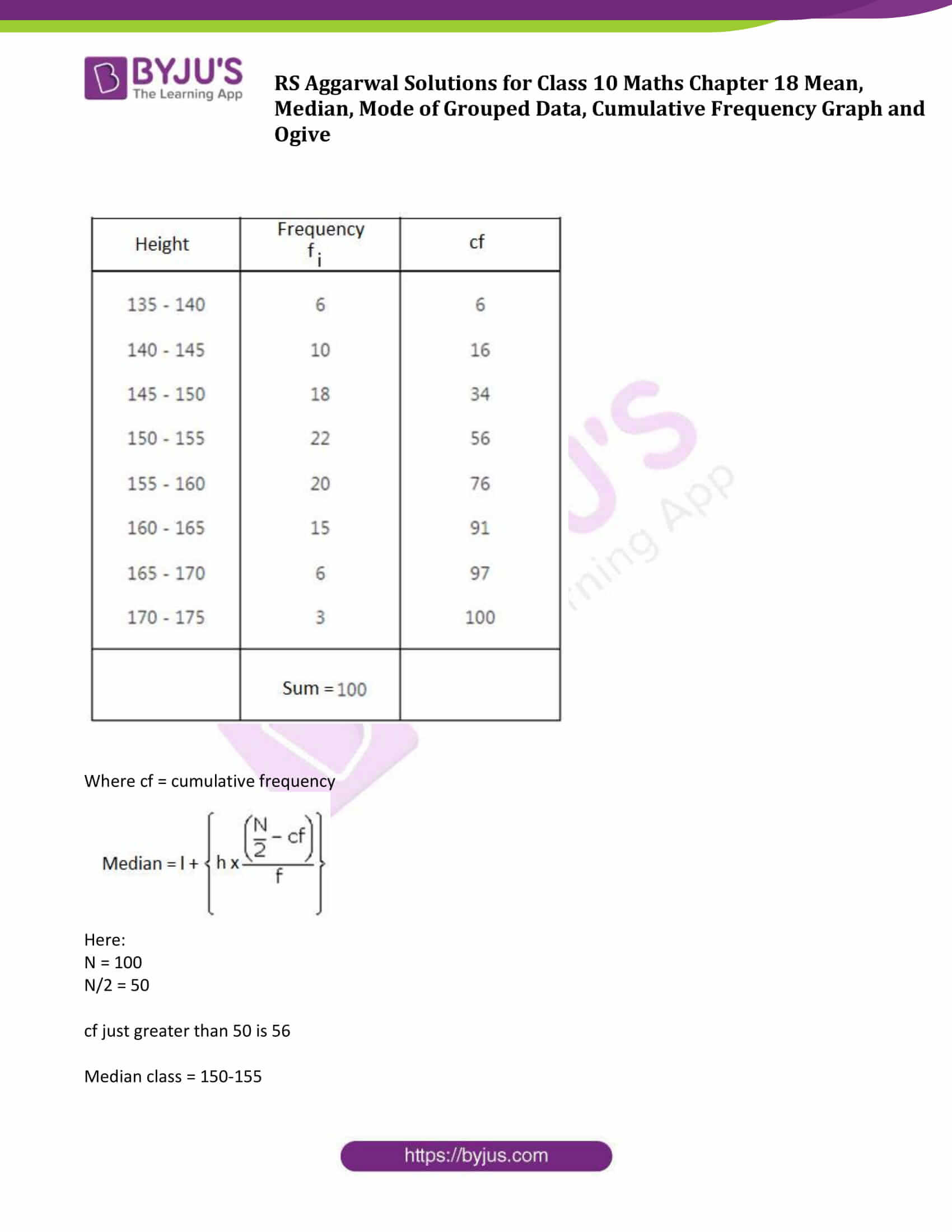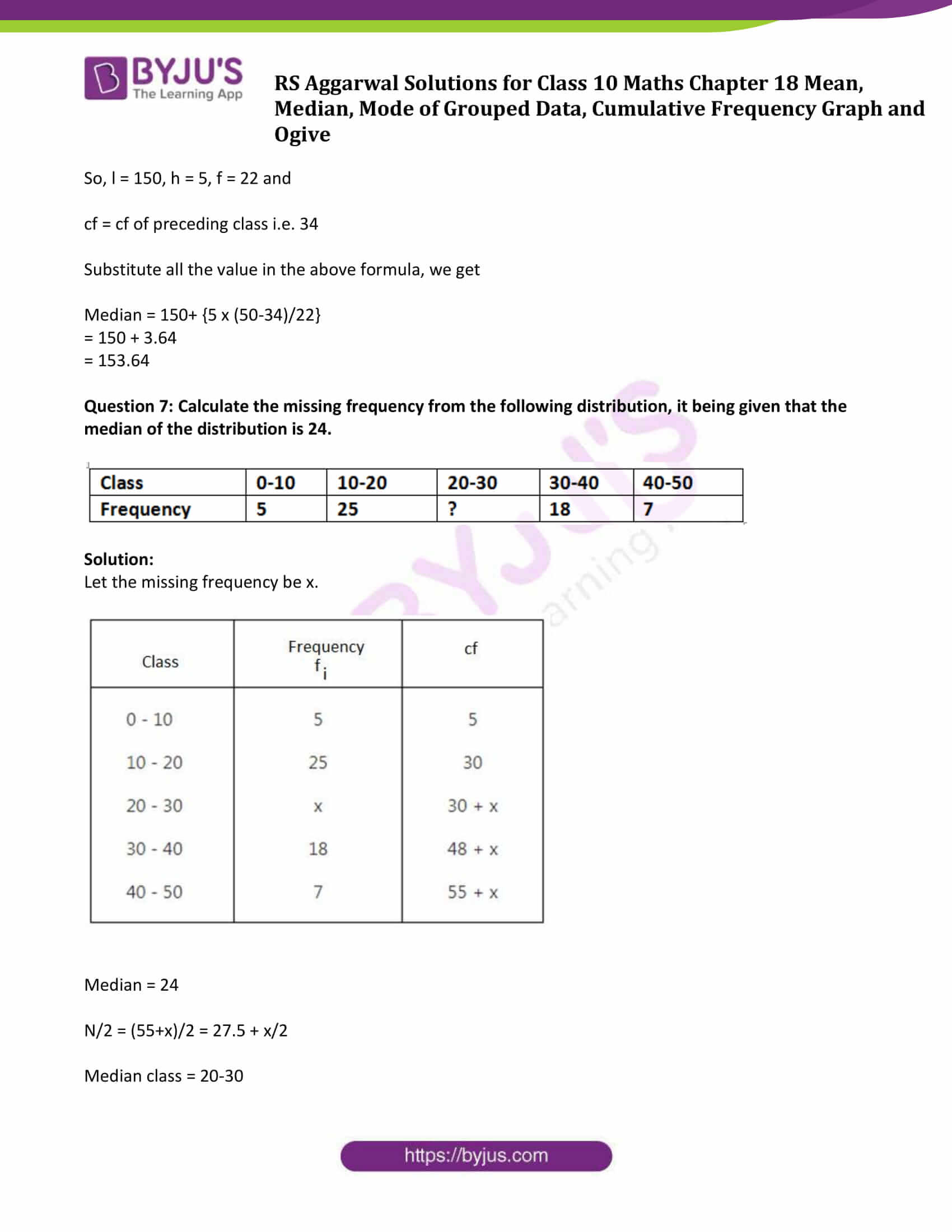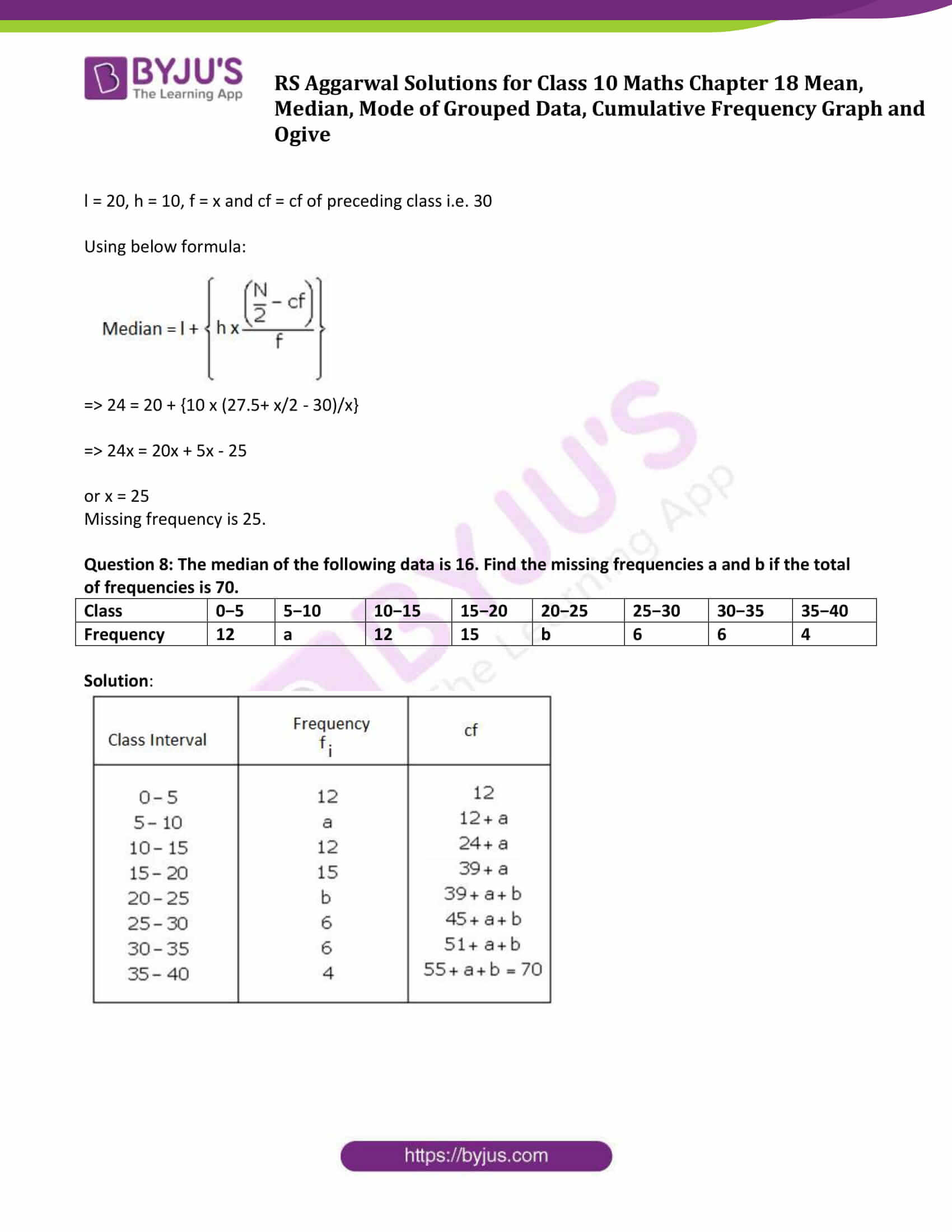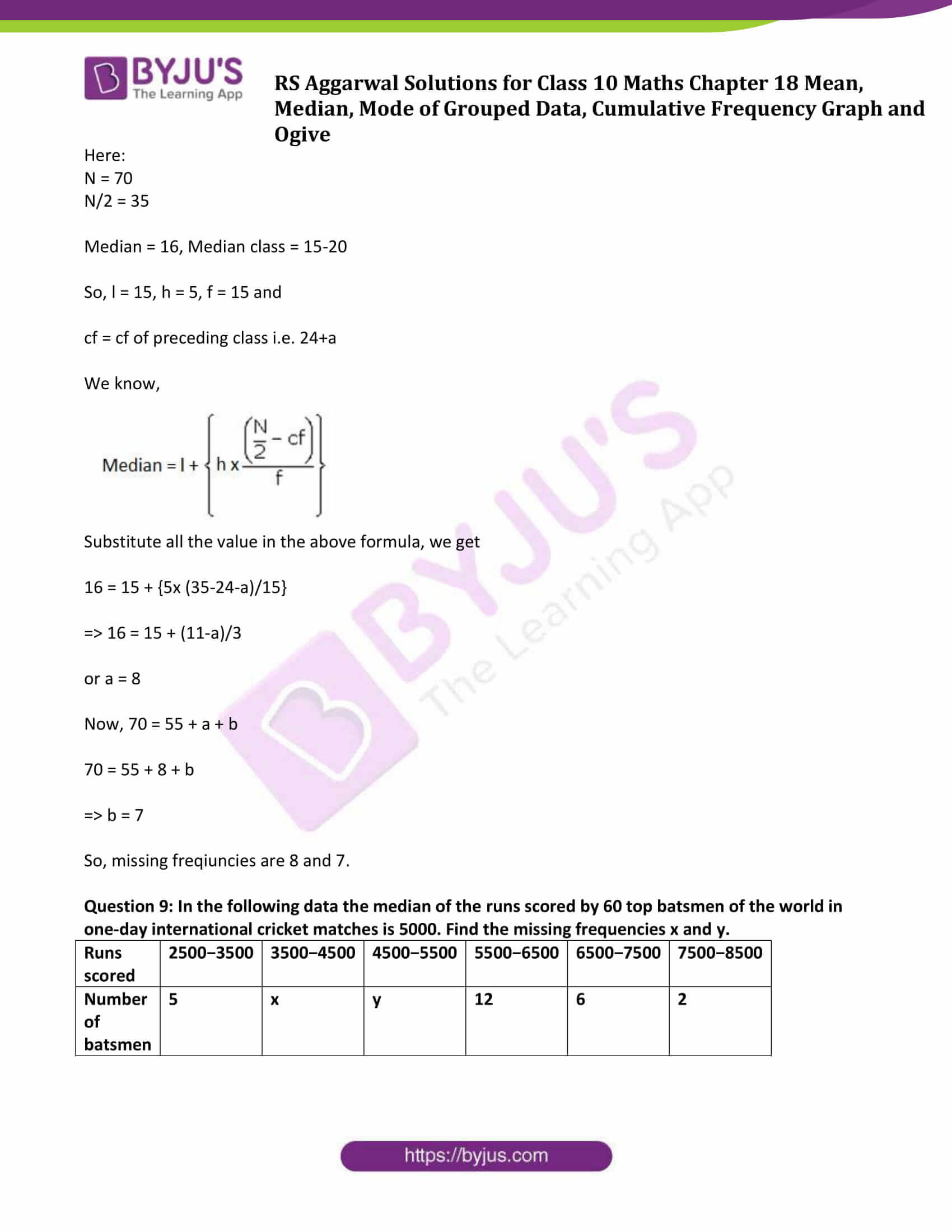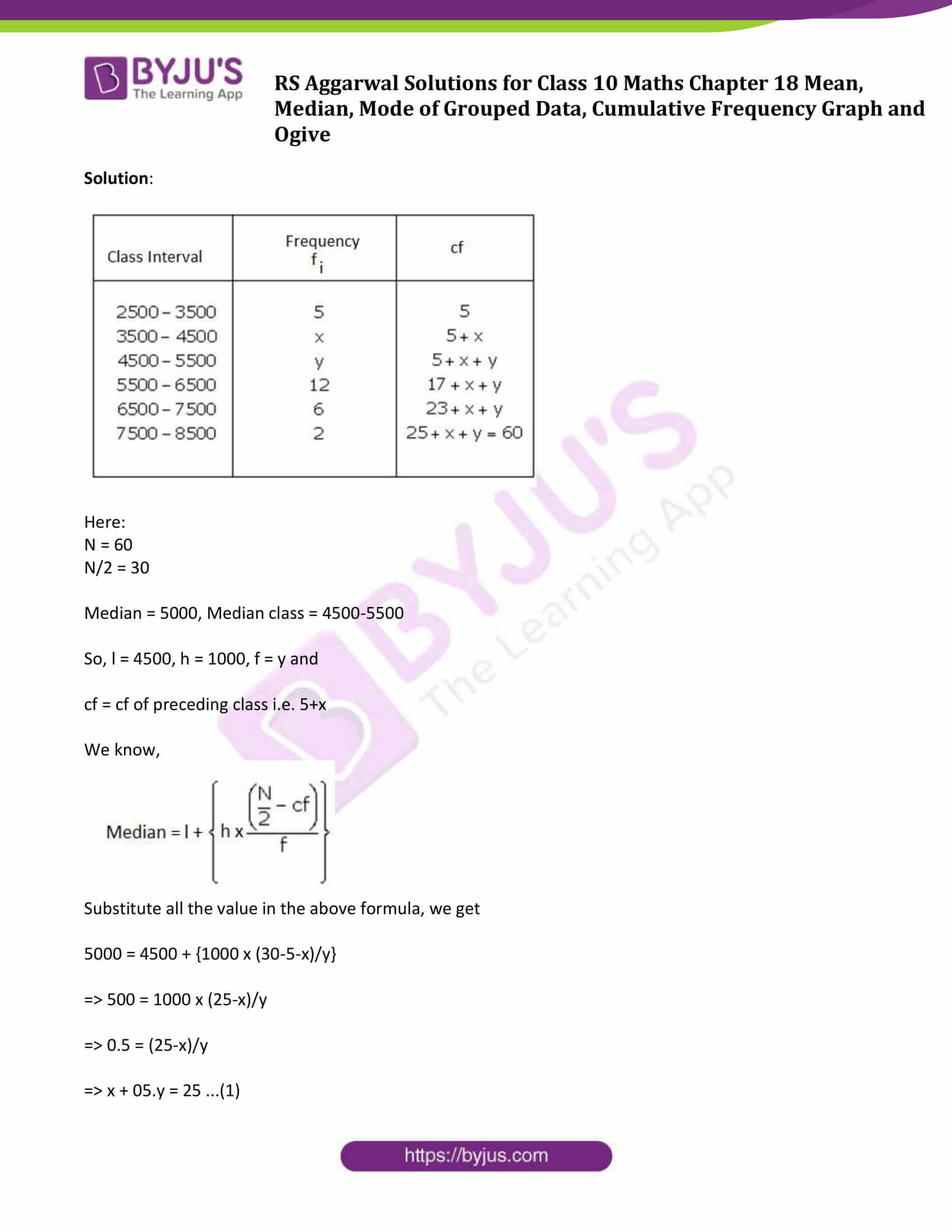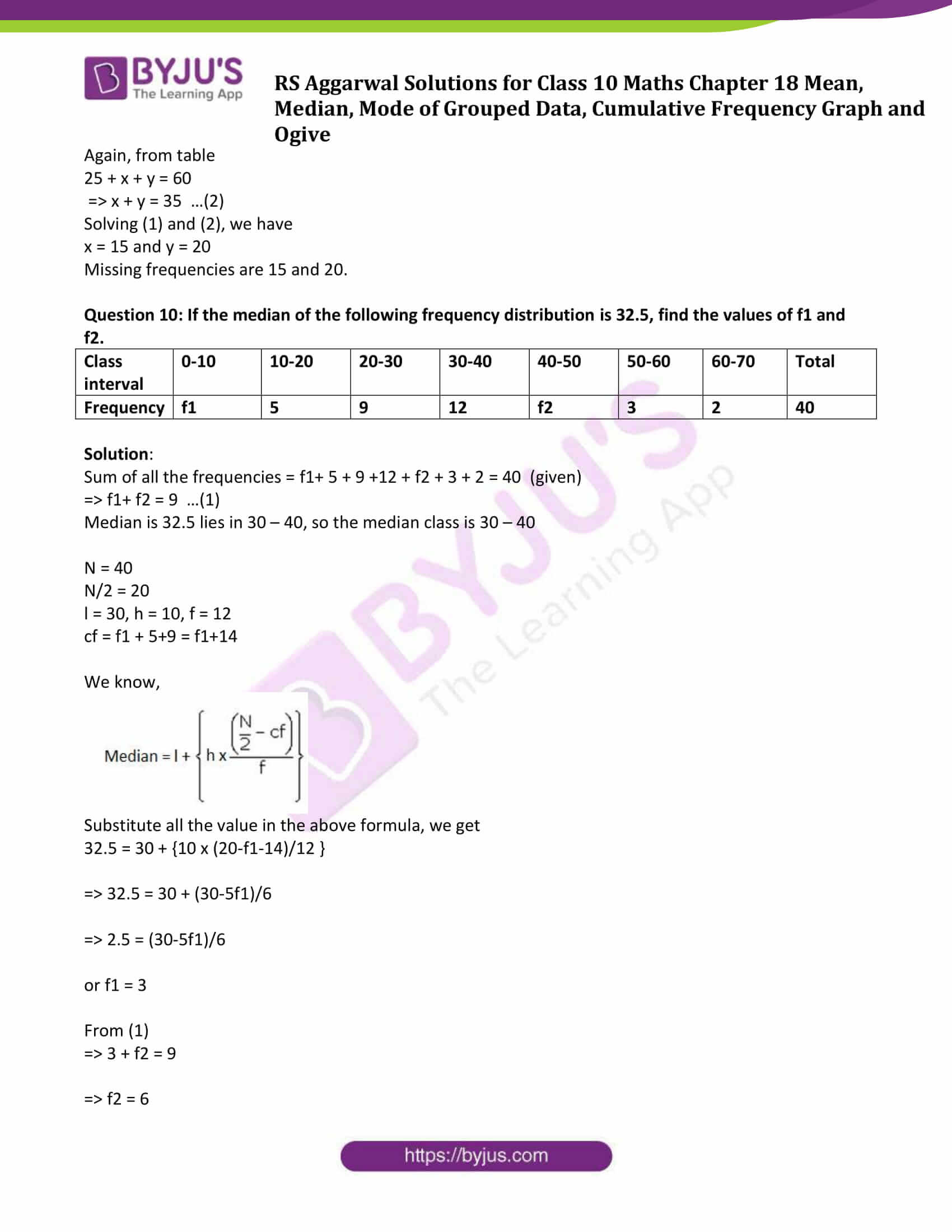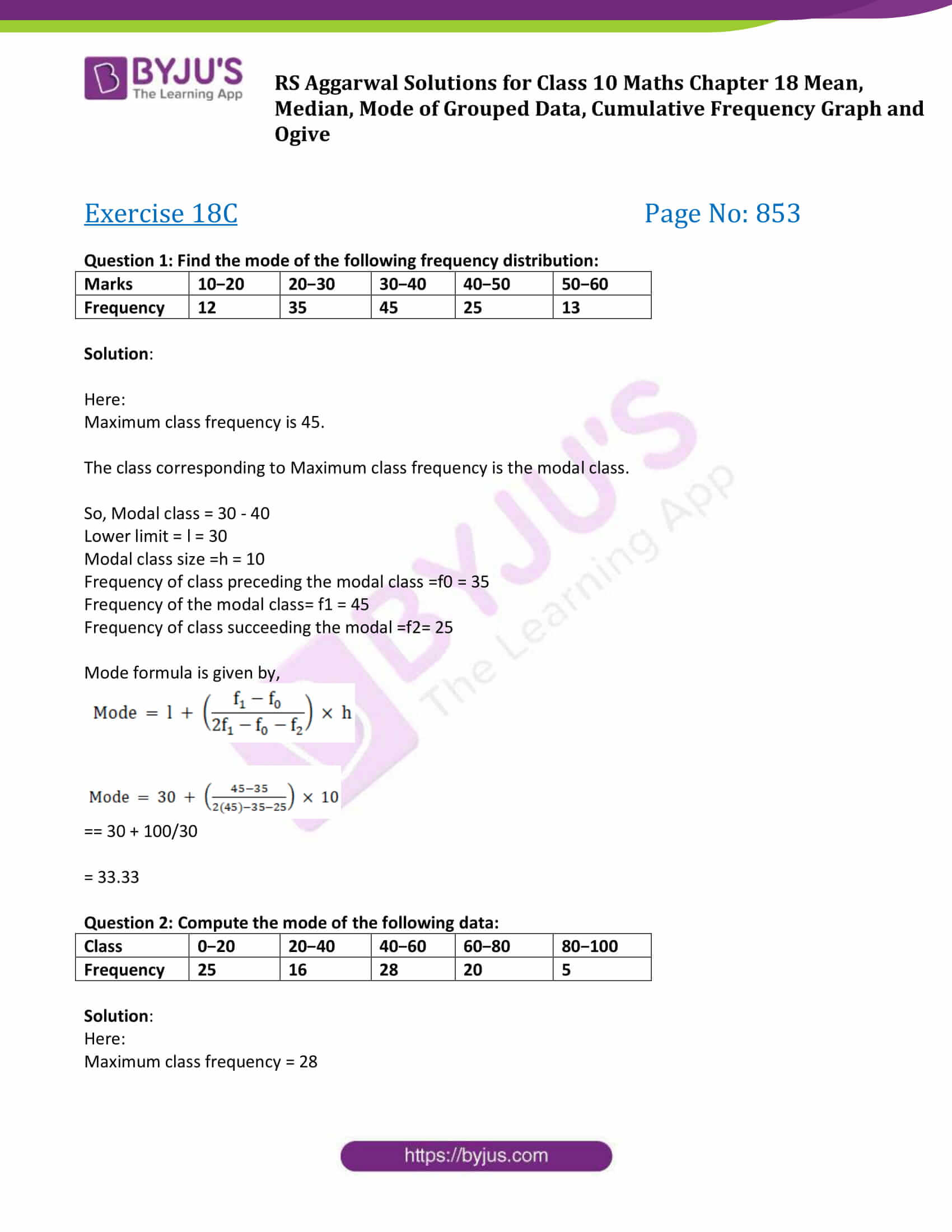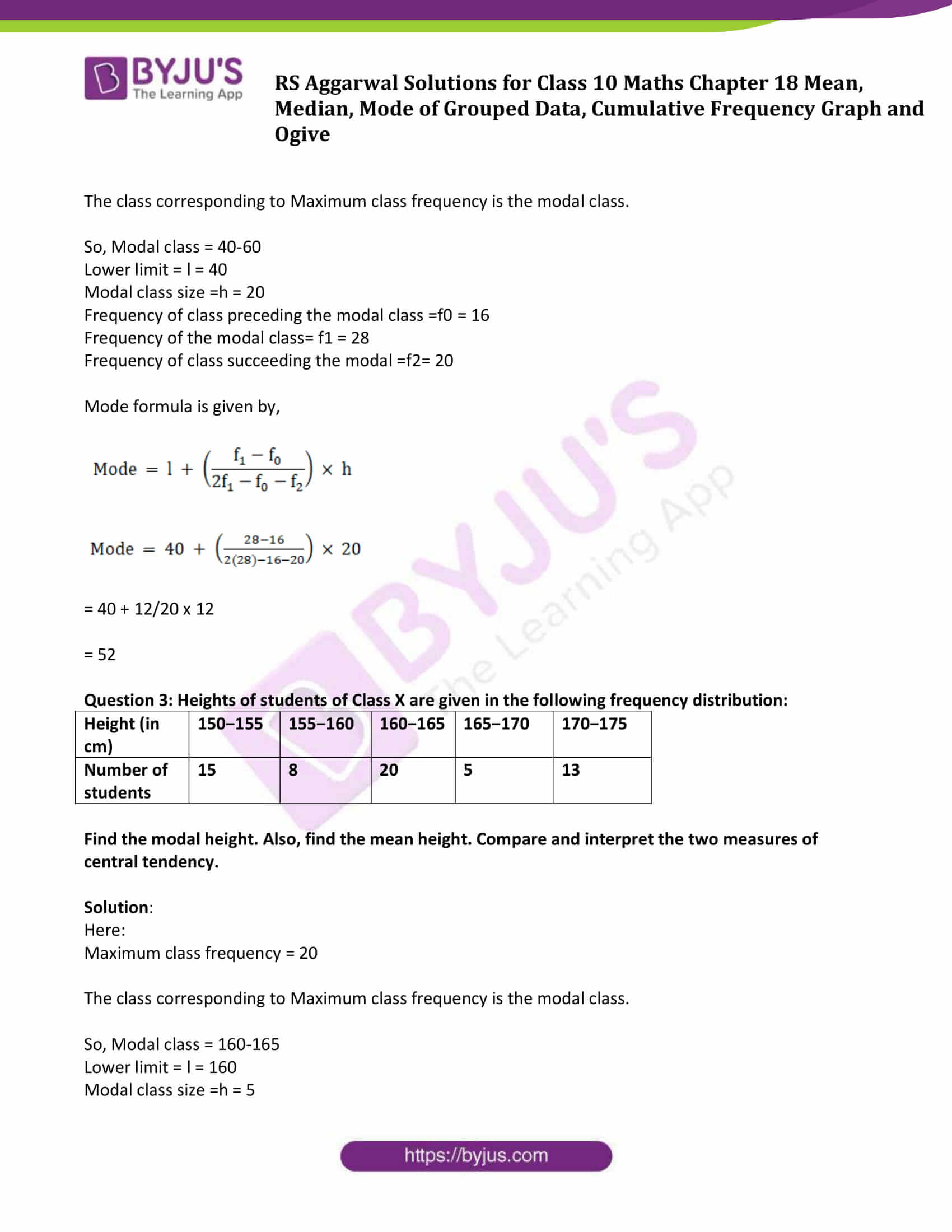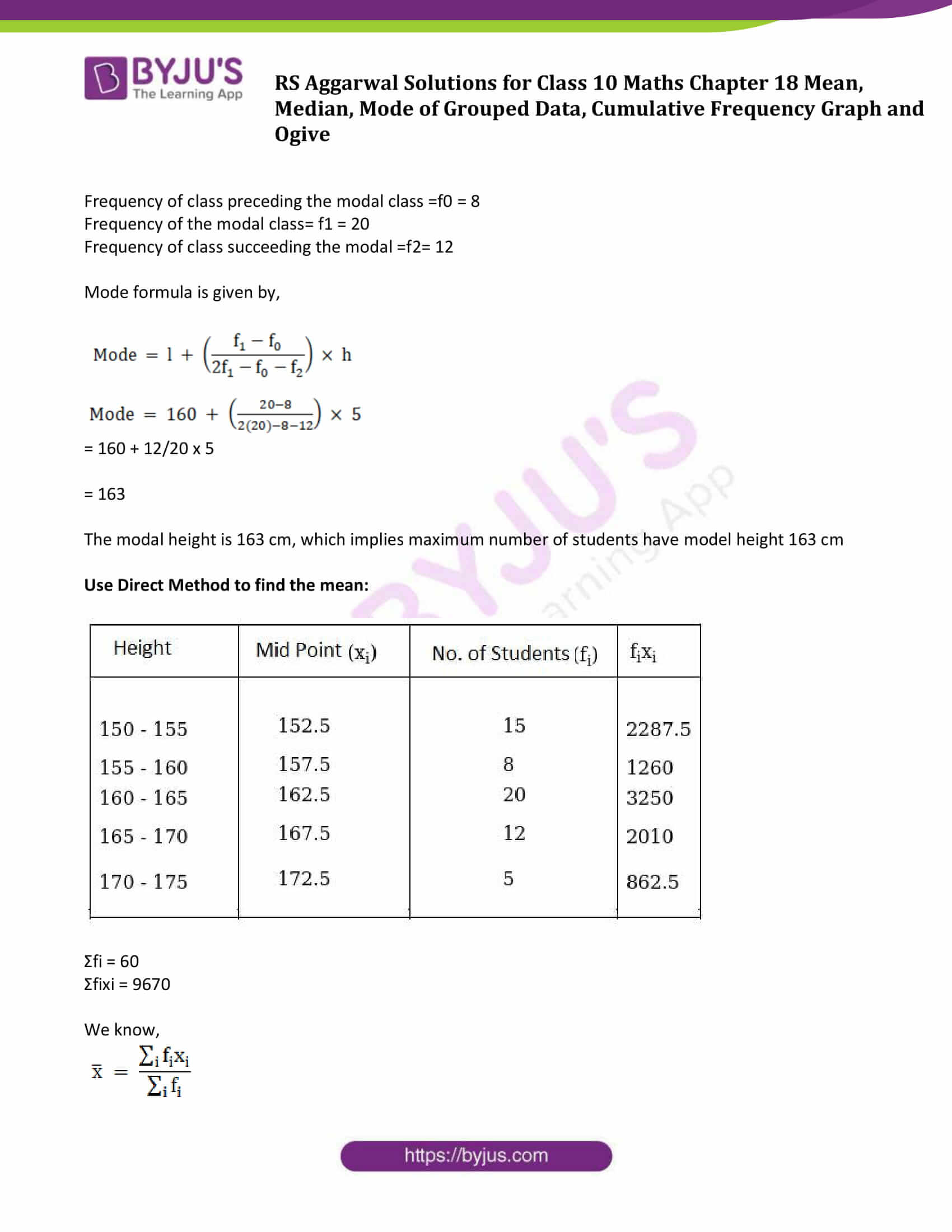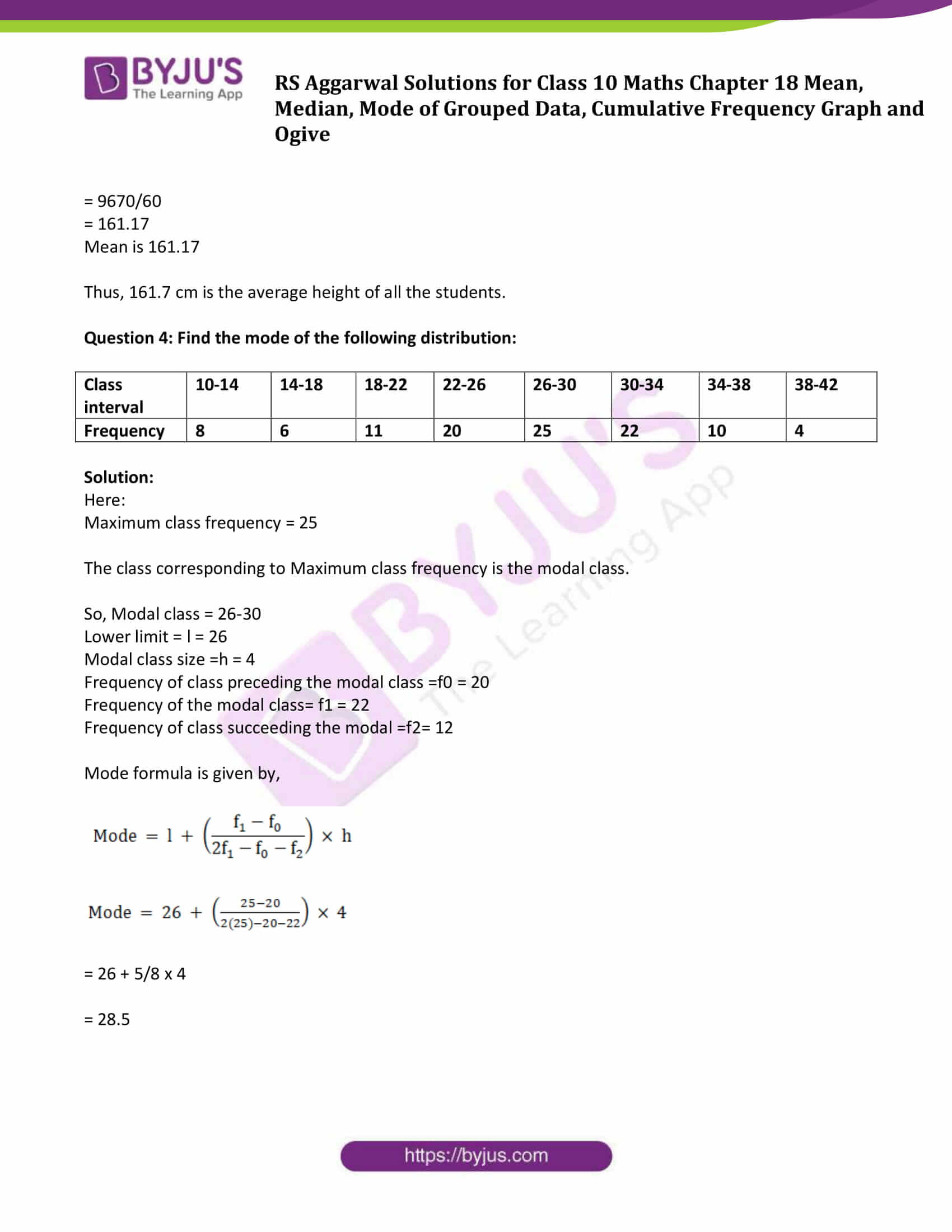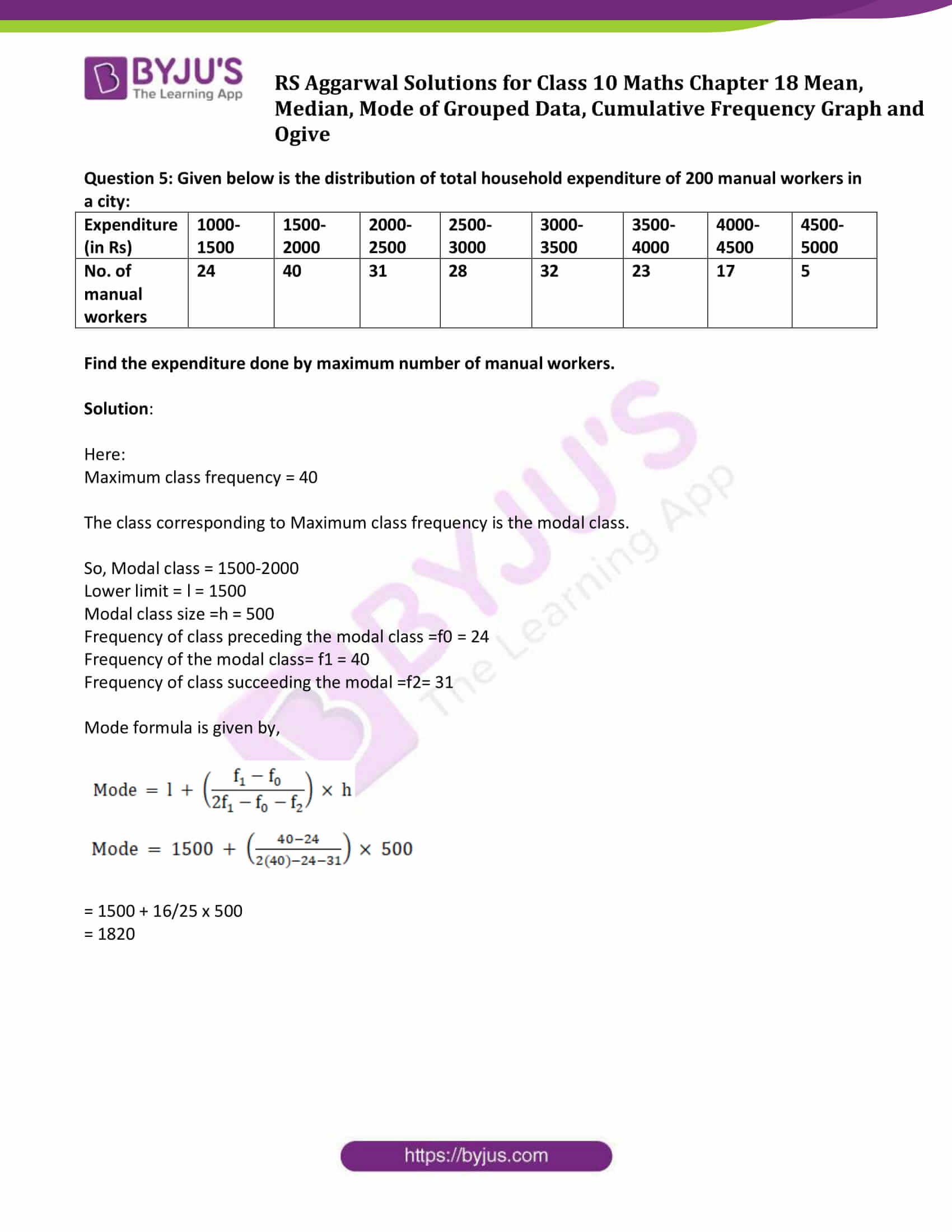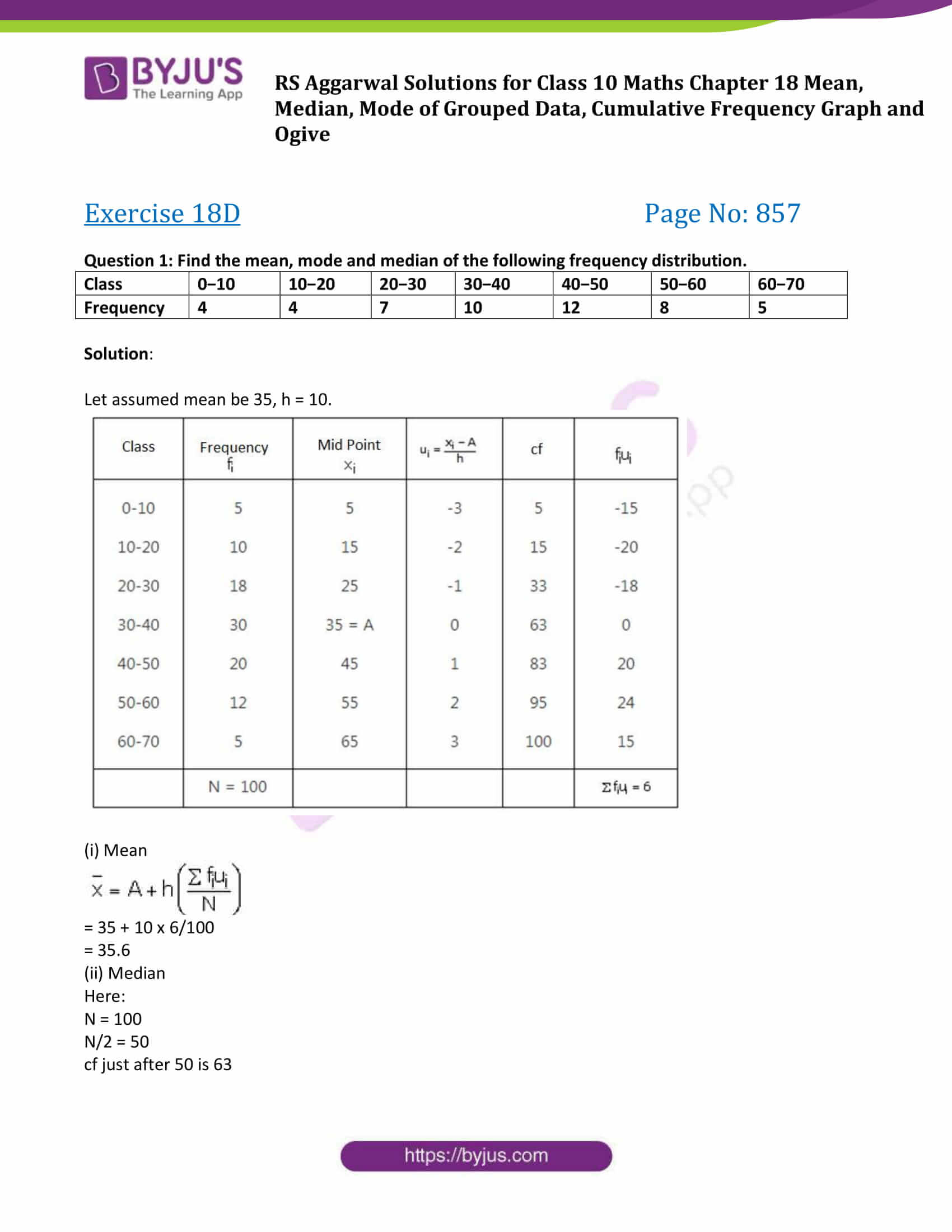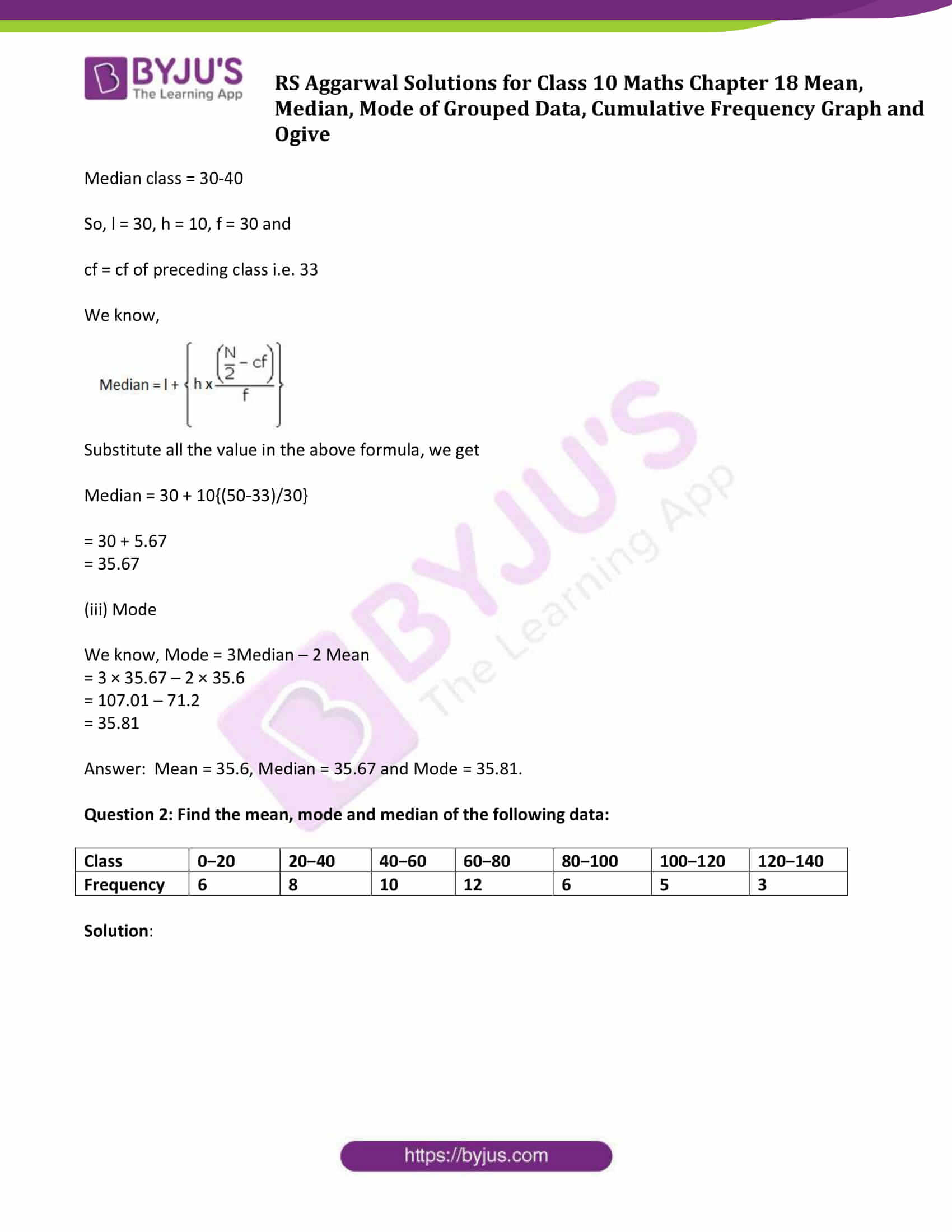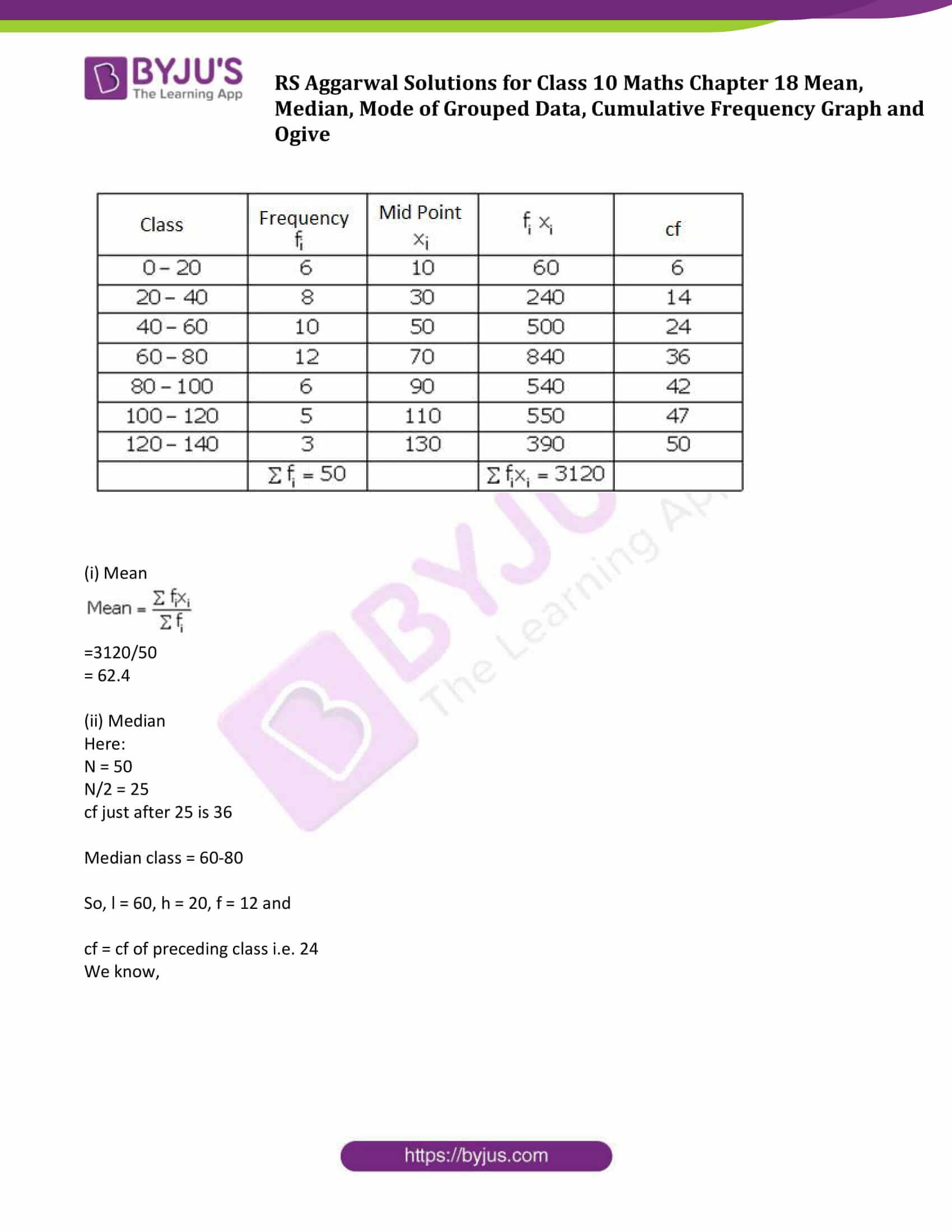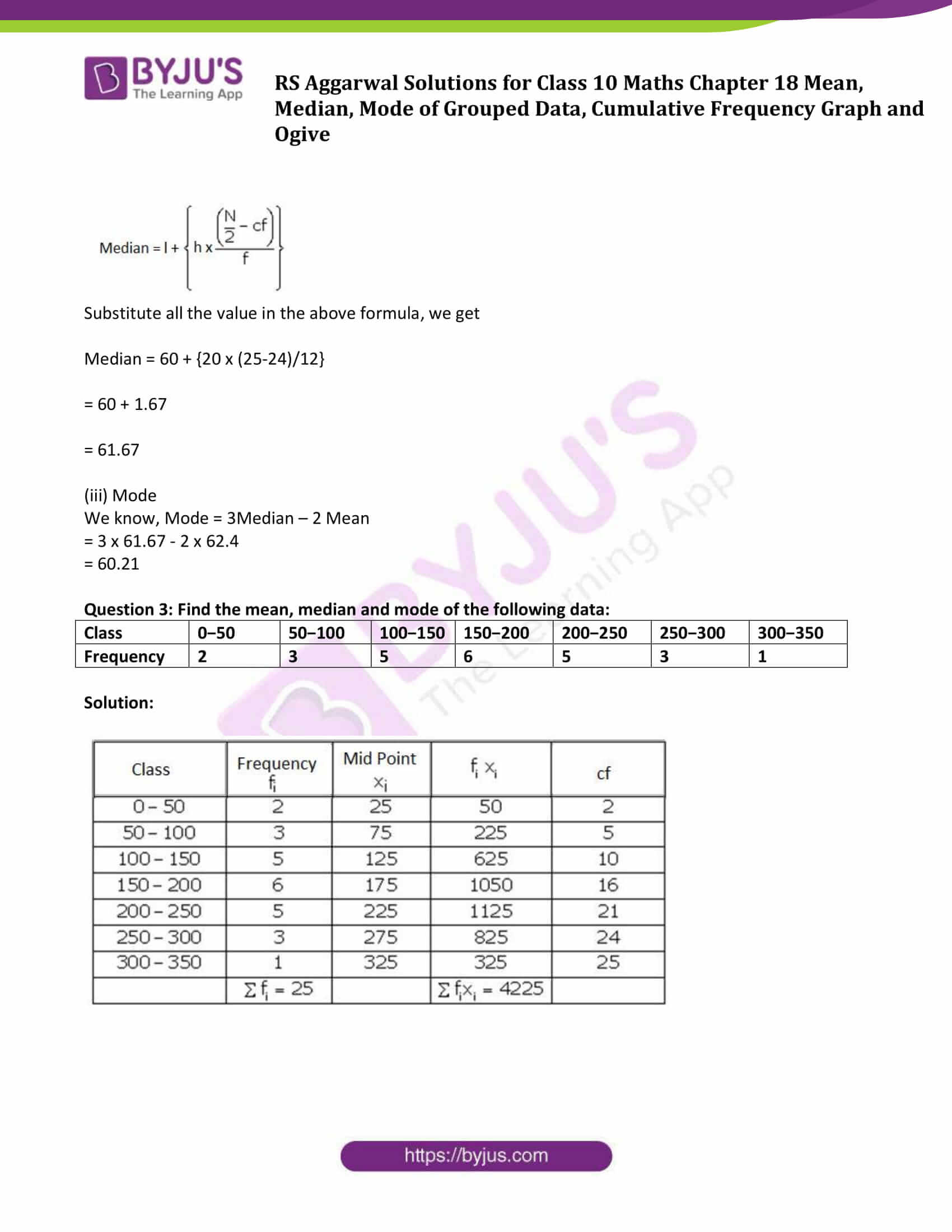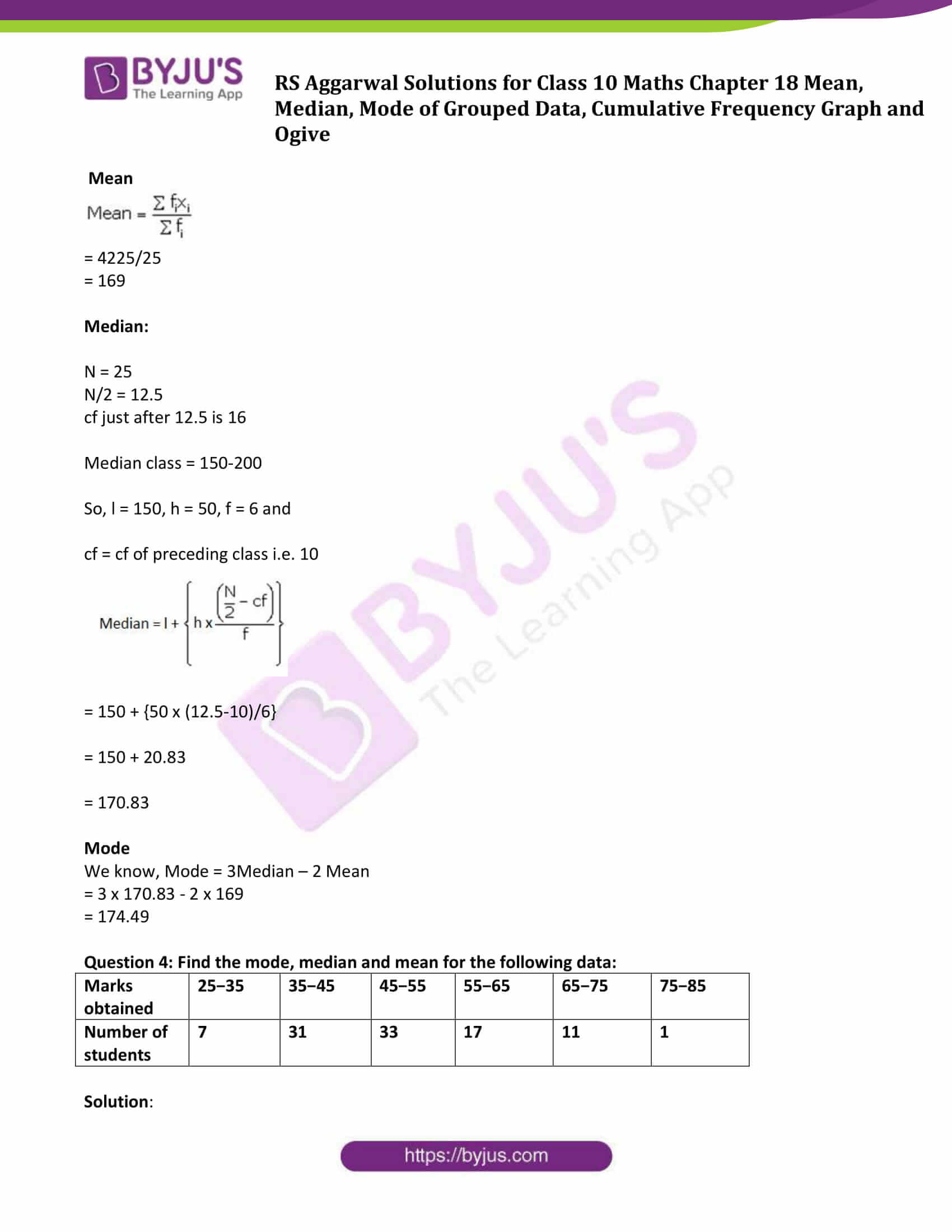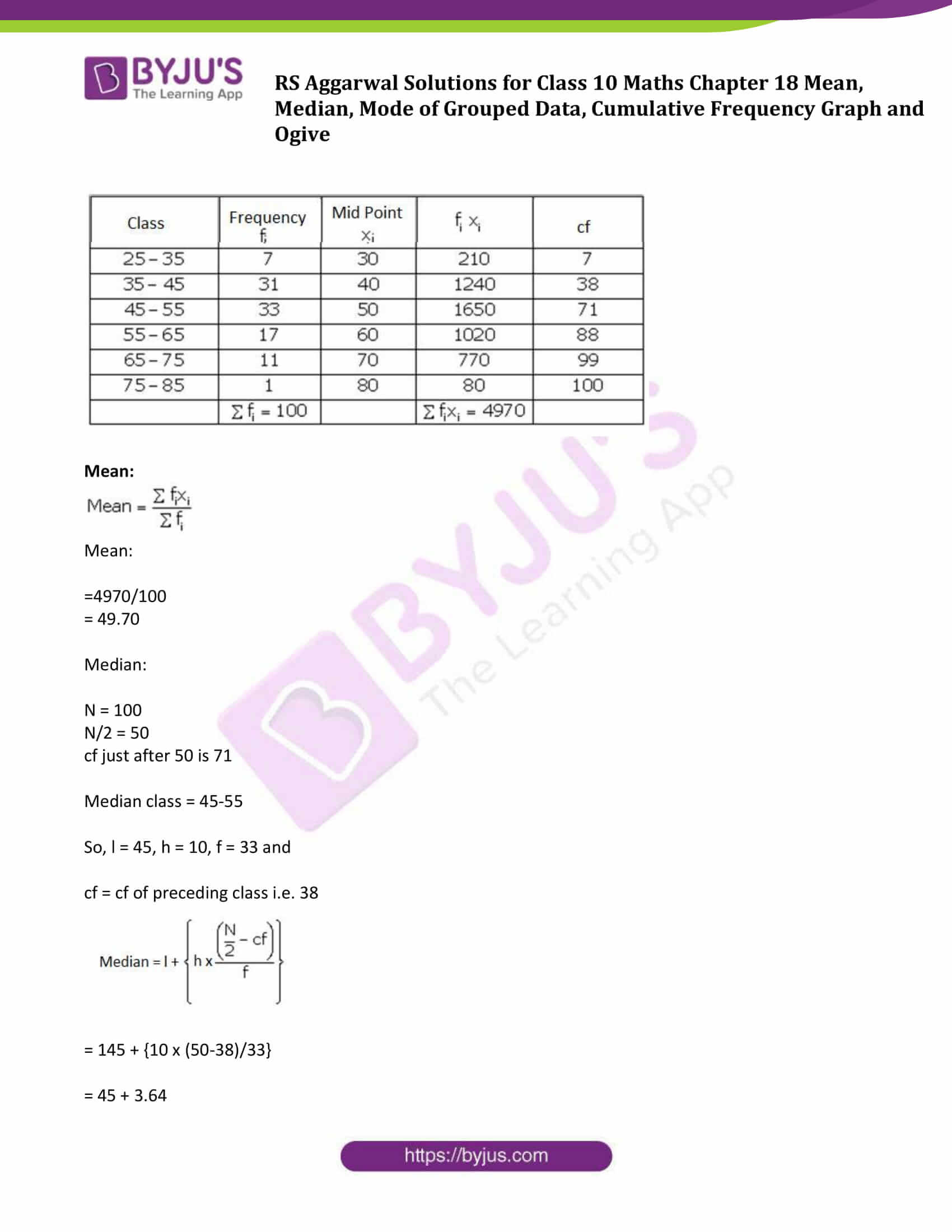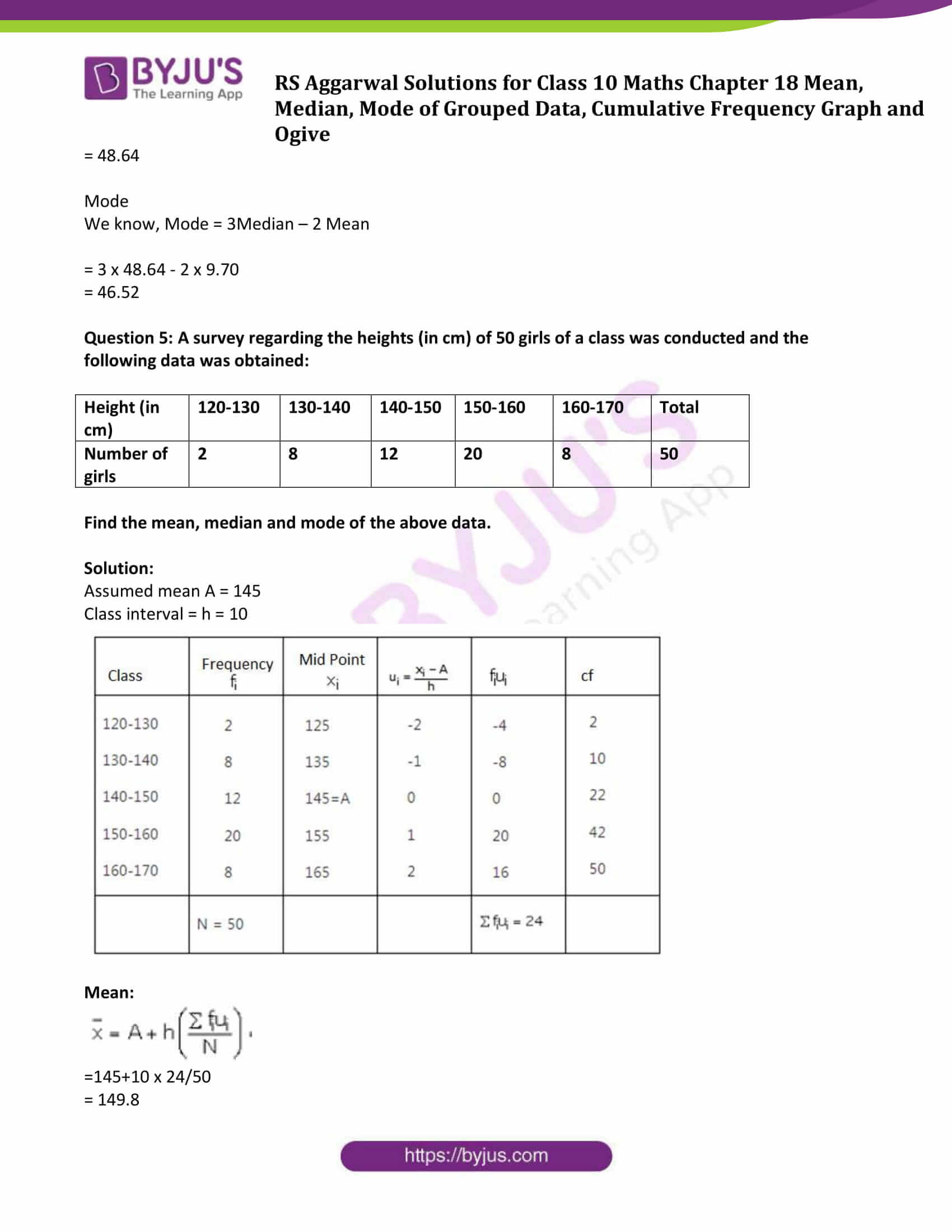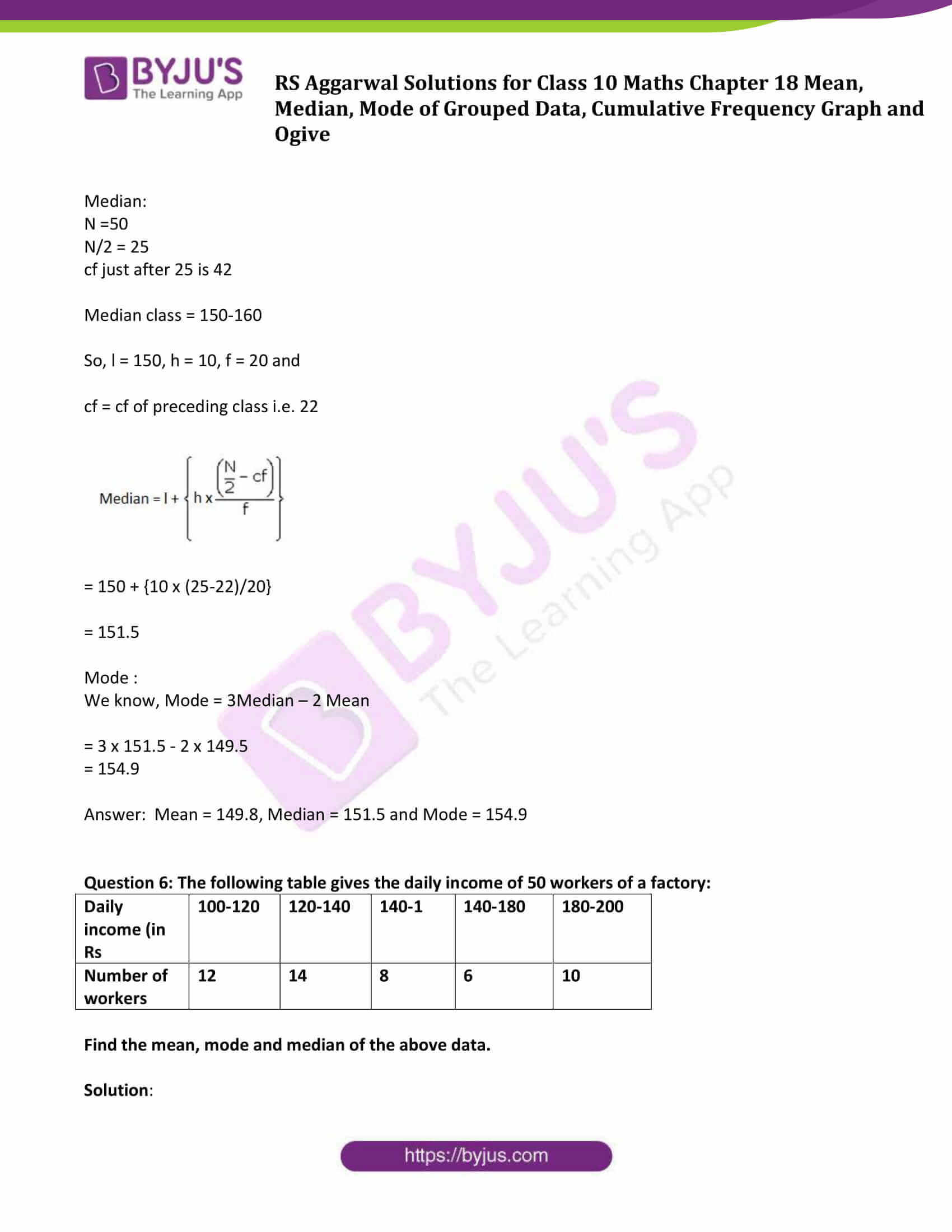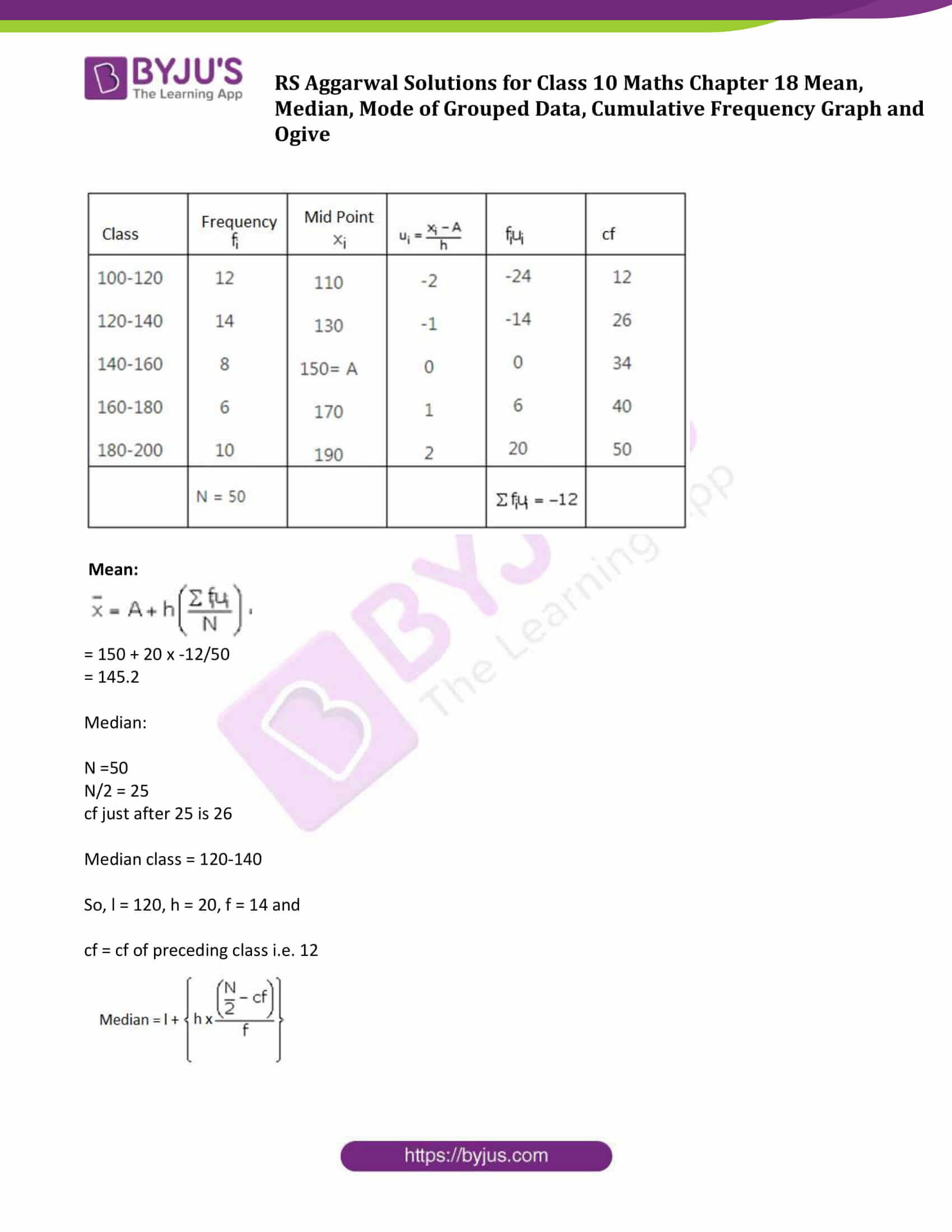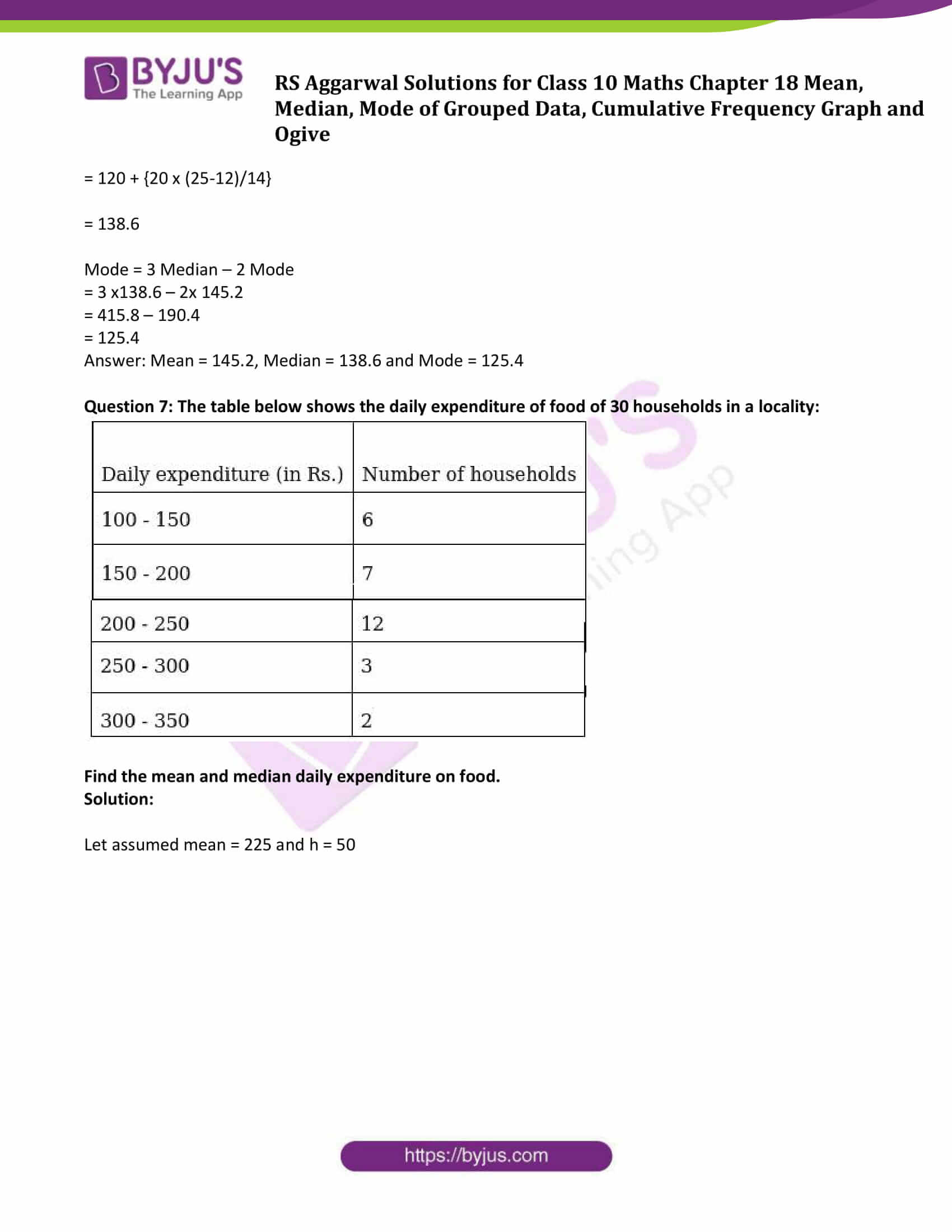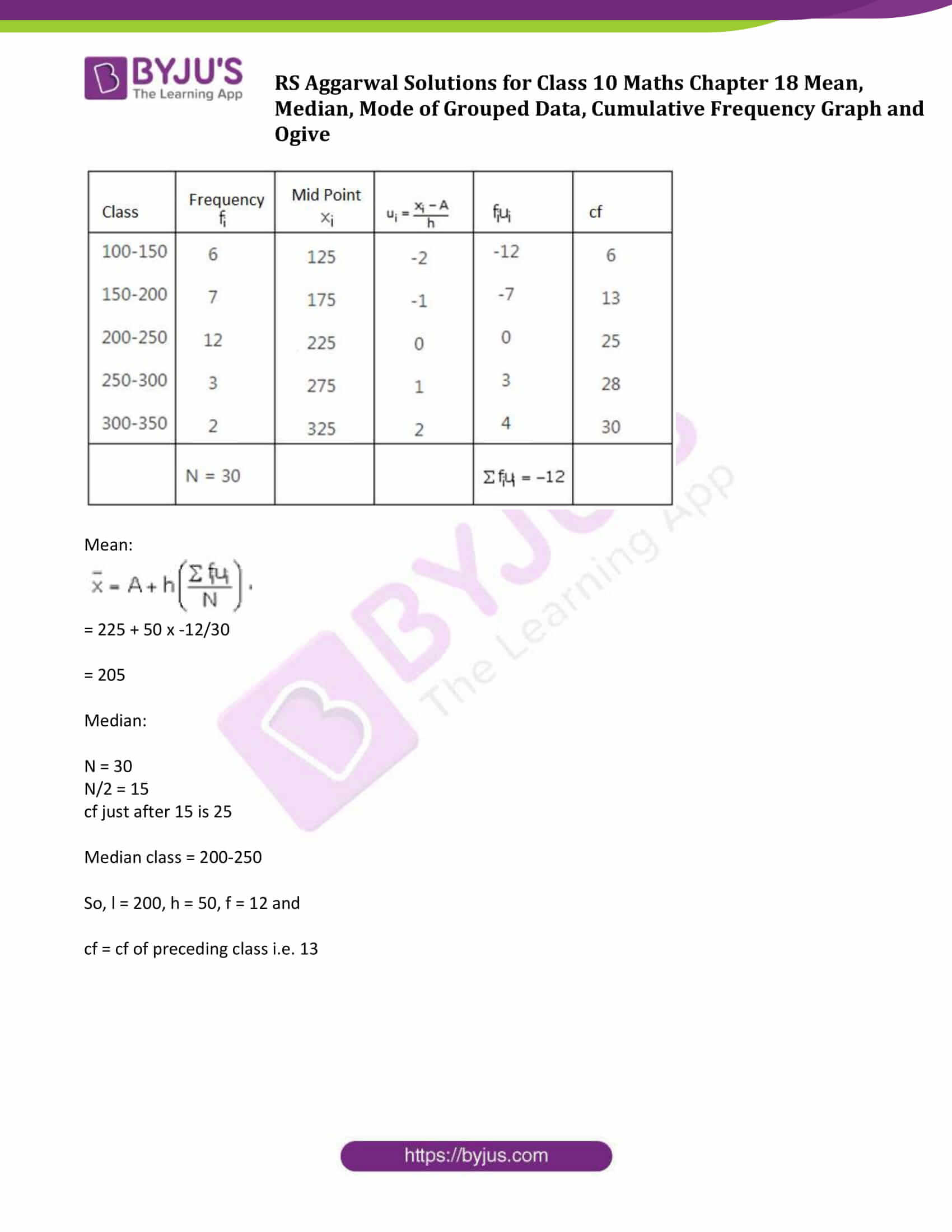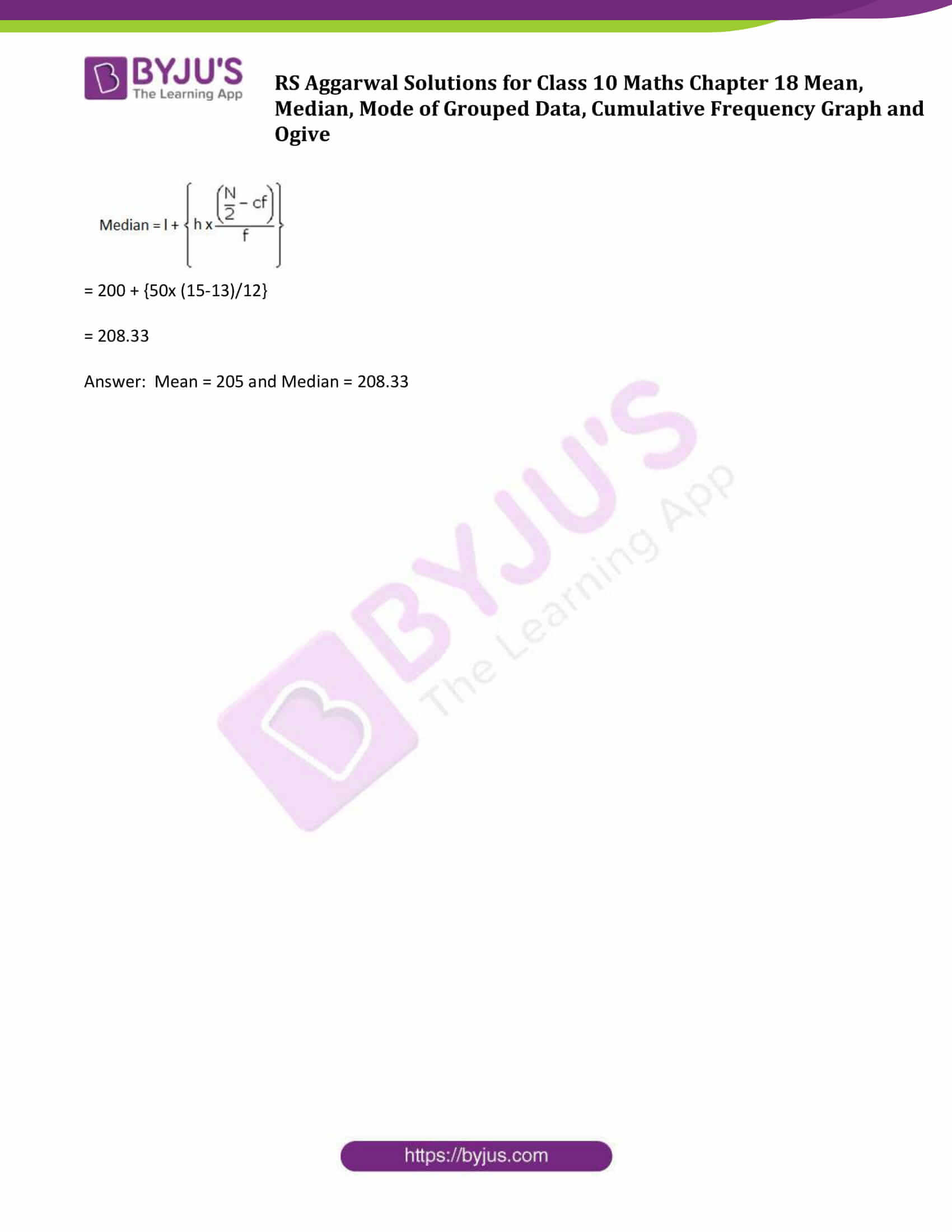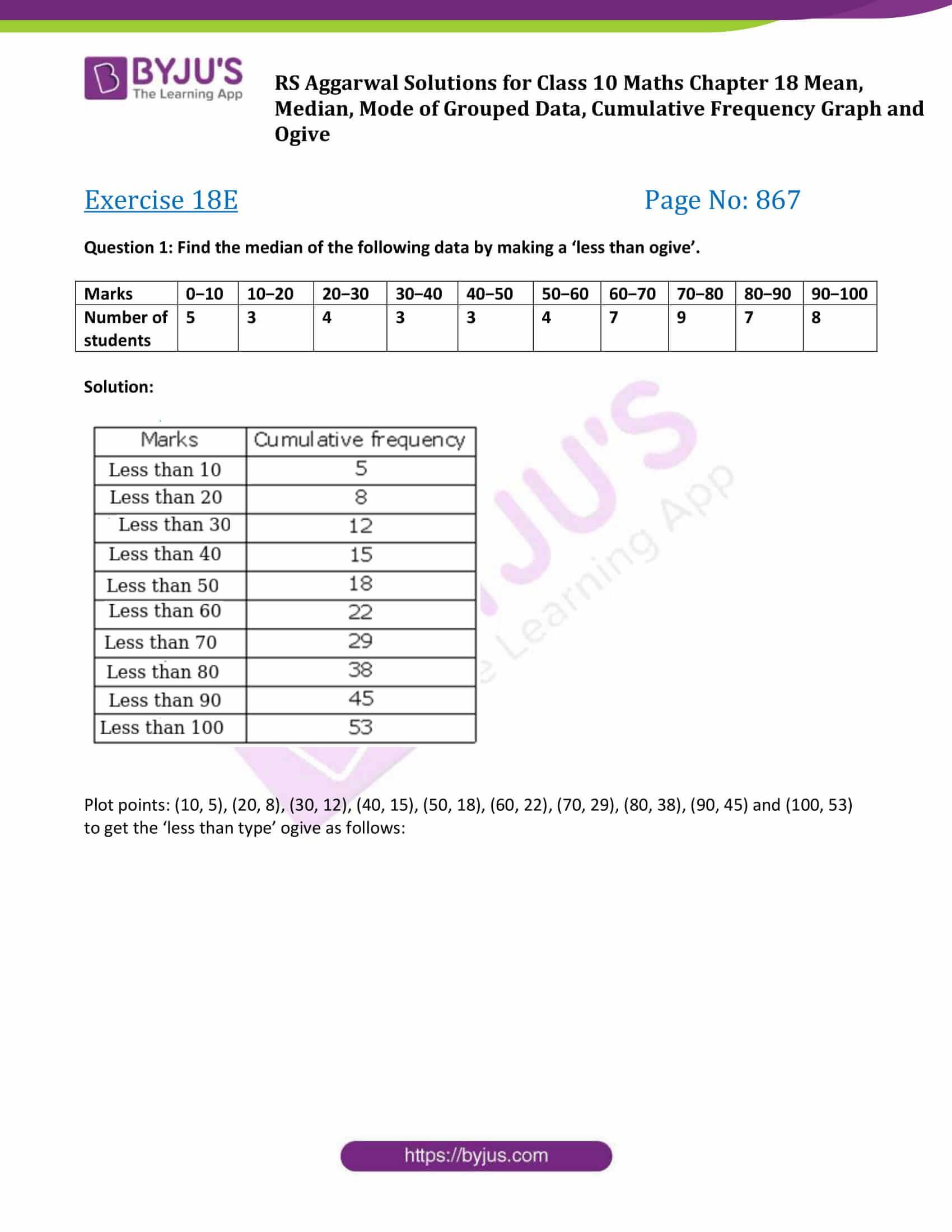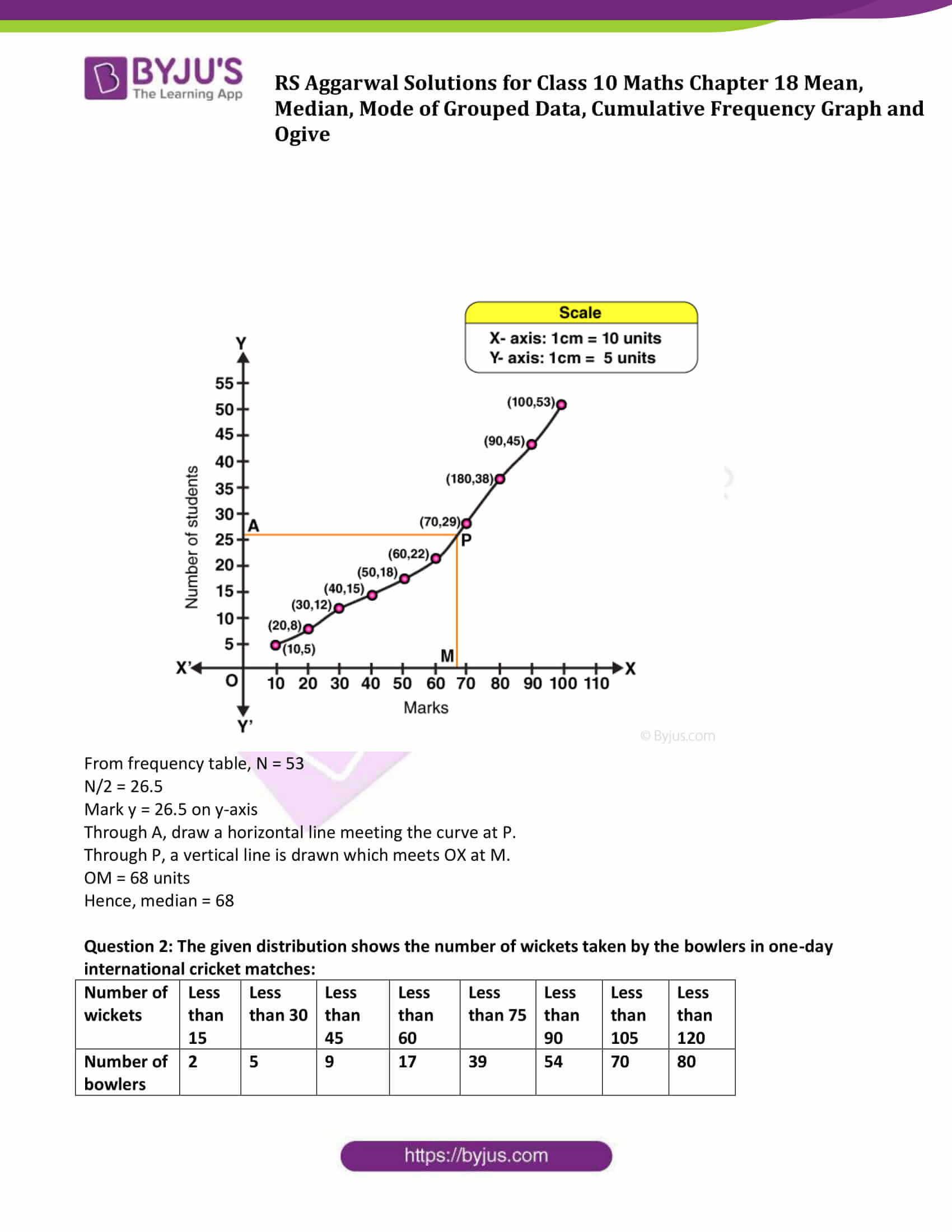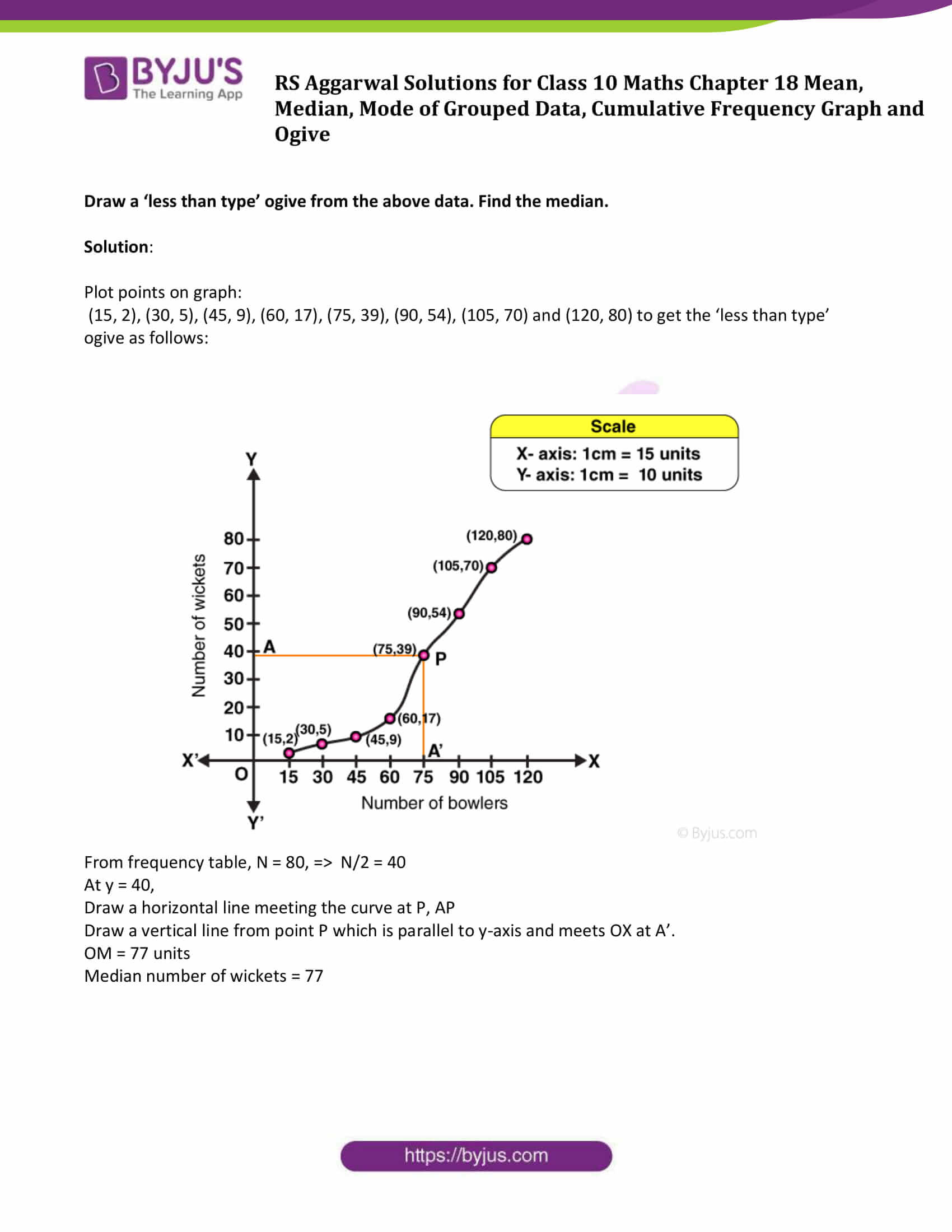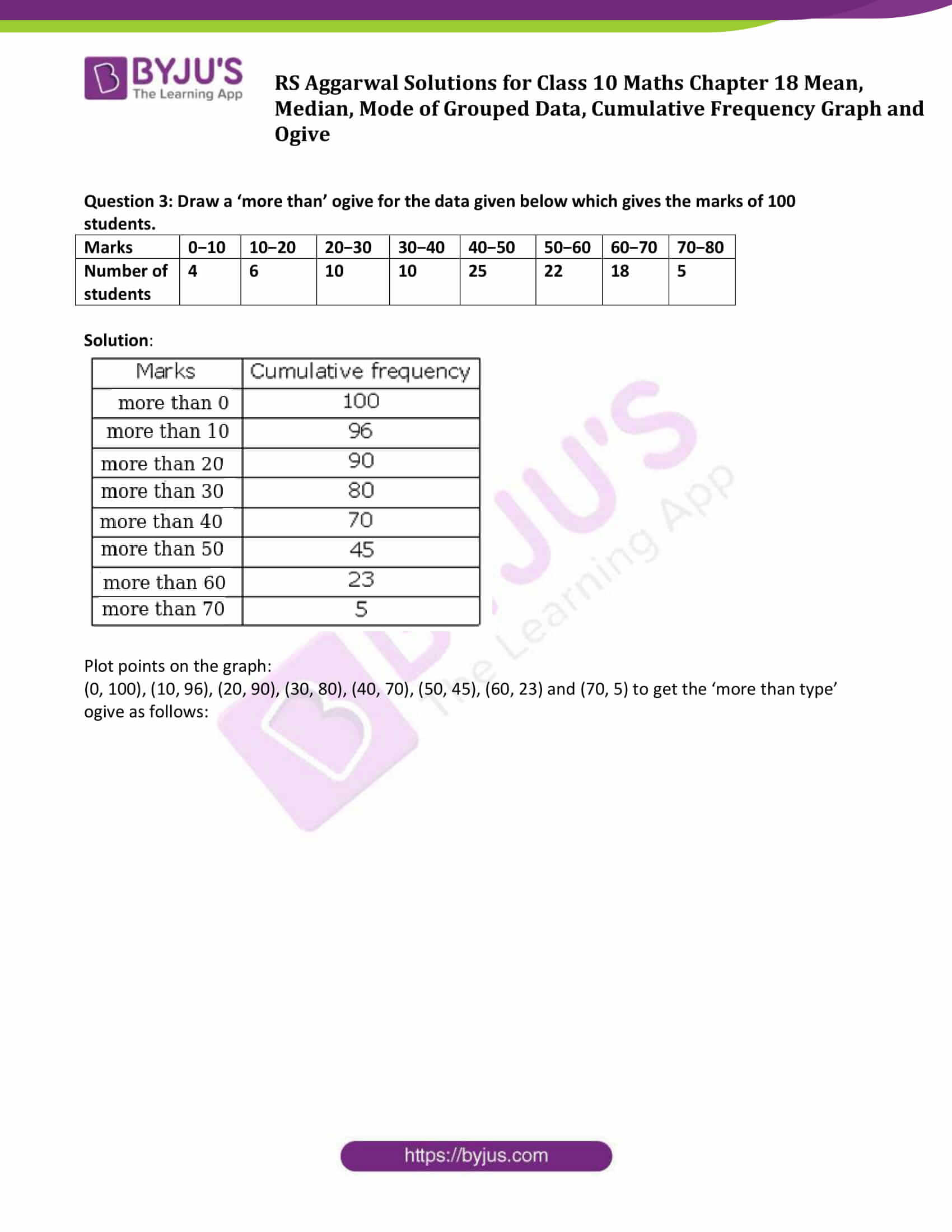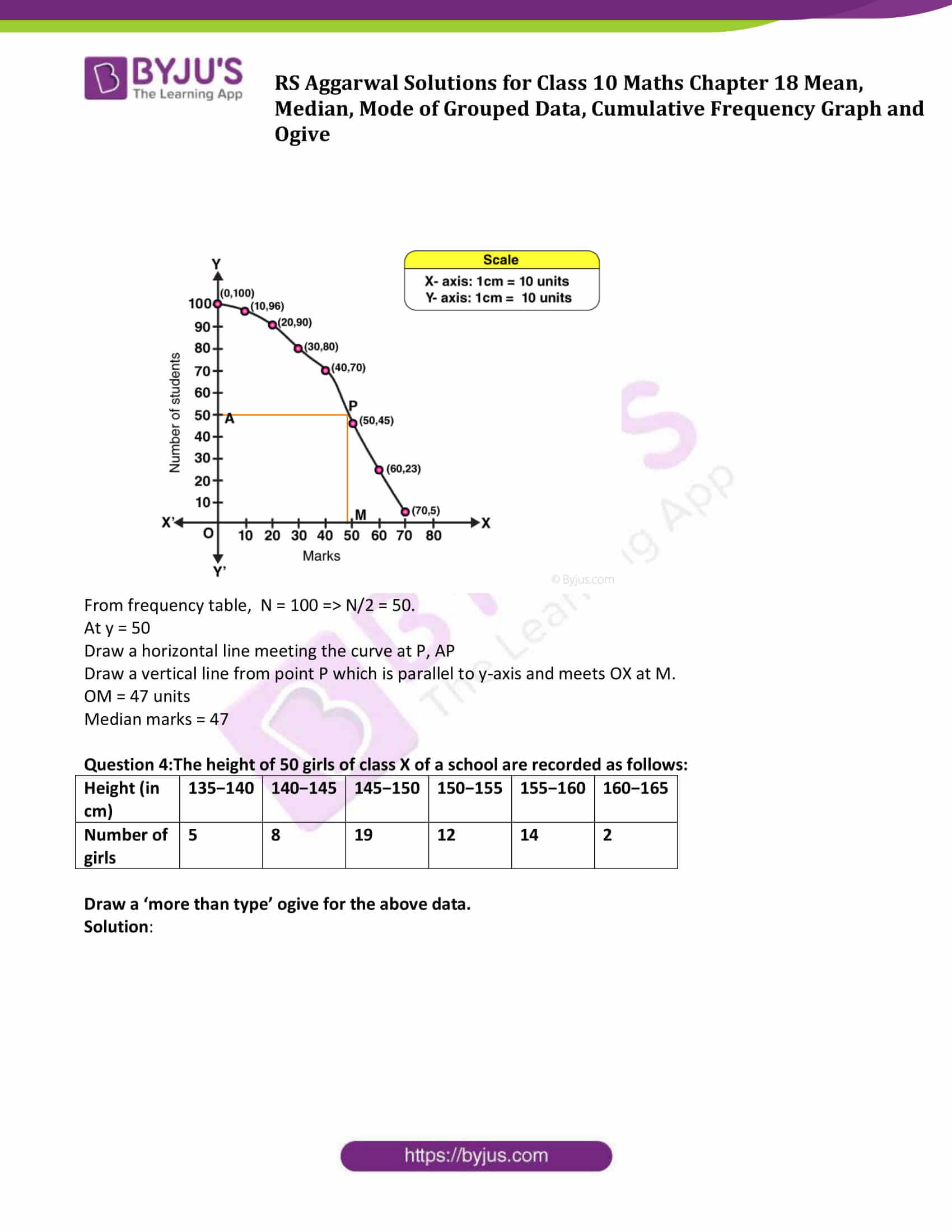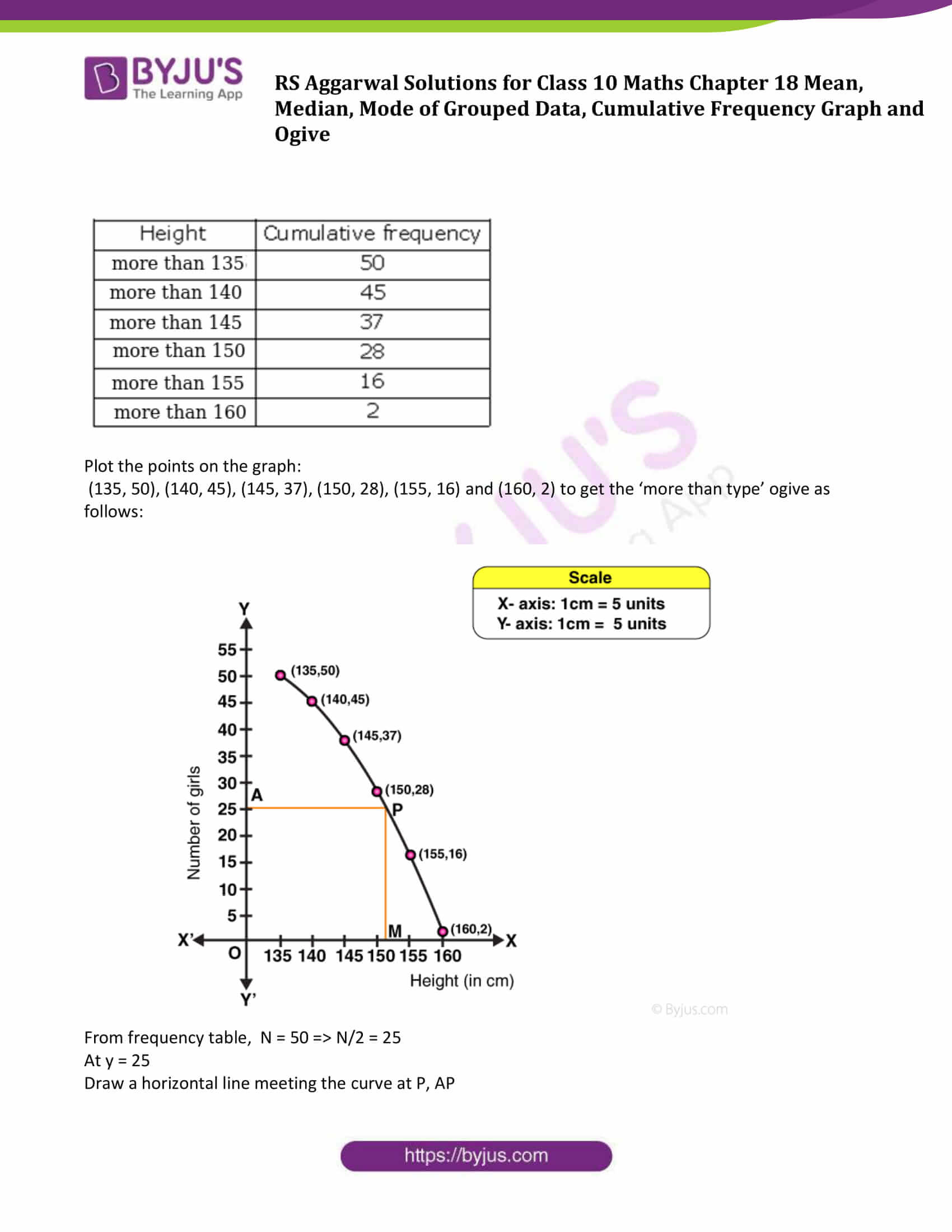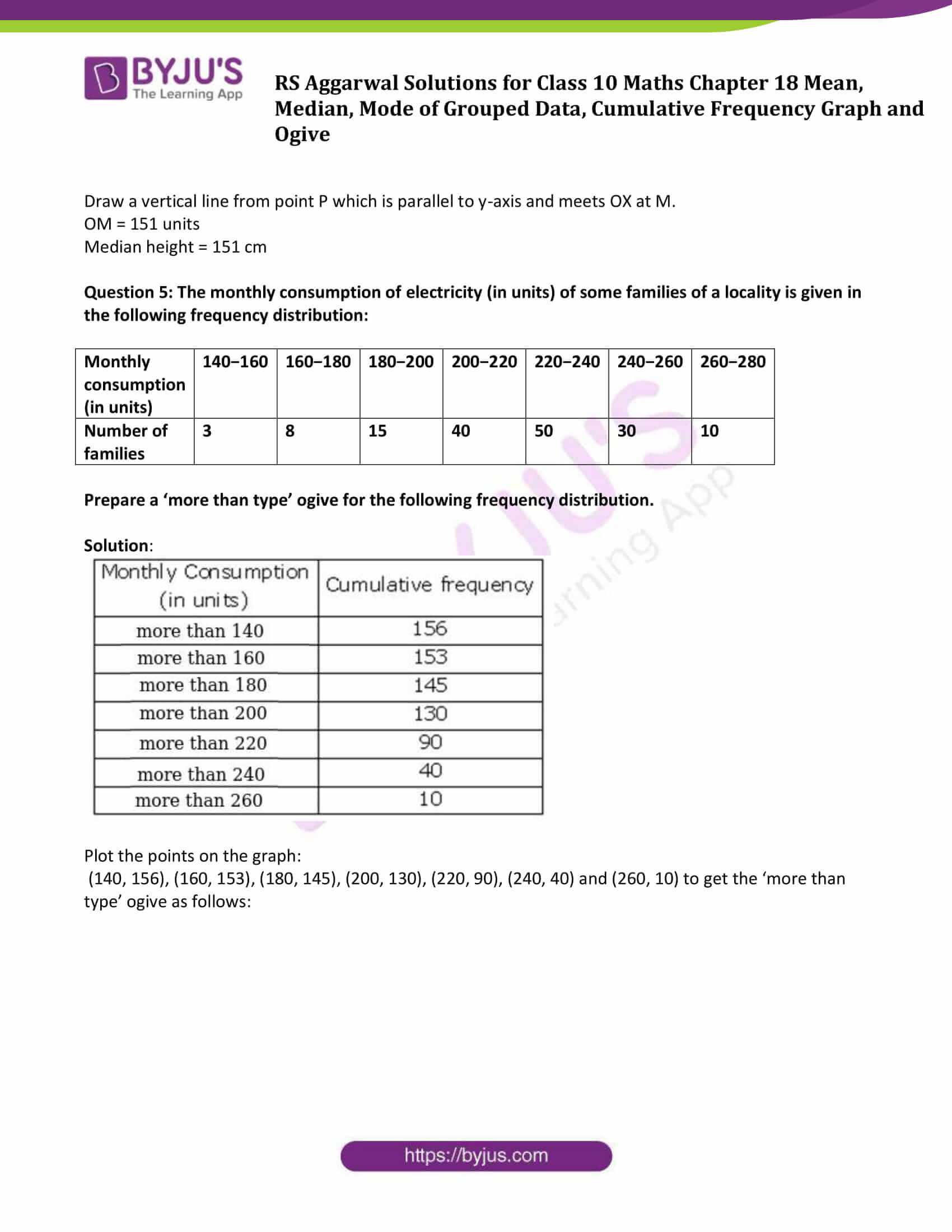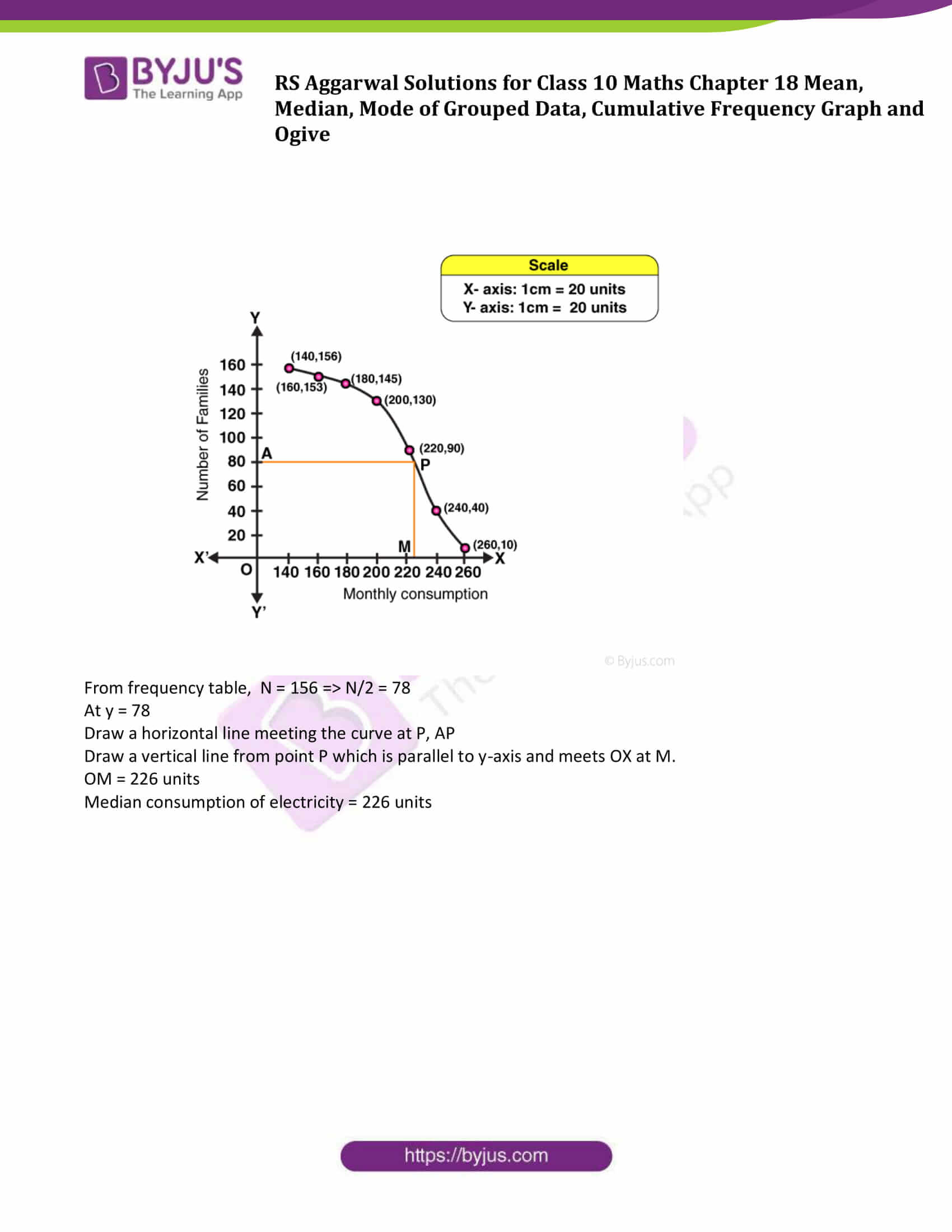## Exercise 18A

Question 1: If the mean of 5 observations x, x + 2, x + 4, x + 6 and x + 8 is 11, find the value of x.

Solution:

We know that,

$Mean = \frac{Sum\,of\, the\, given\, observations}{Total\, number\, of\, observations}$

Sum of the given observations = x + (x + 2) + (x + 4) + (x + 6) + (x + 8)

= x + x + 2 + x + 4 + x + 6 + x + 8

= 5x + 20

Total number of observations = 5

Therefore, Mean = (5x + 20)/5

Also, Mean = 11 (given)

⇨ 11 = (5x + 20)/5

⇨ 55 = 5x + 20

or x = 7

Question 2: If the mean of 25 observations is 27 and each observation is decreased by 7, what will be the new mean?

Solution:

Mean of 25 observations = 27 (given)

Total observations = 25

$Mean = \frac{Sum\,of\, the\, given\, observations}{Total\, number\, of\, observations}$

27 = (sum of 25 observations)/25

⇨ sum of 25 observations = 27 x 25 = 675

When each observation is decreased by 7, then

New Sum is 675 – 25 x 7 = 500

New Mean = 500/25= 20

Question 3: Compute the mean of following data:

 Class 1-3 3-5 5-7 7-9 Frequency 12 22 27 19

Solution: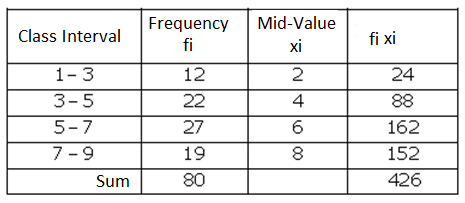Now,

$Mean = \frac{\sum fixi}{\sum fi}$

= 426/80

= 5.325

Question 4: Find the mean of the following data, using direct method:

 Class 0-10 10-20 20-30 30-40 40-50 50-60 Frequency 7 5 6 12 8 2

Solution: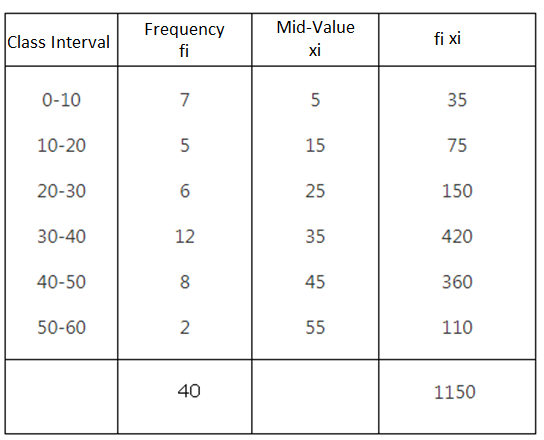$Mean = \frac{\sum fixi}{\sum fi}$

= 1150/40

= 28.75

Question 5: Find the mean of the following data, using direct method:

 Class 25-35 35-45 45-55 55-65 65-75 Frequency 6 10 8 12 4

Solution: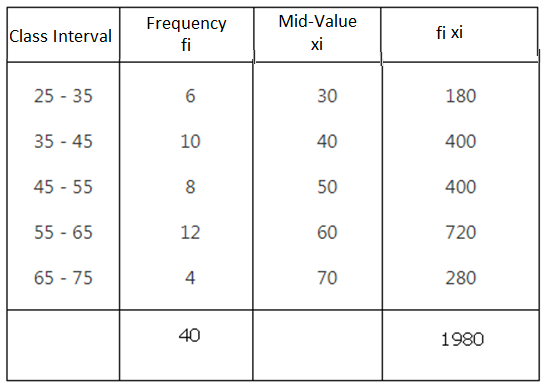$Mean = \frac{\sum fixi}{\sum fi}$

= 1980/40

=49.5

Question 6: Compute the mean of the following data, using direct method:

 Class 0-100 100-200 200-300 300-400 400-500 Frequency 6 9 15 12 8

Solution: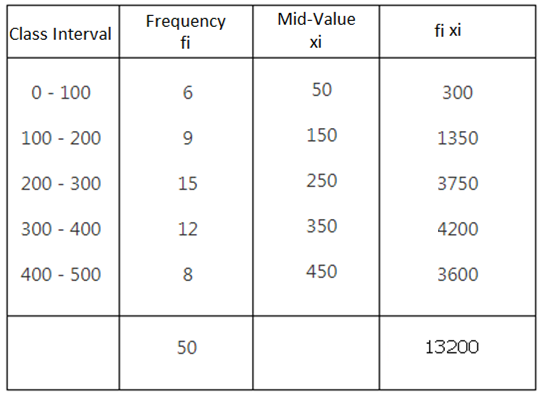$Mean = \frac{\sum fixi}{\sum fi}$

= 13200/40

= 264

Question 7: Using an appropriate method, find the mean of following frequency distribution:

 Class 84−90 90−96 96−102 102−108 108−114 114−120 Frequency 8 10 16 23 12 11

Which method did you use, and why?

Solution: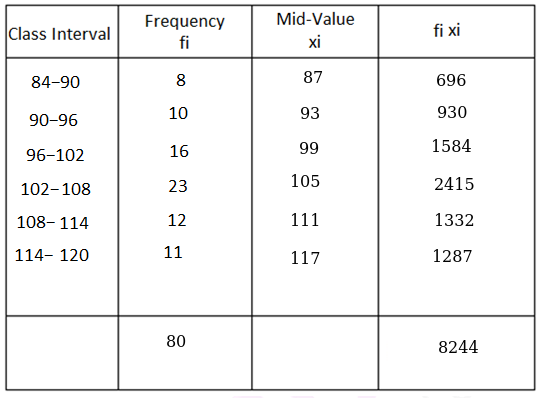$Mean = \frac{\sum fixi}{\sum fi}$

= 8244/80

= 103.05

Used direct method as it is easy to calculate.

Question 8: If the mean of the following frequency distribution is 24, find the value of p.

 Class 0−10 10−20 20−30 30−40 40−50 Frequency 3 4 p 3 2

Solution: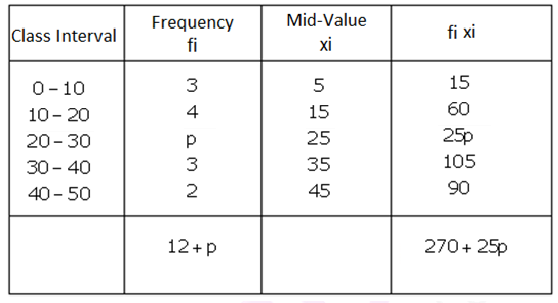$Mean = \frac{\sum fixi}{\sum fi}$

Mean = 24 ( given)

⇨ 24 = (270+25p)/(12+p)

⇨ 24(12+p) = 270 + 25p

or p = 18

Question 9: The following distribution shows the daily pocket allowance of children of a locality. If the mean pocket allowance is Rs. 18, find the missing frequency f.

 Daily pocket allowance (in Rs.) 11−13 13−15 15−17 17−19 19−21 21−23 23−25 Number of children 7 6 9 13 f 5 4

Solution: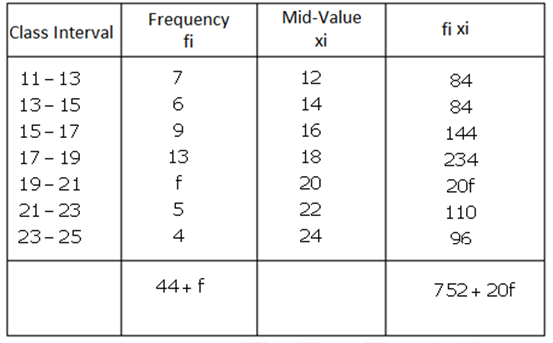$Mean = \frac{\sum fixi}{\sum fi}$

Mean = 18 (given)

18 = (752 + 20f)/(44+f)

792 + 18f = 752 + 20f

or f = 20

Question 10: If the mean of the following frequency distribution is 54, find the value of p.

 Class 0−20 20−40 40−60 60−80 80−100 Frequency 7 p 10 9 13

Solution: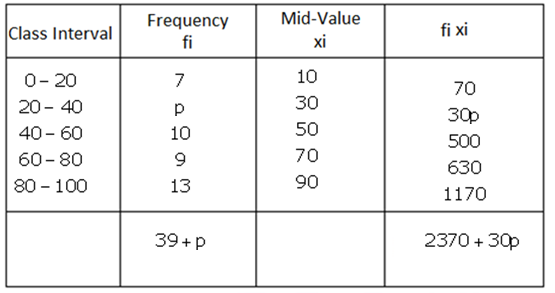$Mean = \frac{\sum fixi}{\sum fi}$

Mean = 54 (given)

⇨ 54 = (2370 + 30p)/(39+p)

⇨ 1053 + 27p = 1185 + 15p

or p = 11

## Exercise 18B

Question 1: In a hospital, the ages of diabetic patients were recorded as follows. Find the median age.

 Age (in years) 0−15 15−30 30−45 45−60 60−75 Number of patients 5 20 40 50 25

Solution: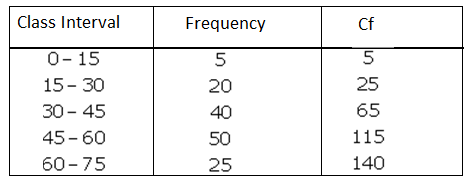Where cf = cumulative frequency

$Median = I +\left \{ h\times\frac{\left ( \frac{N}{2} – cf\right )}{f} \right \}$

Here:

N = 140

N/2 = 70

cf > 70 is 140

Median class = 45-60

So, l = 45, h = 15, f = 50 and

cf = cf of preceding class i.e. 65

Substitute all the value in the above formula, we get

Median = 45 + {15 x (70-65)/50}

= 45 + 1.5

= 46.5

Therefore, median age of diabetic patients is 46.5 years.

Question 2: Compute the median from the following data:

 Marks 0-7 7-14 14-21 21-28 28-35 35-42 42-49 Number of students 3 4 7 11 0 16 9

Solution: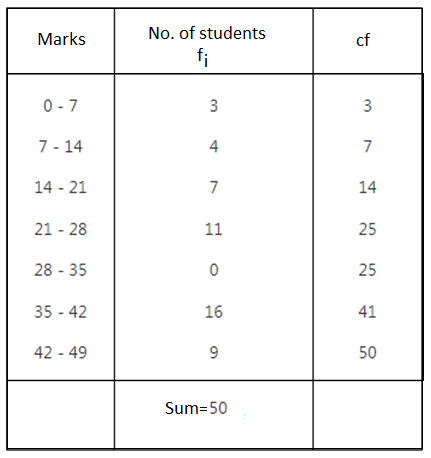Where cf = cumulative frequency

$Median = I +\left \{ h\times\frac{\left ( \frac{N}{2} – cf\right )}{f} \right \}$

Here:

N = 50

N/2 = 25

Here cumulative frequency is 25

Median class = 21-28

So, l = 21, h =7, f = 11 and

cf = cf of preceding class i.e. 14

Substitute all the value in the above formula, we get

Median =21 + {7x (25-14)/11}

= 28

Question 3: The following table shows the daily wages of workers in a factory:

 Daily wages (in Rs) 0-100 100-200 200-300 300-400 400-500 Number of workers 40 32 48 22 8

Find the median daily wage income of the workers.

Solution: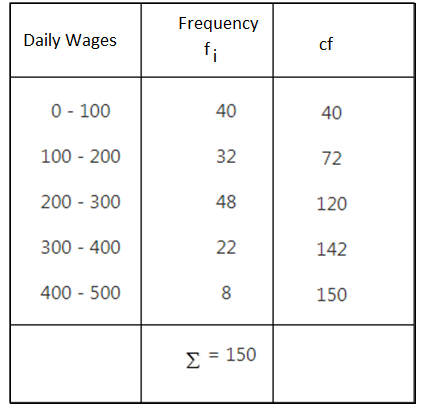Where cf = cumulative frequency

$Median = I +\left \{ h\times\frac{\left ( \frac{N}{2} – cf\right )}{f} \right \}$

Here:

N = 150

N/2 = 75

cf just greater than 75 is 120

Median class = 200-300

So, l = 200, h =100, f = 48 and

cf = cf of preceding class i.e. 72

Substitute all the value in the above formula, we get

Median =200+ {100 x (75-72)/48}

= 200 + 6.25

= 206.25

Median of daily wages is Rs. 206.25.

Question 4: Calculate the median from the following frequency distribution: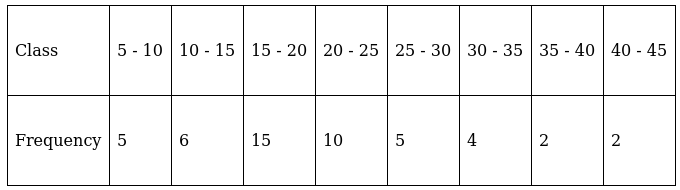Solution: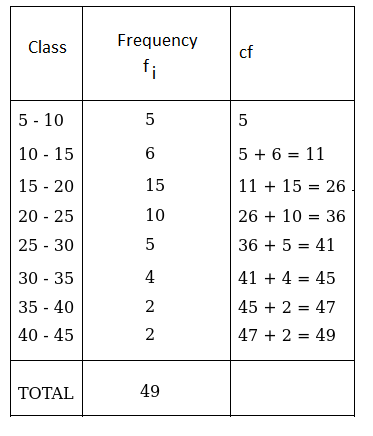Where cf = cumulative frequency

$Median = I +\left \{ h\times\frac{\left ( \frac{N}{2} – cf\right )}{f} \right \}$

Here:

N = 49

N/2 = 24.5

cf just greater than 24.5 is 26

Median class = 15-20

So, l = 15, h = 5, f = 15 and

cf = cf of preceding class i.e. 11

Substitute all the value in the above formula, we get

Median = 15+ {5 x (24.5-11)/15}

= 15 + 4.5

= 19.5

Thus, median of frequency distribution is 19.5.

Question 5: Given below is the number of units of electricity consumed in a week in a certain locality: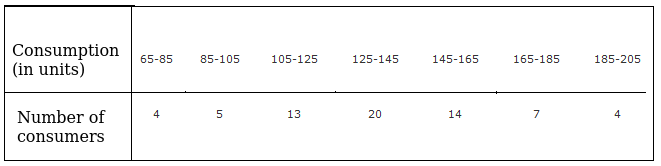Calculate the median.

Solution: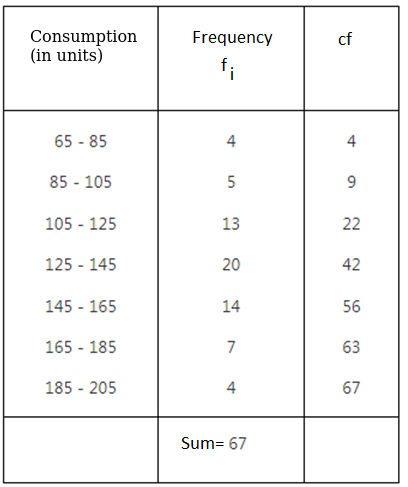Where cf = cumulative frequency

$Median = I +\left \{ h\times\frac{\left ( \frac{N}{2} – cf\right )}{f} \right \}$

Here:

N = 67

N/2 = 33.5

cf just greater than 33.5 is 42

Median class = 125-145

So, l = 125, h = 20, f = 20 and

cf = cf of preceding class i.e. 22

Substitute all the value in the above formula, we get

Median = 125+ {20 x (33.5-22)/20}

= 125 + 11.5

= 136.5

Thus, median of electricity consumed is 136.5.

Question 6: Calculate the median from the following data: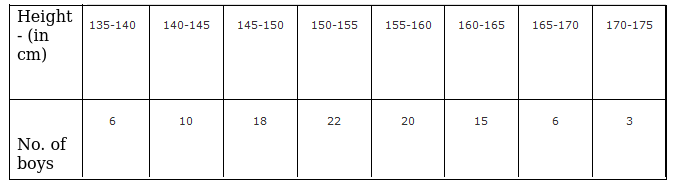Solution: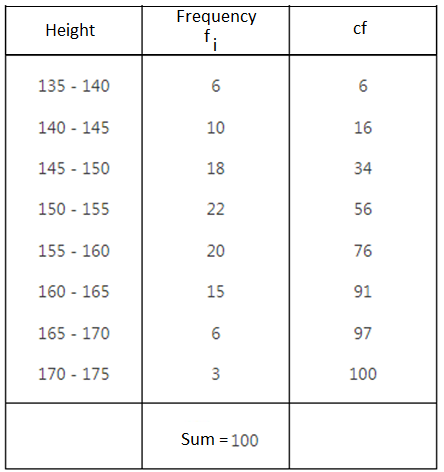Where cf = cumulative frequency

$Median = I +\left \{ h\times\frac{\left ( \frac{N}{2} – cf\right )}{f} \right \}$

Here:

N = 100

N/2 = 50

cf just greater than 50 is 56

Median class = 150-155

So, l = 150, h = 5, f = 22 and

cf = cf of preceding class i.e. 34

Substitute all the value in the above formula, we get

Median = 150+ {5 x (50-34)/22}

= 150 + 3.64

= 153.64

Question 7: Calculate the missing frequency from the following distribution, it being given that the median of the distribution is 24.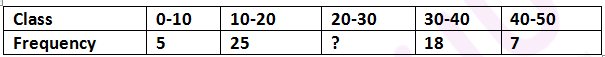Solution:

Let the missing frequency be x.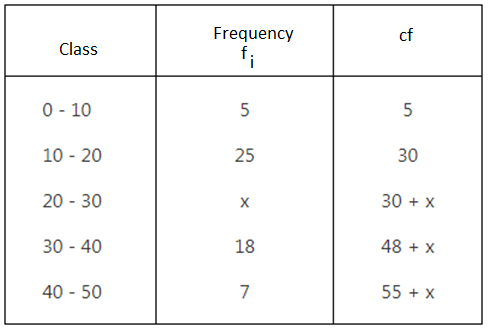Median = 24

N/2 = (55+x)/2 = 27.5 + x/2

Median class = 20-30

l = 20, h = 10, f = x and cf = cf of preceding class i.e. 30

Using below formula:

$Median = I +\left \{ h\times\frac{\left ( \frac{N}{2} – cf\right )}{f} \right \}$

⇨ 24 = 20 + {10 x (27.5+ x/2 – 30)/x}

⇨ 24x = 20x + 5x – 25

or x = 25

Missing frequency is 25.

Question 8: The median of the following data is 16. Find the missing frequencies a and b if the total of frequencies is 70.

 Class 0−5 5−10 10−15 15−20 20−25 25−30 30−35 35−40 Frequency 12 a 12 15 b 6 6 4

Solution: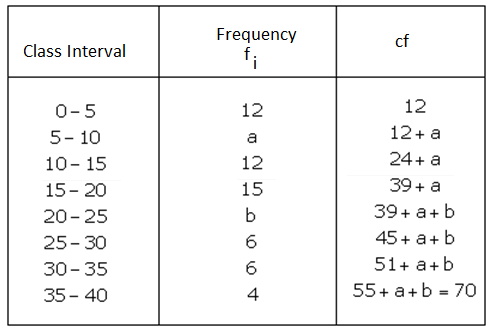Here:

N = 70

N/2 = 35

Median = 16, Median class = 15-20

So, l = 15, h = 5, f = 15 and

cf = cf of preceding class i.e. 24+a

We know,

$Median = I +\left \{ h\times\frac{\left ( \frac{N}{2} – cf\right )}{f} \right \}$

Substitute all the value in the above formula, we get

16 = 15 + {5x (35-24-a)/15}

⇨ 16 = 15 + (11-a)/3

or a = 8

Now, 70 = 55 + a + b

70 = 55 + 8 + b

⇨ b = 7

So, missing freqiuncies are 8 and 7.

Question 9: In the following data the median of the runs scored by 60 top batsmen of the world in one-day international cricket matches is 5000. Find the missing frequencies x and y.

 Runs scored 2500−3500 3500−4500 4500−5500 5500−6500 6500−7500 7500−8500 Number of batsmen 5 x y 12 6 2

Solution: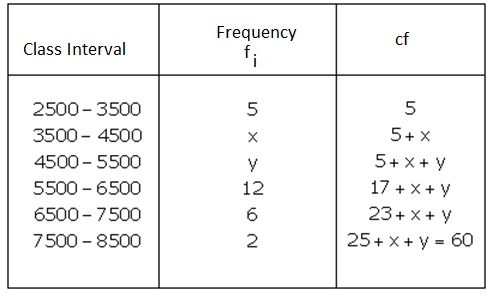Here:

N = 60

N/2 = 30

Median = 5000, Median class = 4500-5500

So, l = 4500, h = 1000, f = y and

cf = cf of preceding class i.e. 5+x

We know,

$Median = I +\left \{ h\times\frac{\left ( \frac{N}{2} – cf\right )}{f} \right \}$

Substitute all the value in the above formula, we get

5000 = 4500 + {1000 x (30-5-x)/y}

⇨ 500 = 1000 x (25-x)/y

⇨ 0.5 = (25-x)/y

⇨ x + 05.y = 25 …(1)

Again, from table

25 + x + y = 60

⇨ x + y = 35 …(2)

Solving (1) and (2), we have

x = 15 and y = 20

Missing frequencies are 15 and 20.

Question 10: If the median of the following frequency distribution is 32.5, find the values of f1 and f2.

 Class interval 0-10 10-20 20-30 30-40 40-50 50-60 60-70 Total Frequency f1 5 9 12 f2 3 2 40

Solution:

Sum of all the frequencies = f1+ 5 + 9 +12 + f2 + 3 + 2 = 40 (given)

⇨ f1+ f2 = 9 …(1)

Median is 32.5 lies in 30 – 40, so the median class is 30 – 40

N = 40

N/2 = 20

l = 30, h = 10, f = 12

cf = f1 + 5+9 = f1+14

We know,

$Median = I +\left \{ h\times\frac{\left ( \frac{N}{2} – cf\right )}{f} \right \}$

Substitute all the value in the above formula, we get

32.5 = 30 + {10 x (20-f1-14)/12 }

⇨ 32.5 = 30 + (30-5f1)/6

⇨ 2.5 = (30-5f1)/6

or f1 = 3

From (1)

⇨ 3 + f2 = 9

⇨ f2 = 6

## Exercise 18C

Question 1: Find the mode of the following frequency distribution:

 Marks 10−20 20−30 30−40 40−50 50−60 Frequency 12 35 45 25 13

Solution:

Here:

Maximum class frequency is 45.

The class corresponding to Maximum class frequency is the modal class.

So, Modal class = 30 – 40

Lower limit = l = 30

Modal class size =h = 10

Frequency of class preceding the modal class =f0 = 35

Frequency of the modal class= f1 = 45

Frequency of class succeeding the modal =f2= 25

Mode formula is given by,

$Mode = I +\left ( \frac{f_{1}-f_{0}}{2f_{1}-f_{0}-f_{2}} \right )\times h$ $Mode = 30 +\left ( \frac{45-35}{2\times 45-35-25} \right )\times 10$

= 30 + 100/30

= 33.33

Question 2: Compute the mode of the following data:

 Class 0−20 20−40 40−60 60−80 80−100 Frequency 25 16 28 20 5

Solution:

Here:

Maximum class frequency = 28

The class corresponding to Maximum class frequency is the modal class.

So, Modal class = 40-60

Lower limit = l = 40

Modal class size =h = 20

Frequency of class preceding the modal class =f0 = 16

Frequency of the modal class= f1 = 28

Frequency of class succeeding the modal =f2= 20

Mode formula is given by,

$Mode = I +\left ( \frac{f_{1}-f_{0}}{2f_{1}-f_{0}-f_{2}} \right )\times h$ $Mode = 40 +\left ( \frac{28-16}{2\times 28-16-20} \right )\times 20$

= 40 + 12/20 x 12

= 52

Question 3: Heights of students of Class X are given in the following frequency distribution:

 Height (in cm) 150−155 155−160 160−165 165−170 170−175 Number of students 15 8 20 5 13

Find the modal height. Also, find the mean height. Compare and interpret the two measures of central tendency.

Solution:

Here:

Maximum class frequency = 20

The class corresponding to Maximum class frequency is the modal class.

So, Modal class = 160-165

Lower limit = l = 160

Modal class size =h = 5

Frequency of class preceding the modal class =f0 = 8

Frequency of the modal class= f1 = 20

Frequency of class succeeding the modal =f2= 12

Mode formula is given by,

$Mode = I +\left ( \frac{f_{1}-f_{0}}{2f_{1}-f_{0}-f_{2}} \right )\times h$ $Mode = 160 +\left ( \frac{20-8}{2\times 20-8-12} \right )\times 5$

= 160 + 12/20 x 5

= 163

The modal height is 163 cm, which implies maximum number of students have model height 163 cm

Use Direct Method to find the mean: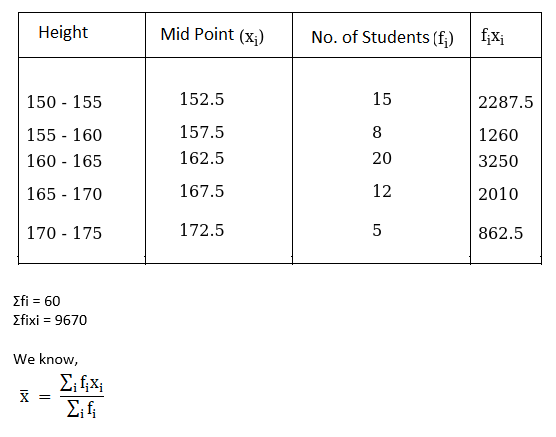= 9670/60

= 161.17

Mean is 161.17

Thus, 161.7 cm is the average height of all the students.

Question 4: Find the mode of the following distribution:

 Class interval 10-14 14-18 18-22 22-26 26-30 30-34 34-38 38-42 Frequency 8 6 11 20 25 22 10 4

Solution:

Here:

Maximum class frequency = 25

The class corresponding to Maximum class frequency is the modal class.

So, Modal class = 26-30

Lower limit = l = 26

Modal class size =h = 4

Frequency of class preceding the modal class =f0 = 20

Frequency of the modal class= f1 = 22

Frequency of class succeeding the modal =f2= 12

Mode formula is given by,

$Mode = I +\left ( \frac{f_{1}-f_{0}}{2f_{1}-f_{0}-f_{2}} \right )\times h$ $Mode = 26 +\left ( \frac{25-20}{2\times 25-20-22} \right )\times 4$

= 26 + 5/8 x 4

= 28.5

Question 5: Given below is the distribution of total household expenditure of 200 manual workers in a city:

 Expenditure (in Rs) 1000-1500 1500-2000 2000-2500 2500-3000 3000-3500 3500-4000 4000-4500 4500-5000 No. of manual workers 24 40 31 28 32 23 17 5

Find the expenditure done by maximum number of manual workers.

Solution:

Here:

Maximum class frequency = 40

The class corresponding to Maximum class frequency is the modal class.

So, Modal class = 1500-2000

Lower limit = l = 1500

Modal class size =h = 500

Frequency of class preceding the modal class =f0 = 24

Frequency of the modal class= f1 = 40

Frequency of class succeeding the modal =f2= 31

Mode formula is given by,

$Mode = I +\left ( \frac{f_{1}-f_{0}}{2f_{1}-f_{0}-f_{2}} \right )\times h$ $Mode = 1500 +\left ( \frac{40-24}{2\times 40-24-31} \right )\times 10$

= 1500 + 16/25 x 500

= 1820

## Exercise 18D

Question 1: Find the mean, mode and median of the following frequency distribution.

 Class 0−10 10−20 20−30 30−40 40−50 50−60 60−70 Frequency 4 4 7 10 12 8 5

Solution:

Let assumed mean be 35, h = 10.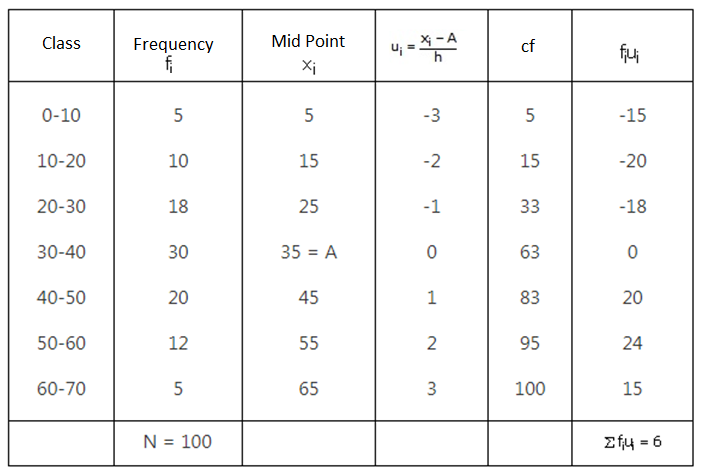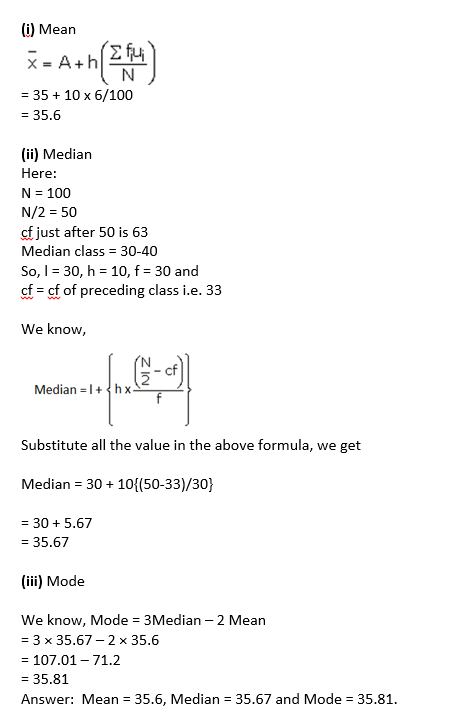Question 2: Find the mean, mode and median of the following data:

 Class 0−20 20−40 40−60 60−80 80−100 100−120 120−140 Frequency 6 8 10 12 6 5 3

Solution: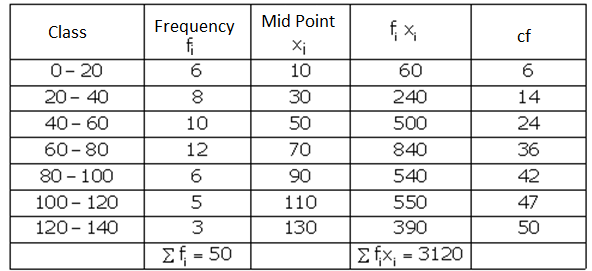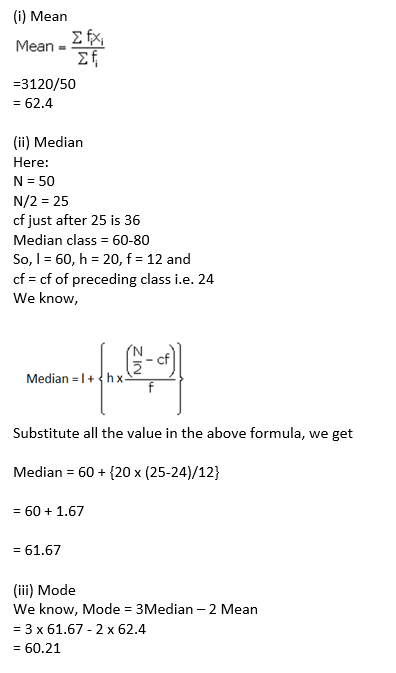Question 3: Find the mean, median and mode of the following data:

 Class 0−50 50−100 100−150 150−200 200−250 250−300 300−350 Frequency 2 3 5 6 5 3 1

Solution: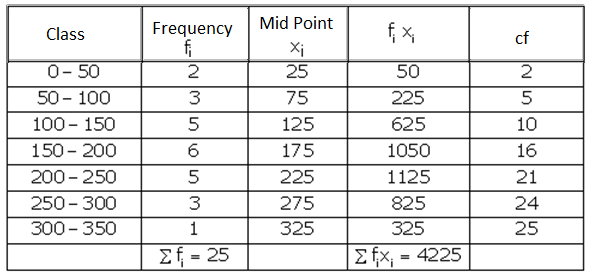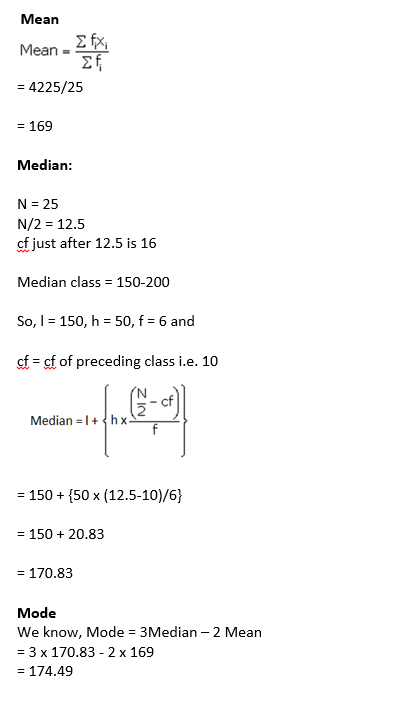Question 4: Find the mode, median and mean for the following data:

 Marks obtained 25−35 35−45 45−55 55−65 65−75 75−85 Number of students 7 31 33 17 11 1

Solution: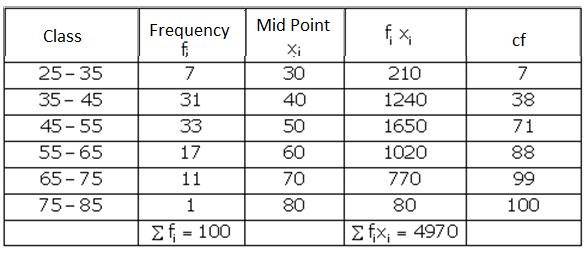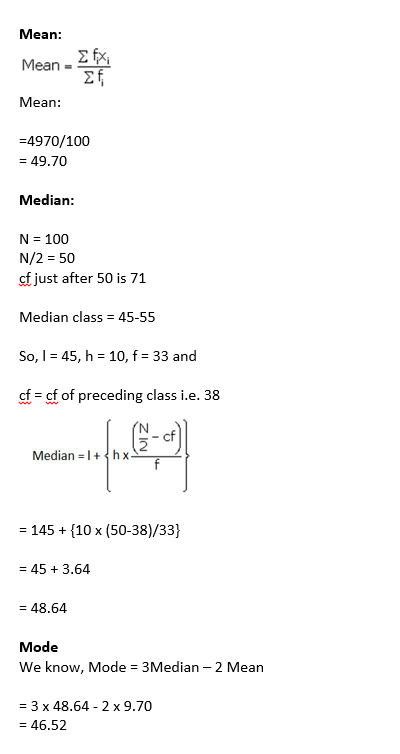Question 5: A survey regarding the heights (in cm) of 50 girls of a class was conducted and the following data was obtained:

 Height (in cm) 120-130 130-140 140-150 150-160 160-170 Total Number of girls 2 8 12 20 8 50

Find the mean, median and mode of the above data.

Solution:

Assumed mean A = 145

Class interval = h = 10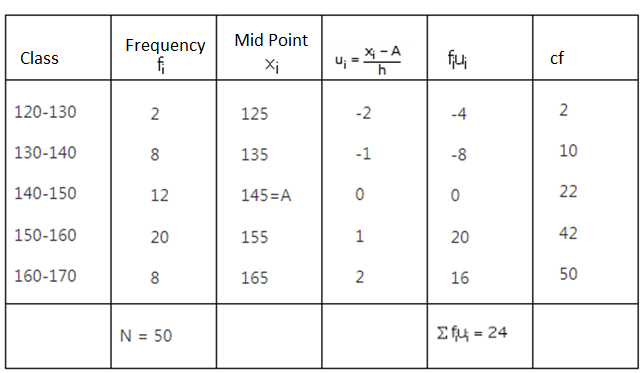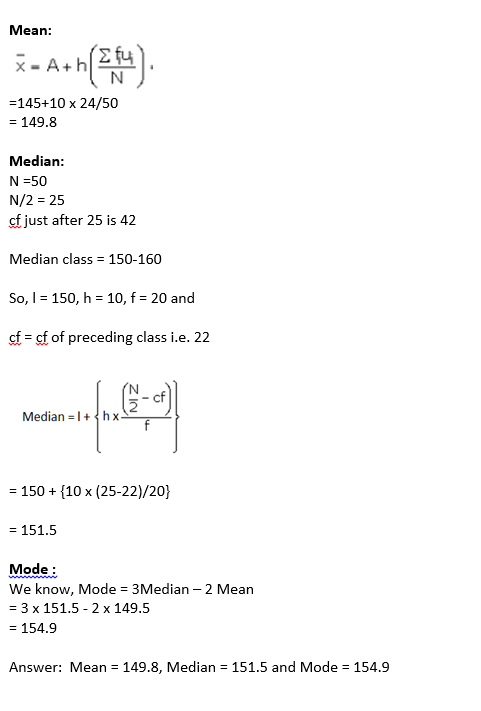Question 6: The following table gives the daily income of 50 workers of a factory:

 Daily income (in Rs 100-120 120-140 140-1 140-180 180-200 Number of workers 12 14 8 6 10

Find the mean, mode and median of the above data.

Solution: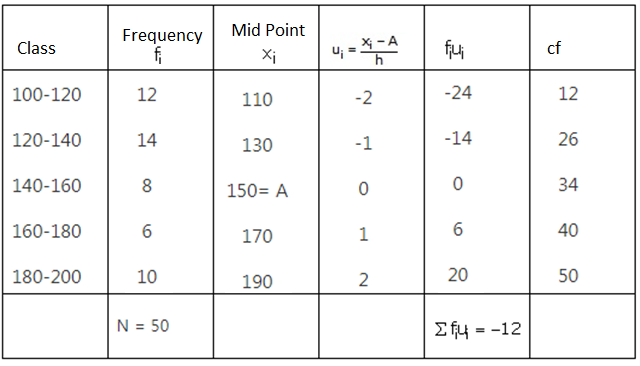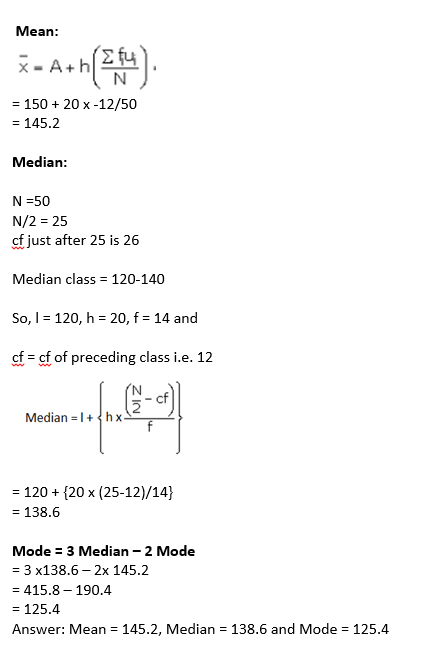Question 7: The table below shows the daily expenditure of food of 30 households in a locality: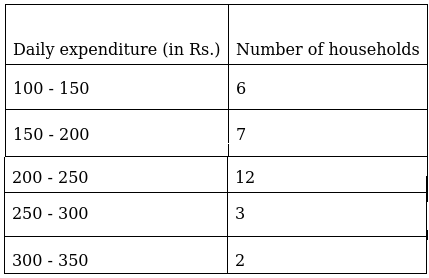Find the mean and median daily expenditure on food.

Solution:

Let assumed mean = 225 and h = 50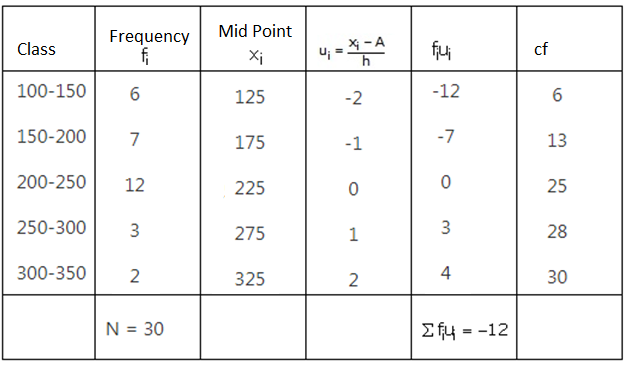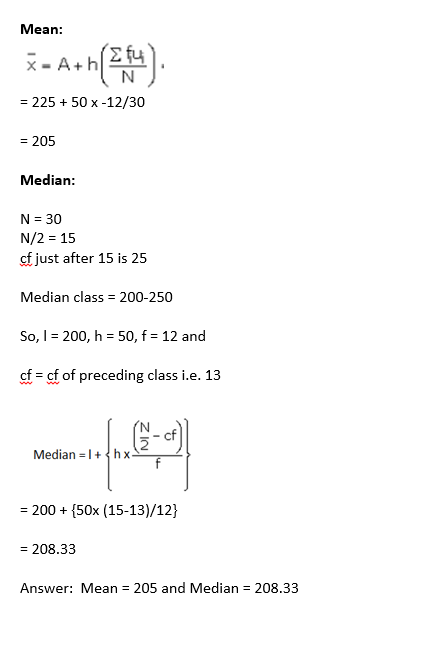## Exercise 18E

Question 1: Find the median of the following data by making a ‘less than ogive’.

 Marks 0−10 10−20 20−30 30−40 40−50 50−60 60−70 70−80 80−90 90−100 Number of students 5 3 4 3 3 4 7 9 7 8

Solution: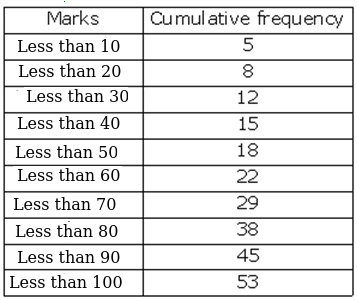Plot points: (10, 5), (20, 8), (30, 12), (40, 15), (50, 18), (60, 22), (70, 29), (80, 38), (90, 45) and (100, 53) to get the ‘less than type’ ogive as follows: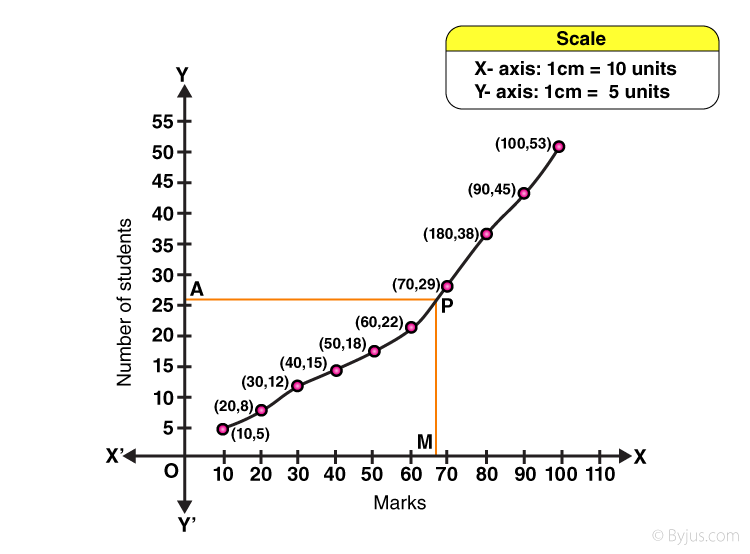From frequency table, N = 53

N/2 = 26.5

Mark y = 26.5 on y-axis

Through A, draw a horizontal line meeting the curve at P.

Through P, a vertical line is drawn which meets OX at M.

OM = 68 units

Hence, median = 68

Question 2: The given distribution shows the number of wickets taken by the bowlers in one-day international cricket matches:

 Number of wickets Less than 15 Less than 30 Less than 45 Less than 60 Less than 75 Less than 90 Less than 105 Less than 120 Number of bowlers 2 5 9 17 39 54 70 80

Draw a ‘less than type’ ogive from the above data. Find the median.

Solution:

Plot points on graph:

(15, 2), (30, 5), (45, 9), (60, 17), (75, 39), (90, 54), (105, 70) and (120, 80) to get the ‘less than type’ ogive as follows: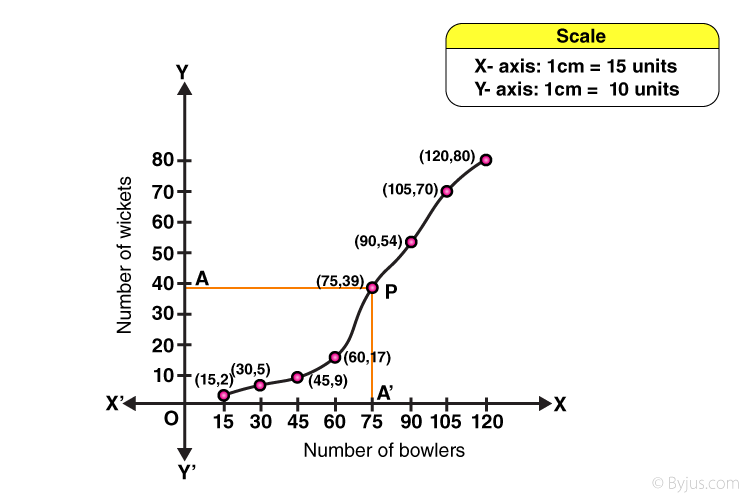From frequency table, N = 80, ⇨ N/2 = 40

At y = 40,

Draw a horizontal line meeting the curve at P, AP

Draw a vertical line from point P which is parallel to y-axis and meets OX at A’.

OM = 77 units

Median number of wickets = 77

Question 3: Draw a ‘more than’ ogive for the data given below which gives the marks of 100 students.

 Marks 0−10 10−20 20−30 30−40 40−50 50−60 60−70 70−80 Number of students 4 6 10 10 25 22 18 5

Solution: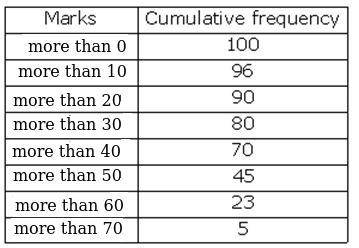Plot points on the graph:

(0, 100), (10, 96), (20, 90), (30, 80), (40, 70), (50, 45), (60, 23) and (70, 5) to get the ‘more than type’ ogive as follows: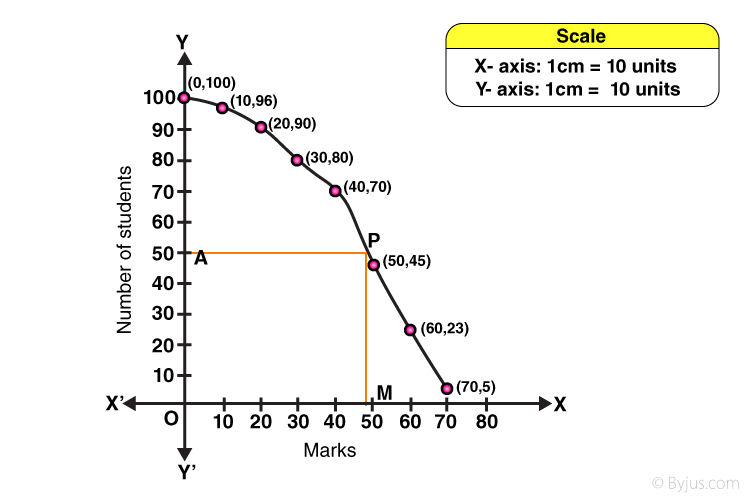From frequency table, N = 100 ⇨ N/2 = 50.

At y = 50

Draw a horizontal line meeting the curve at P, AP

Draw a vertical line from point P which is parallel to y-axis and meets OX at M.

OM = 47 units

Median marks = 47

Question 4:The height of 50 girls of class X of a school are recorded as follows:

 Height (in cm) 135−140 140−145 145−150 150−155 155−160 160−165 Number of girls 5 8 19 12 14 2

Draw a ‘more than type’ ogive for the above data.

Solution: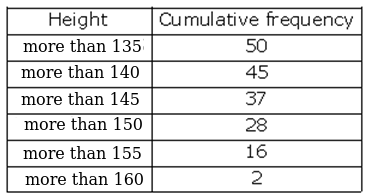Plot the points on the graph:

(135, 50), (140, 45), (145, 37), (150, 28), (155, 16) and (160, 2) to get the ‘more than type’ ogive as follows: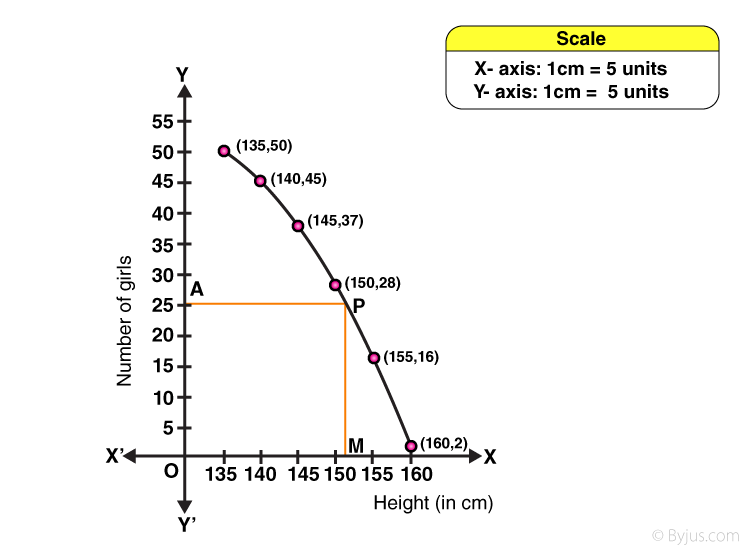From frequency table, N = 50 ⇨ N/2 = 25

At y = 25

Draw a horizontal line meeting the curve at P, AP

Draw a vertical line from point P which is parallel to y-axis and meets OX at M.

OM = 151 units

Median height = 151 cm

Question 5: The monthly consumption of electricity (in units) of some families of a locality is given in the following frequency distribution:

 Monthly consumption (in units) 140−160 160−180 180−200 200−220 220−240 240−260 260−280 Number of families 3 8 15 40 50 30 10

Prepare a ‘more than type’ ogive for the following frequency distribution.

Solution: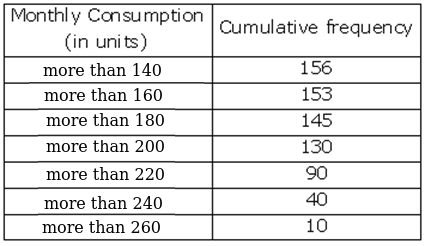Plot the points on the graph:

(140, 156), (160, 153), (180, 145), (200, 130), (220, 90), (240, 40) and (260, 10) to get the ‘more than type’ ogive as follows: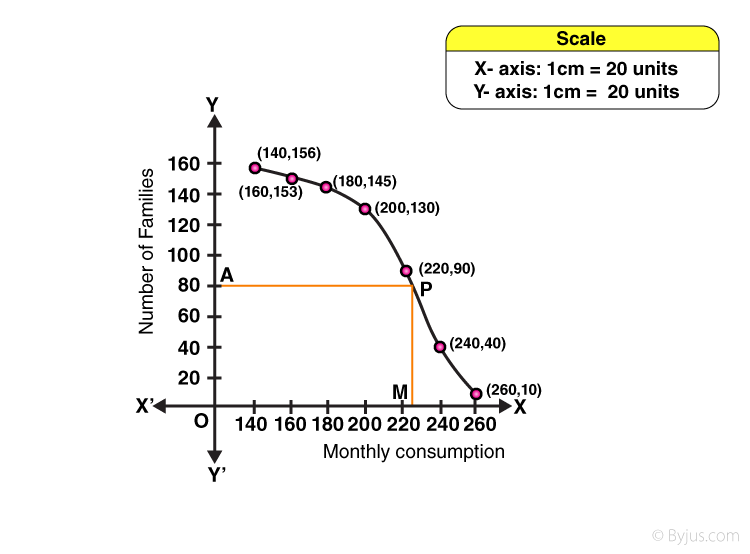From frequency table, N = 156 ⇨ N/2 = 78

At y = 78

Draw a horizontal line meeting the curve at P, AP

Draw a vertical line from point P which is parallel to y-axis and meets OX at M.

OM = 226 units

Median consumption of electricity = 226 units

## R S Aggarwal Solutions For Class 10 Maths Chapter 18 Exercises:

Get detailed solutions for all the questions listed under below exercises:

Exercise 18 A Solutions

Exercise 18 B Solutions

Exercise 18 C Solutions

Exercise 18 D Solutions

Exercise 18 E Solutions

## R S Aggarwal Solutions for Chapter 18 Mean, Median, Mode of Grouped Data, Cumulative Frequency Graph and Ogive Topics:

In this chapter students will study important concepts on Mean, Median, Mode of Grouped Data, Cumulative Frequency Graph and Ogive as listed below:

• Mean of grouped data – Direct Method
• Mean of grouped data – Assumed-Mean Method
• Mean of grouped data – Step derivation method
• Mathematical derivation of assumed mean formula
• Mathematical derivation of step derivation method
• Mean for an inclusive series
• Median for grouped data
• Mode of Grouped Data
• Measures of central tendency
• Cumulative Frequency Curve

### Key Features of R S Aggarwal Solutions for Class 10 Maths Chapter 18 Mean, Median, Mode of Grouped Data, Cumulative Frequency Graph and Ogive Topics:

1. Students can learn and practice questions answers on Mean, Median, Mode of Grouped Data, Cumulative Frequency Graph and Ogive.

2. All questions are solved by subject experts.

3. Easy for quick revision.